## Preface

This book introduces the concepts behind statistical methods used to analyze data with correlated error structures. While correlated data arise in many ways, the focus is on ecological and evolutionary data, and two types of correlations: correlations generated by the hierarchical nature of the sampling (e.g., plots sampled within sites) and correlations generated by the phylogenetic relationships among species.

The book is integrated with R code that illustrates every point. Although it is possible to read the book without the code, or work through the code without the book, they are designed to go hand-in-hand. The R code comes with the complete downloadable package of the book on leanpub.com; if you have problems downloading it, please contact me.

I’ve designed the book to be read in entirety, or at least for each chapter to be read in entirety. Therefore, it is not organized like a reference manual. However, because I don’t expect everybody to read the whole thing, I’ve tried to repeat some material between chapters, so that each chapter is more self-contained. Still, there might be places where you will want to consult another chapter, and I’ve included pointers to sections in other chapters where appropriate.

The material covered in the book is:

Chapter 1, Multiple Methods for Analyzing Hierarchical Data

The first chapter introduces and analyzes a hierarchical dataset of ruffed grouse sampled at stations (plots) within roadway routes (sites). The relationship between the chances of observing a grouse at a station and wind speed during the observation is analyzed using nine methods including linear models (LMs), generalized linear models (GLMs), linear mixed models (LLMs), and generalized linear mixed models (GLMMs). The many methods of analyzing the same dataset begs the question of which is best.

Chapter 2, Good Statistical Properties

Which method is best depends on the question and the data, and it is not always the obvious one. Chapter 2 presents the statistical tools for deciding which method is best to analyze a correlated dataset. The chapter discusses properties of statistical estimators, such as bias and precision, and the characteristics of good hypothesis tests, specifically proper type I error control and high statistical power. This is a very fast overview of mathematical statistics and then application to the grouse dataset presented in Chapter 1.

Chapter 3, Phylogenetic Comparative Methods

There is a close relationship between hierarchical data and phylogenetic data, and the same approaches can be used for their analyses. Chapter 3 employs the tools presented in Chapter 2 to evaluate common methods applied in phylogenetic analyses used to compare among species or other phylogenetic units. I also show the not-so-nice consequences of ignoring the possible correlation generated by phylogenetic relationships among species.

Chapter 4, Phylogenetic Community Ecology

Community data have both hierarchical structure (e.g., samples taken from plots nested within sites) and phylogenetic structure (e.g., related species occurring more often in the same sites). Combining methods for analyzing hierarchical data and phylogenetic data produces Phylogenetic GLMMs (PGLMMs) that are useful in a broad class of ecological community studies. This chapter uses PGLMMs to investigate different types of questions about community structure, and assesses the properties of the models. This material is only covered very technically in the primary literature, and the R packages that can perform the analyses are just being developed. Therefore, the Chapter 4 could function as a manual for the phylogenetic community models discussed.

Background you’ll need

Although the book is titled an introduction, it is an introduction to the concepts behind the methods discussed, not so much the methods themselves. It assumes that you understand basic statistical concepts (such as random variables) and know R and how to run mixed and phylogenetic models. I think that in many cases, the best way of learning is by doing. On the other hand, there is no substitute for getting a good background in the basics of statistical analyses and R before launching off into the more complicated material in this book.

R Code

R code is provided for all analyses in the book. I’ve pasted chunks of the code into the book, but I’ve left out a lot of things like formatting details, creating plots, etc. I wanted the book to be useable while running the R code but also to be readable in its own right.

Exercises

For each chapter, I have exercises that ask you to modify the code that I’ve presented to answer specific questions. All of the exercises have code for the answers that I have kept as a separate file in the downloadable R code. I’m always interested in interesting exercises, so if you have suggestions, please let me know.

References

I have used references throughout the book very lightly, mainly to refer to very specific issues. Probably more useful are the general books below. These are books I’ve used a lot, although I’m sure there are other books just as good. I’m interested in getting your recommendations for good books, so please let me know.

Efron B. and Tibshirani R. J.. 1993. An introduction to the bootstrap. Chapman and Hall, New York.

Gelman A. and Hill J. 2007. Data analysis using regression and multilevel/hierarchical models. Cambridge University Press, New York, NY.

Judge G. G., Griffiths W. E., Hill R. C., Lutkepohl H., and LeeT.-C. 1985. The theory and practice of econometrics. Second edition. John Wiley and Sons, New York.

Larsen R. J. and Marx M. L. 1981. An introduction to mathematical statistics and its applications. Prentice-Hall, Inc., Englewood Cliffs, N. J.

McCullagh P. and Nelder J. A. 1989. Generalized linear models. 2 edition. Chapman and Hall, London.

Neter J., Wasserman W., and Kutner M. H. 1989. Applied linear regression models. Richard D. Irwin, Inc., Homewood, IL.

Feedback

Please, I want and need your feedback. I wanted to self-publish this book, because it means I can update it quickly. I know it can be better than it is. I would appreciate it if you sent comments; email is the easiest way to get hold of me:

arives@wisc.edu

Acknowledgments

This book is the product of many people. The general ideas come from a class I teach at UW-Madison for graduate students, and they have all had a huge impact on how I think about and try to explain statistics. The more proximate origin of the book is a workshop I gave in 2018 at the Xishuangbanna Tropical Botanical Garden, Chinese Academy of Sciences, which followed the same outline. Participants in this workshop provided great help in honing the content and messages. I am indebted to Professors Chen Jin and Wang Bo for hosting my visit.

I also thank Li Daijiang for all of his work developing, cleaning, and speeding the communityPGLMM() code that is the main tool used for Chapter 4. I wish I had his skills. Michael Hardy also kindly allowed me to model the example used in Chapters 1 and 2 on his real dataset. Li Daijiang, Joe Phillips, Tanjona Ramiadantsoa, and Xu Fangfang provided thoughtful comments on parts or all of the manuscript, although I’m responsible for all the lingering errors.

Finally, this work has been supported by the National Science Foundation through various grants, and I am very grateful for this support.

## Chapter 1: Multiple Methods for Analyzing Hierarchical Data

### 1.1 Introduction

Many types of data encountered in ecology and evolution, or really any discipline, are hierarchical, meaning that they have a multi-level structure. An example would be a study of plant communities among multiple sites in which the data are collected from multiple plots within each site. Thus, the data are structured by plots within sites. Other examples might include multiple observations taken on the same individual, multiple individuals sampled in the same population, and multiple populations sampled of the same species. These types of hierarchies likely generate correlations in the data. For example, plant communities measured from different plots within the same site might be similar, because the plots (being in the same site) are more likely to share some feature, soil type for example, that is more similar between plots within the site than between plots among different sites. To be precise, the issue is not so much that plots within sites are more likely to be similar to each other. Instead, it is that we don’t know the cause of this difference. If we did, and if we included this information in a statistical model as a predictor (independent) variable, then the residual or unexplained variance would be independent among all plots, regardless of the site they are in. It is unknown correlation among residual errors that is the challenge for statistical methods. Of course, we will never know before we start an analysis whether we have all the information needed to explain any consistent similarities among plots from the same site. Therefore, we should always assume that the residual variation among plots within sites is correlated. I refer to this as the problem of “correlated data”, rather than “correlated residuals”, only because correlated data sounds better.

There are many different methods for analyzing correlated data. Here, I’m using “methods” to mean categories of models, such as Linear Models (LMs) versus Generalized Linear Models (GLMs). It might seem more natural to refer to different methods as different models (after all, they are called Linear Models, not Linear Methods). I’m going to use methods, though, because there are different models within methods; for example, two linear models could differ in the predictor variables they include.

This chapter describes nine different methods for analyzing the same dataset. Several of these methods are completely valid, and some give similar results. However, others are just wrong for the dataset. The point of this exercise is to discuss different ways to analyze correlated data, and different ways not to. The methods divide into two approaches. The first approach is to aggregate the data at the lower level of organization (e.g., aggregate plots within sites) and then to analyze the data at the higher level (e.g., analyze the site data). The second approach is to analyze all of the data at the lowest level (e.g., plots) while explicitly including its hierarchical structure (e.g., accounting for plots within sites). Here is a list of the methods used.

 Aggregated (site-level) data Linear Models (LM) Generalized Linear Models (GLM) GLM with a “quasi-distribution” Generalized Linear Mixed Models (GLMM) Hierarchical (plot-level) data LM GLM GLM with factor variables GLMM Linear Mixed Model (LMM)

This list is not exhaustive, and there are several obvious possibilities that I’ve left out.

The goals for introducing these nine methods are twofold. First, I want to introduce and compare these methods. Some, but not all, are appropriate for correlated data, and showing how the appropriate methods succeed where the inappropriate ones fail is valuable. Second, the methods will be used in Chapter 2 to illustrate the properties of statistical models.

### 1.2 Take-homes

1. Different statistical methods can give different results. This is not surprising. But sometimes different statistical methods give very similar results, even methods that you might not think are suitable for a given dataset. This might be surprising.
2. Almost all statistical algorithms used for hypothesis testing are approximations. Very often, it is not clear how good these approximations are for datasets with small sample sizes, as are often found in ecological and evolutionary studies.
3. If different methods give different results, then how do you decide which one to use? Actually, this isn’t a question I’m going to answer in this chapter; it is the topic of Chapter 2. The present chapter, though, should force you to face the question of deciding among methods.

### 1.3 Dataset

Chapter 1 uses a dataset that is modeled after data collected by Michael Hardy (Forest and Wildlife Ecology, UW-Madison). Because I don’t want to use his unpublished data, the dataset I’m using is a simulated version (you’ll see how in Chapter 2). What I like about the dataset is that it is very simple, yet not trivial to analyze.

The dataset consists of surveying ruffed grouse, a common game bird, at stations (plots) within roadside routes (sites) in Wisconsin, USA. In May, 2014, Michael and collaborators surveyed 50 roadway routes during a 18-day sampling period. Each route included up to eight stations spaced at 0.8 km intervals. At each station, one observer spent four minutes watching and listening for ruffed grouse. At the end of the observation period, wind speed was recorded, because the researchers suspected that wind speed would affect the chances of detecting a grouse. At each station, ruffed grouse were scored as either present or absent, because it was difficult to determine whether repeated observations were from the same or different birds (most of the time birds were just heard). To simplify things, we can assume that each observer was equally able to detect birds; in reality, this was tested by having different observers sample the same sites, but I’ve simplified the data to have only one sample per site. Finally, the distances between routes were much larger than the distances among stations within routes.

The question we will ask is whether there is a negative effect of wind speed during the observation period on the chances of detecting a ruffed grouse. The challenges for the analyses are twofold. First, the dataset is clearly hierarchical, with observations taken at stations within routes. It would not be surprising if the overall chances of observing ruffed grouse was greater in some routes than others. For example, some routes likely ran through better ruffed grouse habitat than others (although habitat characteristics were not recorded). Therefore, stations within routes might be more or less likely as a group to be scored as having ruffed grouse. Hence, the data from stations within routes are likely to be correlated. Second, the data at each station consist of only whether or not a ruffed grouse was observed. Thus, the response variable at the level of stations is binary.

Let’s read the data into R as a data.frame and take a look at them.

The main thing to see in the data is that stations are nested within routes. The variable GROUSE is the presence or absence of ruffed grouse, scored as a binary 1 or 0, respectively. Finally, WIND was observed at the station level. This becomes important later on.

For both exploring the data and performing the route-level analyses, it is necessary to generate a data.frame that aggregates data from STATION within the same ROUTE:

These data can be plotted in different ways, as illustrated in figure 1.1. The first thing to note is that there is a lot of route-to-route variation in the proportion of stations in which grouse were found. In the map of latitude versus longitude, it looks like there might be some spatial patterns, with nearby routes having either more or fewer grouse than more distant routes. I did check for spatial correlations in the real data, and they were very weak. In the present, simulated data, they are non-existent. As an aside, did it look to you like there was spatial autocorrelation? Many people have said so. I think this is just a reflection of how the human mind works: we are very good at seeing patterns, even when patterns don’t exist. The final thing from the figures is that there does seem to be a negative effect of MEAN_WIND on MEAN_GROUSE. It takes statistics, though, to tell whether the pattern is real, or whether it is just the product of how the human mind works.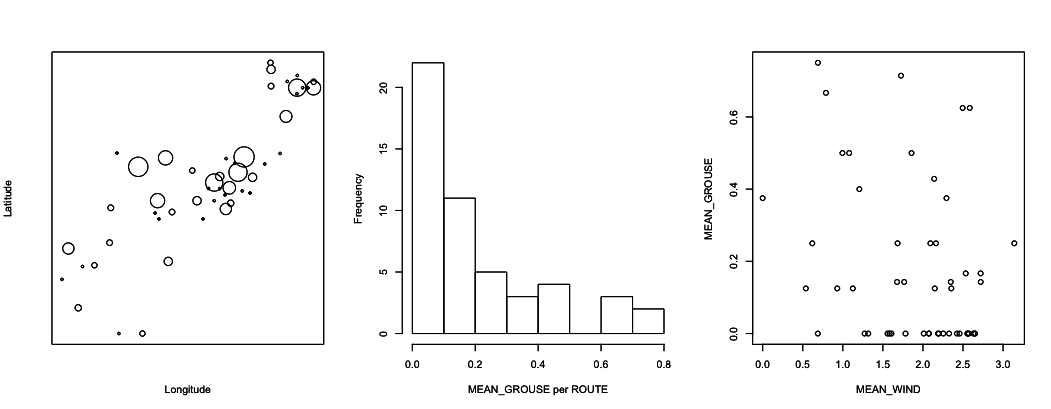Fig. 1.1: Exploration of the ruffed grouse data. The panel on the left gives a map of the routes, with the size of the circles proportional to the fraction of stations at which grouse were observed. The middle panel gives a histogram of the same information. The right panel plots for each route the fraction of stations in which grouse were observed against the mean wind speed per route.

### 1.4 Analyses of aggregated (site-level) data

The grouse data have a hierarchical structure of plots (STATIONS) nested within sites (ROUTES). The goal of the analyses is to determine whether the abundance of grouse depends on wind speed. These analyses can be performed by aggregating data among stations within routes, so that the data points consist of the number of stations within a route at which grouse were observed (from 0 to the number of stations in a route). Or the data can be analyzed at the level of stations, so that the data points are the presence or absence of grouse at each station. This section introduces the methods that aggregate the data so that each ROUTE is a data point, and the following section introduces methods that treat each STATION as a data point.

#### 1.4.1 Linear Model (LM) at the route level

The simplest approach for the route-level analysis is to use a LM, or ordinary linear least-squares regression (OLS), given by the formula

Y = b0 + b1*x + e

where Y is the number (possibly transformed) of stations at which grouse are observed in a route, x is the predictor variable (MEAN_WIND), b0 and b1 are regression coefficients, and e is the error term. You might immediately cry that this model is inappropriate, because it assumes that the errors e are normally distributed. In fact, OLS gives pretty accurate P-values for the statistical significance of the regression coefficient b1 even if the residuals are not normally distributed, a fact I’ll return to in subsection 2.6.5. But statistical tests using OLS do require that the errors e be independently distributed and have the same variance. Since these data are route-level, we can assume that the errors are independent. But it is likely that the variances among routes will differ, for the following reason. Within a route, suppose there are n stations than can take values of 0 or 1 for the observation of grouse. This distribution is therefore binomial if we assume that the observations among stations within the same route are independent. (The problem of correlated errors discussed earlier involves observations from stations within versus among routes, which is different from here where the focus is on the stations within only one route, which can be independent.) If the probability of observing a grouse at a station is denoted p, then the mean of the binomial distribution of stations with grouse is n*p. Furthermore, the variance is n*p*(1 - p). This means that if you know the mean n*p, then you also know the variance, because you know n from how the data were collected. This dependence of the variance on the mean implies that if the mean of the proportion of stations with grouse depends on wind speed (b1 is not 0), then the variance of the errors e will also change with wind speed. This would violate the OLS assumption that the errors have the same variance; in other words, the errors are heteroscedastic. The classical way to try to make the error variances homogenous in LMs is to transform the response variable Y. Therefore, I have let Y be the arcsine-square-root transform of MEAN_GROUSE. Why arcsine-square-root transform? This theoretically should do the best job possible, as explained in standard mathematical statistics textbooks (e.g., Larsen and Marx 1981).

The LM is performed as:

I’ve only printed the main results of interest: the statistical significance of the effect of MEAN_WIND on MEAN_GROUSE. It is significant at the alpha-significance level of 0.05. In other words, if the model were correct, then under the null hypothesis H0:b1=0, the probability of estimating a slope with a magnitude greater than 0.13765 is 0.0438 (since this is a 2-tailed test, the test is for the slope to be either <-0.13765 or >0.13765). A possible problem is that the P-values for hypothesis testing do, strictly speaking, require that the sum of the residual errors be normally distributed. Since the errors are not normally distributed, the P-values are approximate, but with a sample size of 50 routes, the approximation will be very good. The important thing to realize is that for the P-values it is the distribution of the sum of residuals that needs to be normal, not the individual errors, and by the Central Limit Theorem the sums of the residuals will approach a normal distribution as the sample size increases. The rule of thumb is that 30 data points is enough for the sum of residuals to be very close to normal regardless of the actual distribution of the residuals provided they are independent and have the same variance.

#### 1.4.2 Binomial Generalized Linear Model (GLM) at the route level

The LM does not directly account for the data being discrete, but instead assumes that the residuals (possibly after transformation) are normal. GLMs are designed specifically for data that follow non-normal distributions. For the route-level grouse data, the obvious choice for a distribution is the binomial. A binomial GLM for the route-level data assumes that the distribution of grouse observations among routes is

Z = b0 + b1*x

p = inv.logit(Z)

Y ~ binom(n, p)

where inv.logit is the inverse-logit function and Y ~ binom(n, p) means “Y is distributed according to a binomial distribution with parameters n (number of observations) and p (probability of occurrence in each observation)”. The idea of this GLM is to take the linear relationship Z = b0 + b1*x and force Z to be between 0 and 1 using the inv.logit function. The resulting p can be treated as an estimate of the probability of observing grouse at stations within routes, which follows a binomial distribution where n gives the number stations per route. In this formulation of the binomial GLM, I’ve used the logit function as the link function to make the values of p fall between 0 and 1. Other link functions are possible, such as the probit function (McCullagh and Nelder 1989).

GLMs are sometimes touted as solutions to the problems caused by the non-normality of non-normal data. For example, the binomial GLM might be viewed as solving the problem that the data come as counts and are integers. In fact, what GLMs are really doing is prescribing a specific relationship between the variance and the mean of the predicted value of a data point. This solves the problem of heteroscedasticity in the errors, because unlike the LM, the variances are allowed to be different. But they are only allowed to be different as prescribed by the distribution. Specifically, in the binomial GLM the variance in the number of grouse observations in route i is assumed to be ni*pi*(1 - pi), where i denotes the index i = 1, 2, …, n. So, rather than the LM approach of transforming data to try to get homogeneous variances of the residuals, GLMs incorporate the anticipated variances in the fitting process itself.

Implementing a binomial GLM for grouse observations within routes is done with the following code. For the binomial GLM, it is necessary to construct as array with two columns that contain, respectively, the successes (number of stations with GROUSE) and failures (number of stations without GROUSE). This accounts for the different numbers of STATIONS among ROUTES. I have called this array SUCCESS.

Now the P-value for MEAN_WIND is highly significant. I should point out, though, that this P-value, like that for the LM when the errors are not normally distributed, is an approximation. In particular, the glm() output in R uses a Wald test which assumes that the coefficient estimate divided by its estimated standard error (i.e., the z-value) is normally distributed; this is true only in the limit as the sample size gets very large, similar to the requirement for getting P-values from the LM when errors are not normal. Another way of obtaining a P-value for MEAN_WIND is to perform a Likelihood Ratio Test (LRT) comparing the full model containing MEAN_WIND and the reduced model without. Twice the difference in log likelihoods is approximately (as sample sizes get large) chi-square distributed. In the case of these data, the P-value from the LRT is similar but not identical to the P-value from the Wald test.

An immediate lesson from this is that there are multiple ways to approximate P-values, and they don’t give identical results. Whether or not to trust either of these P-values is discussed in Chapter 2 (and the answer is do not trust them).

#### 1.4.3 Quasibinomial GLM at the route level

The binomial GLM makes the explicit assumption that the number of stations scored positive for grouse in each route follows a binomial distribution, so that if ni is the number of stations in route i and pi = inv.logit(b0 + b1*xi) is the expected chance of observing a grouse, then the mean and variance of the distribution are ni*pi and ni*pi*(1 - pi). This means that if ten routes have the same MEAN_WIND (xi), then the variance among them is expected to be ni*pi*(1 - pi). It is common in datasets like this, however, for there to be greater-than-binomial variances; this would occur if there were differences among routes that are not included in the model, such as differences in the quality of grouse habitat.

GLMs allow for greater-than-distributional variances by using “quasi-distributions”. These are not proper probability distributions, since they do not have probability distribution functions. Instead, they use a fudge factor q in the fitting process of the GLM. For the quasibinomial distribution, the variance is set to q*ni*pi*(1 - pi), so the variance is allowed to be greater than ni*pi*(1 - pi) by the amount determined by the fitted value of q. This somewhat ad hoc approach underscores my earlier comment that the main gain of GLMs over LMs is explicitly letting the variance be a function of the mean. In the binomial GLM, the variance is set to exactly (1 - pi) times the mean, while in the quasibinomial GLM, the variance is just proportional to the mean, with q*(1 - pi) being this proportion. When the variance is greater than the binomial variance ni*pi*(1 - pi), this is called overdispersion, and q is the dispersion parameter. Note that in the output of the binomial GLM in the last subsection, this dispersion parameter is taken to be 1.

The quasibinomial GLM gives:

The estimate of the dispersion parameter q is 2.47, which means that the estimated variances are 2.47 greater than expected in a binomial distribution. Acknowledging statistically that there are greater-than-binomial variances leads to a higher P-value for MEAN_WIND. This occurs because, when given the opportunity, the model estimates higher variances and consequently infers that the data contain less information about the true effect of MEAN_WIND on observing grouse. For approximating the P-value, the Wald test uses a t-value rather than a z-value, because the dispersion parameter is estimated. A LRT is not possible, because the quasibinomial is not a distribution, and therefore it has no likelihood. (More about likelihoods in Chapter 2.)

#### 1.4.4 Logit normal-binomial GLMM at the route level

GLMMs give a second way to account for possible greater-than-binomial variances in the data. The GLMM below is set up to generate a logit normal-binomial distribution.

Z = b0 + b1*x + e

p = inv.logit(Z)

Y ~ binom(n, p)

e ~ norm(0, s2)

This is very similar to the binomial GLM, but it includes an “observation-level variance” term e which follows a normal distribution with estimated variance s2 (hence giving the name logit normal-binomial distribution). In this GLMM with an observation-level random effect, the number of levels of the random effect equals the number of data points. Therefore, the observation-level random effect is not associated with the hierarchical nature of the data; indeed, an observation-level random effect would not make sense for a LMM, because it would be exactly the same as the residual variance. It does make sense for a GLMM, however, because it allows the route-to-route variance to be greater than binomial. Since this is not a typical GLMM, I’ll leave explaining exactly what a mixed model (LMM or GLMM) is until returning to them with station-level data in section 1.5.

Fitting this model with glmer() in the lme4 package (Bates et al. 2015) is done with:

I’ve shown two parts of the output, the random effects and the fixed effects. The fixed effects are just b0 and b1, and we see that the effect of MEAN_WIND is significant at the alpha = 0.05 level, but barely. The random effect is the variance in the error term e. It is greater than zero, indicating greater-than-binomial variance in the data.

#### 1.4.5 Simulation of overdispersion

Distribution-specific GLMs and GLMMs have an incredibly valuable attribute: they can be used to simulate discrete data that look like the real data. This isn’t true of the LM applied to the grouse data, because the simulated LM will give continuous values of the arcsine-square root transformed proportion of stations with grouse present. This also isn’t true of the quasibinomial GLM, since the quasibinomial is not a distribution and so can’t be simulated. But it is true of the logit normal-binomial GLMM. I’m going to take advantage of this attribute to show you overdispersion using the GLMM.

The simulation model looks exactly like the model we fit to the data:

I have simulated 1000 samples (e.g., routes), so there are lots of them. Each sample is picked from a binomial distribution with n = 8 (stations within routes). The values of x are picked from a normal distribution with mean zero and standard deviation 1. The route-level variance e has standard deviation sd.e; when sd.e = 0 there is no variance among routes beyond that expected from a binomial distribution, and when sd.e = 1 there is greater-than-binomial variation among routes. I have initially set b1 = 0, so there is no effect of x. This is because I first want to see how the logit normal variance generated by e affects the variance of the distribution of Y. In particular, if Y is binomially distributed, then the variance of Y should be n*p*(1 - p), where p = inv.logit(b0).

Plots of Z and Y for the cases of sd.e = 0 and 1 show how the logit normal variance increases the variance of Y. In these plots, the red lines are the values of Z and n*p for sd.e = 0. When sd.e = 0, the variance in Y is very close to the expected value, n*p*(1 - p). When sd.e > 0, the variance in Y is greater.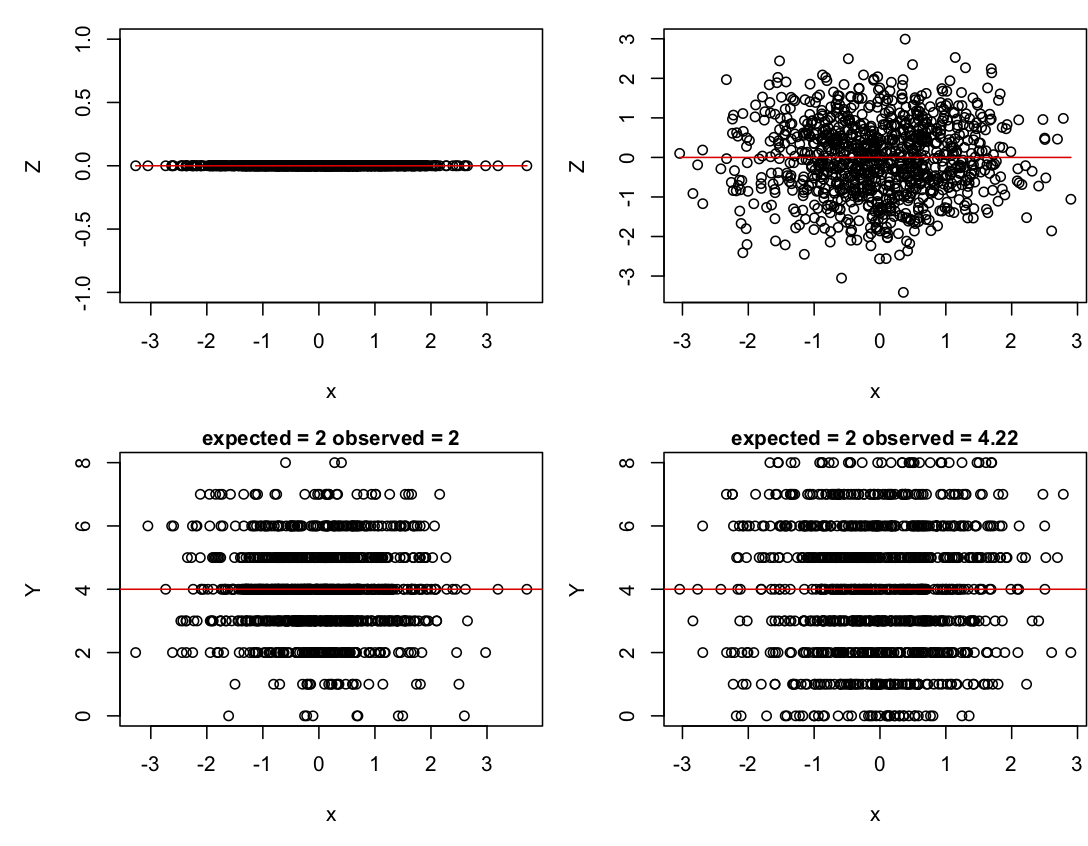Fig. 1.2: Simulations of a logit normal-binomial distribution with sd.e = 0 (left column) and sd.e = 1 (right column). The top panels give the distribution of Z, and the lower panels give the distribution of Y. Red lines give the values of b0 + b1*x (Z space) and n*inv.logit(b0 + b1*x) (data space).

If in the code you change b0 while keeping sd.e = 0, you will find that the variance of Y changes too. The variance is greatest when b0 = 0 and decreases symmetrically for smaller and larger values of b0. Finally, if you let b1 = 1 with sd.e = 0, you can see how the variance around the value of p changes with x, being greatest around p = 0.5 and decreasing as p gets smaller or larger. These changes in the variance in Y with changes in the mean are a reason for using GLMs and GLMMs.

### 1.5 Analyses of hierarchical (plot-level) data

The big difference between route and station-level data is that the station-level data are hierarchical and contain correlations, with stations within the same route more likely to be similar than stations among routes. Ignoring correlated residuals typically leads to poor and dangerous behavior of statistical tests. The first two methods below ignore this correlation structure; I use them to show the effect of correlated residuals on hypothesis tests of b1. The other three methods account for correlations but treat the binary station-level data differently.

#### 1.5.1 LM at the station level

A LM can be used to analyze the station-level data that looks very much like the LM used to analyze the route-level data (subsection 1.4.1); the difference is that the station-level data (data.frame d) are used rather than the data aggregated by routes (data.frame w). Also, the response variable Y is not transformed, because it only takes values of 0 and 1; a transformation would have no effect on the discrete nature of the observations. It might seem like a horrible mistake to use a LM that assumes continuous errors on binary data, but you should withhold judgment until Chapter 2.

The predictor variable is now WIND, rather than MEAN_WIND, since the wind speed is taken separately at each station. The resulting P-value for WIND is very low.

#### 1.5.2 GLM at the station level

The station-level GLM is similar to the GLM for route-level data (subsection 1.4.2), but with data.frame d replacing data.frame w, and WIND replacing MEAN_WIND.

A difference in the syntax arises between this and the route-level GLM. In the route-level GLM, the data are scored as successes and failures (the array w$SUCCESS), because the data are binomial counts, up to eight, of the number of stations at which a grouse was observed. In the station-level GLM, the data are the presence/absence of grouse at each station, and for binary data, successes and failures don’t need to be identified. The P-value for WIND is similar to the value obtained from the station-level LM, but a little higher. This might seem surprising, since the GLM explicitly accounts for the binary nature of the data. Doesn’t accounting for this unavoidable “error” variance (the difference between observed and predicted values) reveal the true variation in the data and consequently lead to a more powerful statistical test? Apparently not. The reason is that what the GLM is really doing is accounting for the change in the variance with the change in the mean. For binary data, the variance is just p*(1 - p) since n = 1. The binomial GLM uses this relationship when fitting the data. #### 1.5.3 GLM with ROUTE as a factor A traditional way to analyze the grouse data at the station level is to treat ROUTE as a factor which has 50 levels, with one factor for each route. Thus, the general model is Z = b0 + b1*x + b2[factor] p = inv.logit(Z) Y ~ binom(n, p) where the coefficients b2[factor] are estimated for each of the levels of the factor (ROUTE). This allows different routes to have different overall numbers of observed grouse, as we would expect if there were differences among routes. For the grouse data, 50 means must be estimated. The analysis is easy to do with the glm() function, although here I have also used the function Anova() from the car library (Fox and Weisberg 2011) because it gives a summary statistic for the overall effect of the factor ROUTE in the model, rather than reporting all 49 estimates of b2[factor] for the differences among routes. (The remaining route serves as the intercept, accounting for the total of 50 levels of the factor ROUTE.) The factor ROUTE is highly significant, indicating that routes differ a lot in the numbers of grouse observed. We also saw this information in the high dispersion parameter from the quasibinomial route-level model (subsection 1.4.3) and the high observation-level variance (random effect) in the logit normal-binomial GLMM (section 1.4.4). The P-value for WIND is much higher than it is for either of the two previous route-level models, implying that the inclusion of ROUTE as a factor absorbs a lot of the variation among routes in the number of grouse observed. This can increase the P-value of WIND by removing variation that was previous assigned to WIND in the station-level GLM without ROUTE as a factor. This might also be due to the high numbers of degrees of freedom (Df = 49 in the output above) taken up by ROUTE. This removal of degrees of freedom could weaken the statistical results for WIND; having a factor with 50 levels when there are only 372 data points is a little worrying, since this should decrease statistical power. It is possible to formulate a GLMM to overcome this possible shortcoming, which is the next method. #### 1.5.4 GLMM with ROUTE as a random effect The GLMM version of the GLM with ROUTE as a factor looks very similar: Z = b0 + b1*x + beta[factor] p = inv.logit(Z) Y ~ binom(n, p) beta[factor] ~ norm(0, s2_b*V) The difference is that rather than estimate a separate value of beta[factor] for each level of the factor, instead the model assumes that the effects associated for each level of the factor are drawn from a normal distribution with mean zero and variance s2_b. This means that, rather than estimate 49 different coefficients, instead the GLMM estimates a single variance parameter, s2_b. For the grouse data, the GLMM in effect moves the possible correlation among stations within routes from the regression parameters that are individually estimated (the fixed effects) to the variance term in the model (the random effects). This GLMM is similar to the logit normal-binomial GLMM with observation-level variance (subsection 1.4.4), except in the present case there is an accounting for the correlation among stations within routes. The correlation among stations within routes is generated by the assumption that the level of the random effect beta[factor] is the same for all stations within the same route. To explain this in more detail, I need to use the covariance matrix of the random effect. This covariance matrix gives all of the pairwise covariances among observations. Thus, if there are n data points, the covariance matrix is n by n, containing the n2 pairwise covariances; the diagonal elements are the covariances of the observations with themselves, in other words, the variances. I will denote the covariance matrix s2_b*V, where the scalar s2_b scales the magnitude of covariances contained in the matrix V. If the data are organized so that all stations within the same route are adjacent, then the GLMM with a random effect for route has a covariance matrix that is block-diagonal: the value of beta[factor] for all stations in the same route are the same, so they are perfectly correlated. Thus, for a simple case in which there are 3 routes and 4 stations per route, the V matrix would be Implementing the GLMM with ROUTE as a random effect follows familiar syntax, with the new term (1|ROUTE) giving the nested structure of stations within routes: The non-zero variance of the random effect, ROUTE (i.e., s2_b), indicates that there is covariance in the observations among stations within the same route. Also, for this model the P-value for WIND is lower than the case of the GLM with ROUTE as a factor. Since we have both the GLMM with ROUTE as a random effect and the GLM with ROUTE as a factor (subsection 1.5.3), we can compare their results. Although the GLMM estimates a variance in the random effects, it still produces estimates of the value of beta[factor] for each route. (For the algorithm used in the function glmer(), these values are computed while finding the ML parameter estimates.) The values of b2[factor] in the GLM are allowed to take on whatever values give the best fit to the number of stations with grouse observations per route. In contrast, the values of beta[factor] in the GLMM are assumed to be normally distributed which puts a constraint on their values.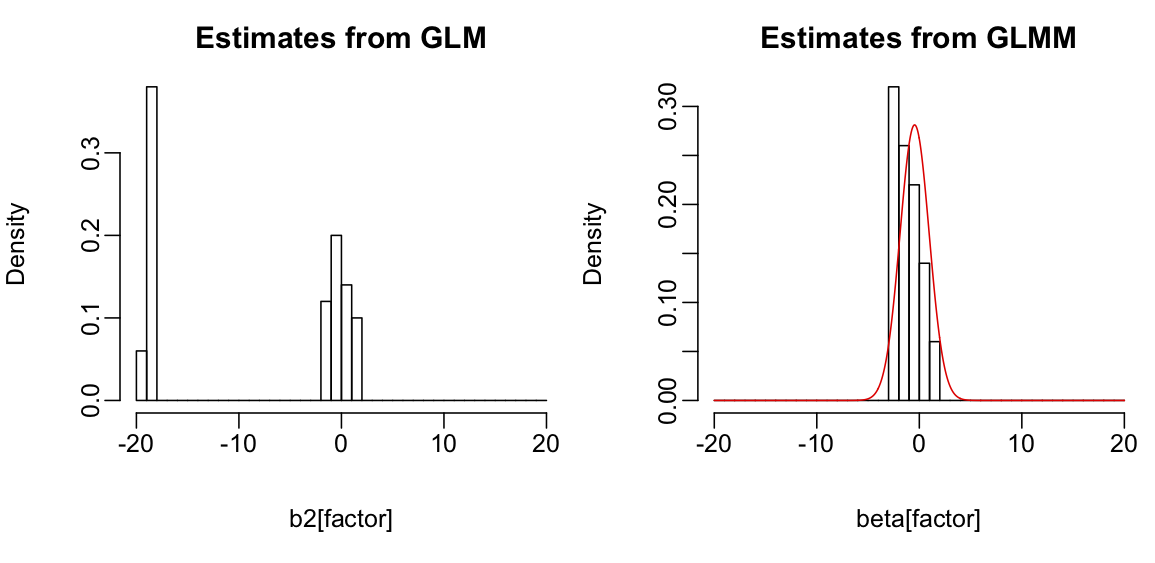Fig. 1.3: Values of b2[factor], the coefficients for each ROUTE estimated in the GLM with ROUTE as a factor (subsection 1.5.3) and values of beta[factor], the random effects in the GLMM (subsection 1.5.4). For the GLMM, the red line gives the normal distribution whose variance s2_b was fit to the data. In the right panel of figure 1.3, the random effects beta[factor] from the GLMM are clustered around the mean (the intercept, b0). In contrast, in the left panel there are 22 values of b2[factor] from the GLM with values around -20. These values correspond to the 22 routes in which no grouse was observed. Not surprisingly, the best estimates for the expected number of grouse observed in these routes (i.e., values of b2[factor]) are small, limited only by the numerical precision of my computer (these values are passed through the inv.logit function and become very small). In contrast, the assumption that the random effects of the GLMM are normally distributed pulls the random effects towards the mean, a property referred to as shrinkage or partial pooling effects (Gelman and Hill 2007, Chapter 12). For this particular dataset and statistical question, this shrinkage is reasonable, because it is unlikely that the chances of observing a grouse on the routes where none was observed during the survey are truly zero. Inclusion of these 22 routes does make a difference in the inference about WIND. If the 22 routes with no grouse observations are removed from the dataset, the GLMM estimate of the WIND coefficient is no longer statistically significant (P = 0.0816), which is similar to the GLM with ROUTE as a factor applied to the same dataset (P = 0.0966). Therefore, for the whole dataset with all routes, I suspect that the apparent loss of power in the GLM with ROUTE as a factor relative to the GLMM with ROUTE as a random effect is due to the fact that the latter model uses more of the information provided by the routes with no grouse observations; the GLMM doesn’t assume that all of the zeros are explained by ROUTE like the GLM does. Finally, note that the distribution of the random effects in the GLMM is not very normal, even though normality was assumed in the model (right panel of Fig. 1.3). This assumption was not strong enough to override the patterns in the data completely. As a final point of clarification, I want to compare the station-level GLMM discussed above with the observation-level logit normal-binomial GLMM from subsection 1.4.4. The observation-level logit normal-binomial GLMM analyzes 50 data points (one for each route) assuming that the distributions of observations within routes are binomial. Thus, the observations are the grouse counts per route. The observation-level random effect gives a different, independent value of p for each route, with the number of values equal to the number of observations (leading to the descriptor “observation-level random effect”). Because these values are assumed to the independent, the covariance matrix is s2_e*I, where I is the 50-by-50 identity matrix. In the station-level GLMM with ROUTE as a random effect (discussed above), the data are not aggregated, so there are 372 data points. Therefore, the block-diagonal covariance matrix is 372-by-372 and contains the assumption that observations from stations within routes are correlated. #### 1.5.5 LMM with ROUTE as a random effect Finally, I’ve applied a LMM to the station-level data: Y = b0 + b1*x + beta[factor] + e beta[factor] ~ norm(0, s2_b*V) e ~ norm(0, s2_e*I) The LMM is a linear model, so both the random effects beta[factor] and the error terms e have their own normal distributions. Whereas the covariance matrix for the residual error e includes the identity matrix I, the covariance matrix for the route random effect V is block-diagonal to account for the correlations among stations within the same route. To see the correlation structure, consider three cases involving station i and station j in the same route, and station k in a different route. The total covariance matrix for the model is s2_b*V + s2_e*I. To visualize this, assume s2_b = 1 and s2_e = 2. Then for the simple case of 3 routes each with 4 stations, the covariance matrix is From this, the covariance between station i and station k is 0; the covariance between station i and station j is s2_b = 1; and the covariance between station i and itself (i.e., its variance) is s2_b + s2_e = 3. Fitting the LMM to the grouse data: The variances s2_b and s2_e are the random effects. The actual values don’t really have much meaning because this LMM was fit to binary data. Nonetheless, the P-value for the effect of WIND (P = 0.0114) is close to that from the GLMM (0.01078) which is designed for binary data. More about this in Chapter 2. ### 1.6 Reiteration of results Where are we so far? We’ve discussed nine different methods and estimated the relationship between grouse observations and wind speed with each of them. Here is a summary of the results for the coefficient for WIND_MEAN in the route-level methods and WIND in the station-level methods.  Aggregated data (site-level) estimate P-value LM -0.138 0.0436 GLM(binomial) -0.494 0.0082 GLM(quasibinomial) -0.495 0.0992 GLMM -0.720 0.0496 Hierarchical data (plot-level) LM -0.056 0.0026 GLM -0.388 0.0030 GLM(ROUTE as a factor) -0.423 0.0534 GLMM -0.459 0.0108 LMM -0.050 0.0114 Some of the methods seem to be well-designed for the dataset, accounting for both the correlation of stations among routes and the binomial nature of the data: these are the route-level quasibinomial GLM and logit normal-binomial GLMM, and the station-level GLM with ROUTE as a factor and GLMM with ROUTE as a random effect. Some methods account for the correlation structure of the data but not its binomial nature: these are the route-level LM and the station-level LMM. The remaining methods don’t account for either the correlations or the binomial nature of the data. Comparing the results for the significance of the effect of wind on grouse observations, it seems that, for this particular dataset and question, accounting for correlated data makes a big difference in the significance levels given by different methods for the effect of wind on grouse observations. In contrast, whether or not the data are treated as binomial doesn’t seem to make much difference to the P-values. There is a second pattern: in the complementary pairs of station-level and route-level methods (e.g., the LM for route-level data and the LMM for station-level data), the station-level methods have greater power to detect an effect of wind on grouse observations. You might think that this is because the station-level analyses use more data. This turns out that this is not really the explanation, but that is a topic for Chapter 2. Finally, I’ve been focusing almost exclusively on the statistical significance of the regression coefficient for the effect of wind, rather than the estimated value itself. The table above shows that the analyses with LMs or LMM give estimates much lower than the GLMs and GLMMs. This is not surprising, since the estimates in the GLMs and GLMM are in terms of Z before it is logit-transformed into the probability p of observing a grouse. Therefore, the coefficients are measuring different things in LM and LMM versus GLM and GLMM models. The coefficients in the LM and LMM don’t have particularly useful interpretations; for example, fitting a LM to binary data Y will likely give predicted values of Y that are less than 0 or greater than 1. But even if the coefficients don’t have a useful meaning, statistical tests of whether they are zero (i.e., whether there is a relationship between the predictor variable and the response variable) still seem to be okay. ### 1.7 Summary 1. Different methods can give different results. We have nine different conclusions about the effects of wind speed on grouse observations. Some methods gave highly significant associations and others gave weak or no statistically significant association. And this list of nine methods is not exhaustive. 2. Almost all statistical methods used for hypothesis testing rely on approximations of one form or other. For example, even though a GLM might be designed for binomial data, the statistical tests on its coefficients are approximations; the tests are only going to give correct P-values in the limit as the sample size becomes infinite. Even though these approximations will often get better with larger sample sizes, it is generally not known how large is large enough not to worry about the accuracy of the approximations. 3. The different methods gave different results, even though we analyzed a pretty simple dataset. It is likely that many of the analyses you have done on your own data would also give different results if you had analyzed them with different methods. What can be done about this? It is often recommended that you should use the best model based upon what you know about the data. Of course you should. But this doesn’t protect you against getting wrong results (as discussed in Ives 2015, and Warton et al. 2016). Chapter 2 is designed to give you the tools for this. ### 1.8 Exercises 1. Develop and compare a LM fit to the station-level data treating route as a factor to the LMM treating route as a random effect (subsection 1.5.5). Do they give similar conclusions? Is the comparison between these LM and LMM similar to the comparison between the GLM treating route as a factor (subsection 1.5.3) and the GLMM treating route as a random effect (subsection 1.5.4)? Produce a figure like figure 1.2. Does this explain your answer in the same way figure 1.3 explains the difference between the GLM and GLMM? ### 1.9 References Bates D., Maechler M., Bolker B., Walker S. 2015. Fitting linear mixed-effects models using lme4. Journal of Statistical Software, 67(1), 1-48. Fox J. and Weisberg S. 2011. An {R} companion to applied regression, Second Edition. Sage, Thousand Oaks CA. Gelman A., Hill J. 2007. Data analysis using regression and multilevel/hierarchical models. Cambridge University Press, New York, NY. Ives A.R. 2015. For testing the significance of regression coefficients, go ahead and log-transform count data. Methods in Ecology and Evolution, 6:828-835. Larsen R.J., Marx M.L. 1981. An introduction to mathematical statistics and its applications. Inc., Englewood Cliffs, N. J, Prentice-Hall. McCullagh P., Nelder J. A. 1989. Generalized linear models. 2 edition. Chapman and Hall, London. Warton D.I., Lyons M., Stoklosa J., Ives A.R. 2016. Three points to consider when choosing a LM or GLM test for count data. Methods in Ecology and Evolution, 7:882-890. ## Chapter 2: Good Statistical Properties ### 2.1 Introduction When there are many possible methods for analyzing a dataset, it is necessary to ask which one is best. What properties should you look for in a statistical method? And how should you look? In Chapter 1 I presented a collection of nine methods for analyzing a simple dataset. Some gave the same results, and others didn’t. Which method, and hence which results, should you believe? While you might think that the best method will be the one that incorporates all of the attributes of the data – for the grouse data in particular, this would be both the correlation structure of the residuals and the binomial nature of the data. But this is a mistake. Incorporating all the attributes of the data does not guarantee that the method is best, and just because a method doesn’t have all the attributes of the data doesn’t mean it will perform poorly. Selecting a good statistical method involves two conceptually distinct issues. First, you want a statistical method that, if it really does properly describe the underlying process that generated your data, gives you the correct information about the data. You need to be aware that you can’t take this for granted. For example, just because the output from a statistical package gives you a P-value of 0.034, you shouldn’t necessarily believe this value. As I’ll show in this chapter, even very standard methods do not give you the correct P-values. On the bright side, these problems with statistical methods are relatively easy to discover and only a little more difficult to correct. Second, you want to know how misleading your statistical results might be if your model doesn’t properly describe the underlying process that generated your data. This second issue is more difficult than the first issue, because you will never really know what the process underlying your data is. Nonetheless, you can at least explore reasonable possibilities for these processes and see how your method performs. Be cautious. Although these two issues are conceptually distinct, in practice the same approaches can be used to assess each. The approaches involve simulations. For the first issue – checking on the properties of your statistical tests when you assume the model is correct – the simulations are technically referred to as parametric bootstraps. For the second issue – checking on the properties of your statistical tests when you don’t assume the model is correct – the simulations are just simulations. I use simulations a lot, and I am generally skeptical of any result until I have done simulations. In the ecological and evolutionary literature, I’m often surprised to see new methods presented without a battery of simulations to test how they work. In the statistics literature, this would not pass muster. The chapter starts with a description of the properties of estimators (functions that estimate parameters in a model) that should be used to assess how good they are. It then addresses properties of hypothesis testing involving estimators. Thus, the chapter presents:  Properties of Estimators Bias Efficiency (Precision) Consistency Properties of Hypothesis Tests Type I errors Power I’ll first present these properties using simple Ordinary Least-Squares (OLS) regression with data in which the error terms are independent and identically distributed normal random variables, because this is one of the few cases in which the statistical properties of estimators are correct. I’ll then repeat the investigation for a binary (logistic) regression problem. Finally, I’ll return to the nine methods we applied to the ruffed grouse data in Chapter 1 to try to sort out which method is best. ### 2.2 Take-homes 1. Estimators are functions of random variables that describe your data and have useful properties that lead to estimates of parameters in statistical models. Because they are functions of random variables, they are themselves random variables, and their properties – expectation, variance, behavior as sample sizes increase, etc. – determine the quality of the parameter estimates that they give. 2. Don’t trust the P-values that are reported from even standard statistical tests. Test them. If you find a problem with a statistical test, it is often relatively easy to fix the problem using parametric bootstrapping or some other approach. 3. As illustrated by the grouse data, failing to account for correlation in your data can lead to badly inflated type I errors (false positives). 4. The problem presented by discretely distributed data, or more generally non-normal data, rests mainly in the problem of heteroscedasticity. Often the problem of heteroscedasticity is not that great. 5. Hierarchical models, such as LMMs and GLMMs, are sometimes thought to have more statistical power because they use more data. For example, there are more data points in the station-level grouse data than in the route-level data. However, having more data points per se does not increase statistical power, and if you find it does in an analysis you performed, then your statistics are probably wrong. However, if a hierarchical model uses more information from the data, then it can have more power. ### 2.3 Estimators Statistical estimators are functions of random variables that have useful properties for fitting a model. To put flesh on that vague definition, suppose you perform an experiment that involves taking n observations. Each observation can be thought of as a draw from a random variable X; a random variable is just a list of possible outcomes of an experiment along with the associated probability of each outcome. For example, suppose your experiment involves flipping a coin; a process like flipping a coin that has two outcomes (heads or tails) is a Bernoulli process. The random variable X describes the possible outcomes – 1 (heads) or 0 (tails) – of each flip and the probability associated with each outcome. The entire experiment of flipping a coin n times could then be represented by the list of random variables (X1, X2, …, Xn). Once you perform the experiment, you have a specific set of outcomes (x1, x2, …, xn) that is a string of 1s and 0s; there are no longer probabilities associated with the outcomes, because you know what the flips produced. A statistic, as defined by statisticians, is a function of the n random variables (X1, X2, …, Xn). Because it is a function of random variables, a statistic is also a random variable whose mean, variance, and other attributes depend on the n random variables Xi. An estimator is a particular statistic that has useful properties for estimating the parameters of the process X. For example, a possible statistic for estimating the mean number of 1s (heads) from the coin flipping experiment is the estimator W: W = F(X1, X2, …, Xn) = sum(X1, X2, …, Xn)/n W is just a function F() that gives the expectation of the random coin flips. Notice that W can’t be computed to give a number until after you have performed the experiment; once you know the heads and tails, the outcome of W is just the average. But when discussing the properties of estimators, it is the properties of the random variable W that are discussed. In this simple example, W is designed to give estimates of the mean. Estimators can be designed to give estimates of any parameter in a model. For example, there are estimators for slopes (regression coefficients) in regression models, and also estimators for parameters that describe the variances and covariances in hierarchical and phylogenetic models. All of these estimators can be judged by the same criteria. #### 2.3.1 Maximum Likelihood (ML) estimators How do you derive a good estimator? One of the most common approaches is to derive the maximum likelihood (ML) estimator. The likelihood is the probability of observing a given set of outcomes of an experiment for specified values of the parameters in a model. The greater the probability of obtaining the observed outcome of the experiment, the better the fit of the model. The ML estimates of the parameters are those values that maximize the likelihood of the observed experimental outcome. It is relatively easy to derive the ML estimator for the coin-flipping Bernoulli process. Let p be the probability of a heads; to make things interesting, we’ll assume that p might not equal 0.5, because there could be a weighted coin. The parameter of the Bernoulli process is thus p, and p is what we want to estimate. A formal description of the Bernoulli process is Pr(X=1|p) = p Pr(X=0|p) = (1 - p) The first of these expressions states that the probability that the random variable X equals one given that the Bernoulli parameter has value p is p, and the second expression is similar. These two expressions can be collapsed into the probability distribution function f(x|p) defined as the probability that a single trial (flip) gives x = 1 or x = 0, conditioned on the value of the parameter p. Thus, f(x|p)=x*p + (1 - x)*(1 - p) You can put in values of x (1 or 0) to see that this expression works. The likelihood of the outcome of the entire experiment of n coin flips is the product of all the probabilities of the individual flips, so L(x1, x2, …, xn|p) = f(x1|p) f(x2|p) … f(xn|p) = prod(xi*p + (1 - xi)*(1 - p)) where prod is the product taken over all i = 1, 2, …, n. Taking the log to give the log likelihood function, logL, turns the products into sums, which are easier to handle mathematically: logL(x1, x2, …, xn|p) = sum(log(xi*p + (1 - xi)*(1 - p))) Since xi takes only values 1 and 0, this sum reduces to m*log(p) + (n-m)*log(1-p), where m is the number of 1s in the array x (i.e, the number of successes). To get the ML estimator of p, we want to find an expression that gives the value of p that maximizes the log likelihood (logL). Finding this maximum involves taking the derivative of the logL with respect to p and solving for that p where the derivative is zero (i.e., at the top of the peak in the logL). To illustrate this, figure 2.1 shows the logL for two experiments in which 2/10 (black line) and 6/10 flips (green line) were heads. Reading from the graph you can see in the first case that the ML estimate of p is 0.2, and in the second case it is 0.6. So the ML estimate of p is just the mean number of heads that occurred in the experiment: W = f(X1, X2, …, Xn) = sum(X1, X2, …, Xn)/n The ML estimator is what you would guess would be the best estimator based on your intuition. This is a very simple case giving a very simple estimator, but in general ML estimators often are intuitive.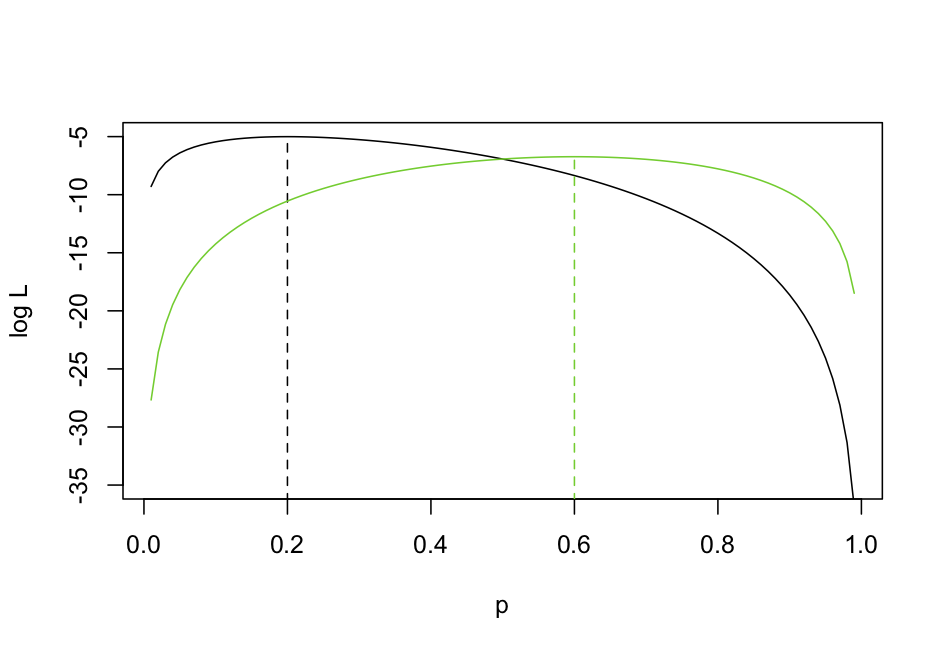Fig. 2.1: Examples of the log likelihood (logL) of a Bernoulli coin-flipping experiment for two different experimental outcomes: 2/10 heads (black line) and 6/10 heads (green line). ### 2.4 Properties of estimators Estimators have a set of properties that measure different aspects of their performance. To illustrate these properties, I’ll use the statistical problem of estimating the slope b1 in the regression model Y = b0 + b1*x + e where e is a normal random variable that is independently and identically distributed among samples. Since I want to talk about estimators in general, I will assume that the parameter being estimated is θ. In this problem, θ = b1. #### 2.4.1 Bias If an estimator for θ is unbiased, then its expectation equals the actual value of θ: E[W] = θ In other words, if you take the values of W from all possible experimental outcomes and weight them by the probability of the outcomes, then this value would be the parameter θ. This is clearly a useful property for an estimator: on average you expect its value to equal the true value of the parameter you are estimating. To test to see if the OLS estimator of b1 is unbiased, I used the function lm() with the following code. This code first specifies the number of points, n, the parameters b0 and b1 in the model, and the standard deviation of the error e. It then sets the number of simulations, nsim, and defines a data.frame to collect the results, the estimates of b0 and b1, the P-value associated with b1, and two different estimates of the variance of e (used in the next subsection on efficiency). It then iterates through nsim simulations and fits of the model each time.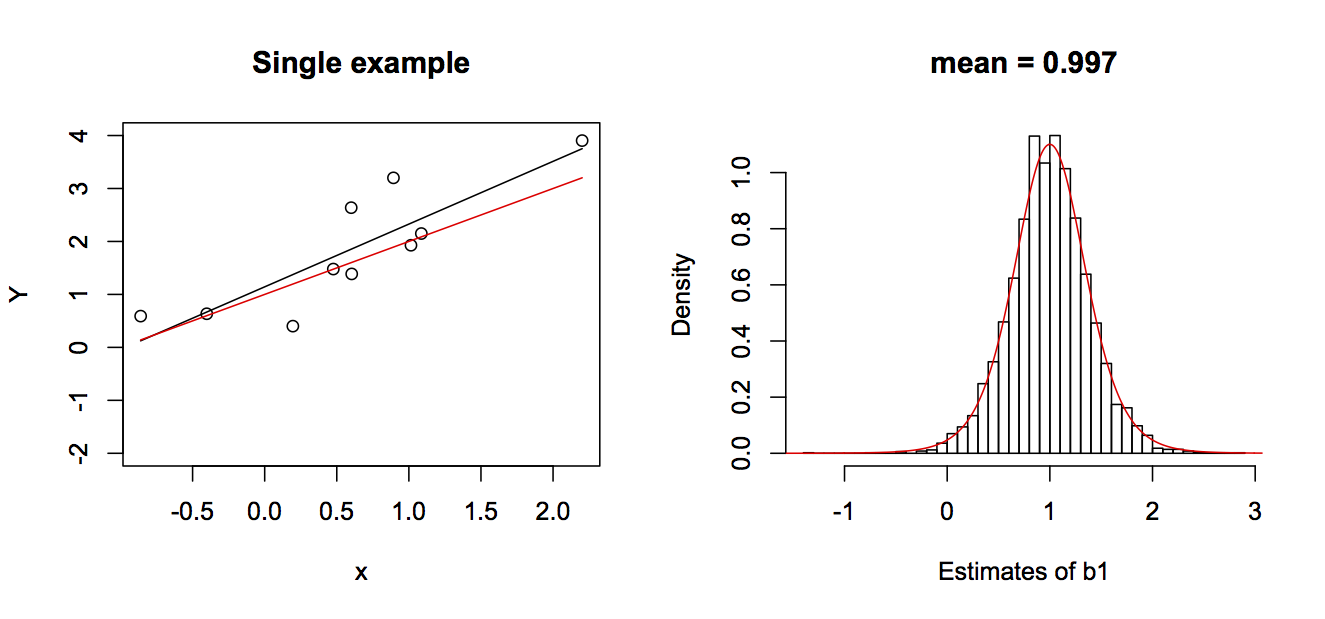Fig. 2.2: In the left panel is a single simulation of a linear regression dataset with the true line b0 + b1*x in red and the estimated line in black. The right panel gives the nsim = 5000 estimates of b1 and a t-distribution that is theoretically expected to be the distribution of the estimator of b1. The true values for the simulations were b0 = 1 and b1 = 1. The nsim = 5000 estimates of b1 are given in the right panel of figure 2.2. Note that they closely follow a t-distribution parameterized from the model parameters, which is theoretically the distribution of the estimator of b1. This shows that not only is the OLS estimator of b1 unbiased, but the theoretical distribution of the estimator is pretty accurate. #### 2.4.2 Efficiency (Precision) An efficient statistic has the lowest variance, that is, the highest precision, of any estimator. Therefore, if We is an efficient estimator, then for any other estimator W, var[We] ≤ var[W] The OLS estimator of b1 is the most efficient. In fact, it has been shown by the Gauss–Markov theorem that the OLS estimator of the coefficients of a linear regression model is BLUE, the best linear unbiased estimator, where “best” means the most efficient (Judge et al. 1985). This result doesn’t depend on the error terms being normal, or even identically distributed. But it does require that they be independent and homoscedastic. This property of OLS estimators is why OLS works so well for estimating regression coefficients. I need to make a brief detour in the discussion right now to avoid a seeming contradiction that you might have spotted. In Chapter 1 (subsection 1.4.1) I introduced the LM and said that it gave good P-values for the regression coefficients even when the data were discrete counts. This is consistent with the discussion of the efficiency of OLS in the last paragraph. However, at the end of Chapter 2 (section 1.6) the regression coefficients estimated from the LM were very different from those estimated from other methods. This is because the LM was applied to transformed data (subsection 1.4.1). The LM is still BLUE, but what it is estimating (the slope of a transformed variable) has a different meaning from a regression coefficient from a GLM (subsections 1.4.2 and 1.4.3). In the GLMs the regression coefficients give estimates of the probabilities p of observing a grouse at a station when transformed through the inv.logit link function. In contrast, the LM does not estimate a value of p. Thus, the LM applied to binomial data can give very good statistical tests for whether there is an association (i.e., whether b1 statistically differs from zero) even though the specific value of the estimate of b1 is difficult to interpret. I haven’t used simulations to show that the OLS estimator of the regression coefficients is efficient, because this isn’t very interesting; there aren’t any serious contenders. A more interesting case is the estimator of the variance s2 of e. There are two possible estimators of the variance s2. The first is the ML estimator, which is just the mean of the squared residuals. The second is the mean-squared-error (MSE). Specifically, ML estimator of s2 = (1/n)*sum((Xi - mean(X))2) MSE estimator of s2 = (1/df)*sum((Xi - mean(X))2) where df is the degrees of freedom, equal to n minus the number of regression coefficients in the model. Note that the ML and MSE estimates only differ by a constant, with the MSE estimator being (n/df) greater than the ML estimator. The values from these estimators in the simulations are shown in figure 2.3.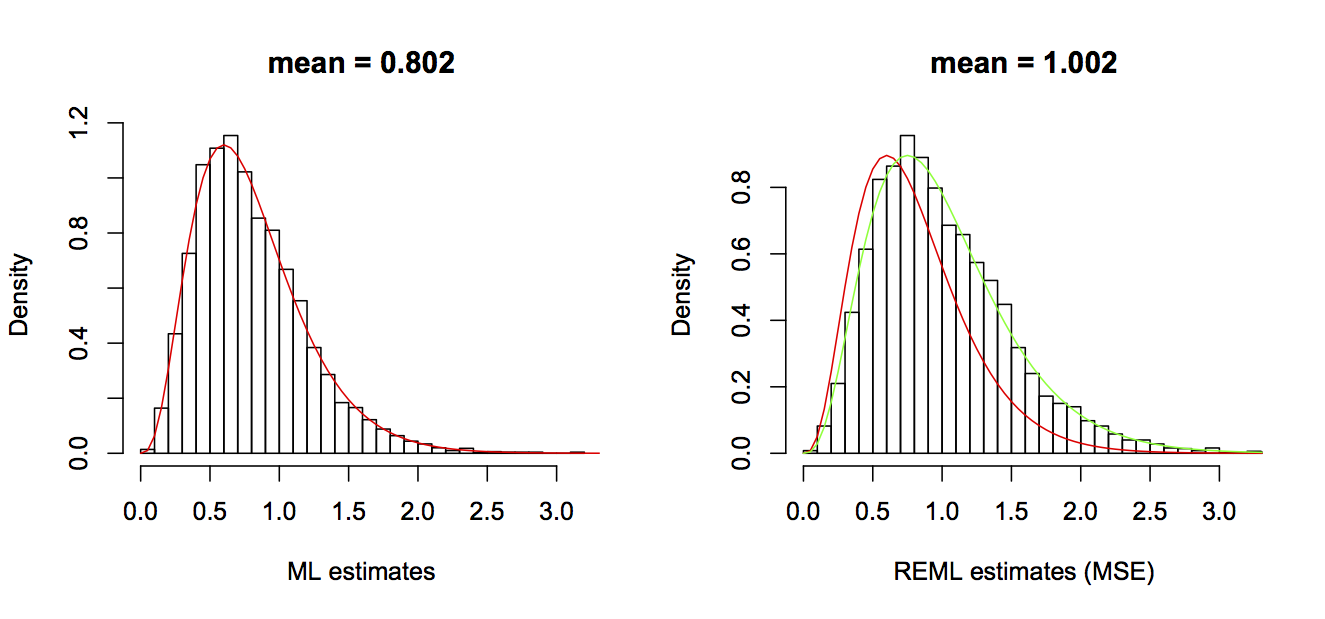Fig. 2.3: The distributions of two estimators of the variances of the error terms e in the regression model: the ML estimator (left panel) and the MSE estimator (right panel). The red line in both figures gives the theoretical distribution of the ML estimator, and the green line in the right panel gives the theoretical distribution of MSE estimator. ML and MSE estimators show that there is a trade-off in the estimation of s2. The ML estimator has higher efficiency (precision) than the MSE estimator. However, the ML estimator is biased; the expectation of the ML estimator is always below the true value by (n/df). It might seem strange that ML estimator is biased. After all, it is just computed by taking the sample variance of the residuals. The cause for this bias, though, is that in computing the variance of the residuals, the mean value (given by b0 + b1*x) has been estimated by minimizing the residuals (i.e., producing the least squares). In other words, the estimates of b0 and b1 have already been made to minimize the variance of the residuals, so it is not too surprising that the variance of the residuals is lower than the true variance of the errors. I won’t go into the details, but the estimates of the two coefficients b0 and b1 reduce the information available to estimate the variance by the equivalent of two data points (hence the reduction in degrees of freedom by 2) (for details, see Larsen, R.J. and Marx, M.L. 1981). To put this into a broader context, the MSE is the restricted maximum likelihood (REML) estimator. REML estimators are closely related to ML estimators, but they are specifically designed to estimate the variance components of a model separately from the mean components, which leads to unbiased estimates of the variances (Smyth and Verbyla 1996). In the simple case above, the variance component of the model is just the variance of the residuals, but in hierarchical and phylogenetic models, the variance components also include the covariances and hence the correlated structure of the data. The mean components of the model are the regression coefficients. The separation of mean and variance components in REML often leads to better estimates of both, although this is not always the case. #### 2.4.3 Consistency Consistency is the property that the expectation of the estimator of θ becomes arbitrarily close to the true value of θ as the sample size n becomes arbitrarily large. In other words, E[W] -> θ as n -> ∞ Even if an estimator is biased for small sample sizes, it can still be consistent. For example, the ML estimator of s2 is biased, but as n goes to infinity, it converges to the MSE and the true value of s2 (since n/df converges to one). It would be nice if not only an estimator were consistent, but also if its precision increased (variance decreased) rapidly with n. Unfortunately, the increase in precision with n is often not as fast as you might think. This is shown by plotting the standard deviation of the OLS estimator for b1 against the sample size (Fig. 2.4). Although the standard deviation drops rapidly initially when the sample sizes are small, the rate of return as sample sizes get larger diminishes, and the standard deviations come nowhere close to zero even for sample sizes of 1000. It is worth making two technical comments. First, the standard deviation of an estimator is the standard error of the estimate. Second, the standard errors of b1 are proportional to the standard deviation of the residual errors. Specifically, I ran these simulations for three values of the standard deviation of the residual errors (sd.e = 1, 2, and 3). Note that the three corresponding standard errors of the estimates of b1 are proportionally the same.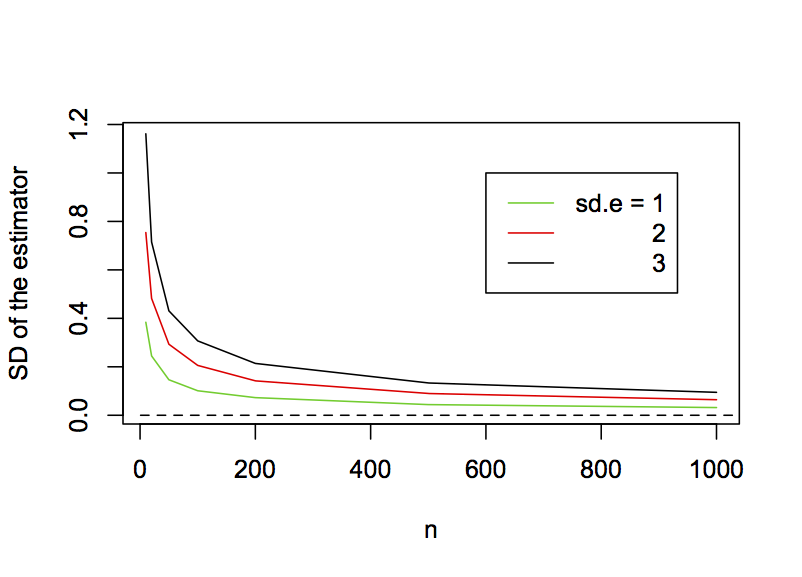Fig. 2.4: In a simple regression model, the decrease in the standard deviation of the OLS estimator of b1 as a function of the sample size for sd.e = 1, 2, and 3. ### 2.5 Hypothesis testing Hypothesis testing uses the statistical distribution of an estimator (remember, the estimator is a random variable) to infer the probability of the occurrence of a set of observations assuming some null hypothesis is correct. The test then involves claiming that the hypothesis is false if it is deemed sufficiently unlikely (the alpha significance level). This approach contains several arguments of logic. 1. There is an underlying process generating the data that could be, at least conceptually, repeated an unlimited number of times. In other words, the experimental observation is described by a random variable X that can be repeatedly sampled. 2. Our observation in the experiment is only one of these infinite number of possible realizations. 3. We can compare our observation against these realizations and from this assign a probability to what we observed. 4. If something is sufficiently unlikely under a null hypothesis, then we are comfortable saying that the hypothesis is false. In doing this, we are conceding that with probability alpha, we have made a mistake, and the null hypothesis is in fact correct even though we say that it is false. But at least we are clear about our grounds for making the call. In writing the description of hypothesis testing in the last paragraph, I have tried to emphasize the frequentist logic behind it. This contrasts with the logic behind Bayesian statistics, which is distinct from frequentist logic both conceptually and computationally. I’m only bringing up Bayesian statistics here to state that I’m not going to address Bayesian statistics in this book. This isn’t because I have anything against Bayesian statistics. In fact, even though you might hear a lot about how Bayesian and frequentist approaches are fundamentally different and one is superior to the other (with proponents on both sides), I’ve found that most statisticians use one or the other approach depending on the problem. The Bayesian framework leads to algorithms that make it possible to answer statistical problems that would be computationally too hard for frequentist algorithms; therefore, hard problems often pull researchers towards Bayesian statistics. Nonetheless, I have yet to meet a Bayesian statistician who would forgo OLS for estimating parameters for linear regression in favor of a Bayesian approach. I personally lean towards frequentist approaches because my background is in theoretical ecology, and in theoretical ecology models of real systems are designed and studied often without any attempt to fit them to data. I learned statistics to try to fit theoretical models to data. But with my background in theory, I am used to theoretical models existing before attempting to fit them to data; these theoretical models are thus “reality” that underlie the patterns we observe in nature. This makes me comfortable with the idea that there is some fundamental process, given by a model, underlying the data – the frequentist assumption. Getting back to hypothesis testing, the two key issues that make a test good are having the correct type I error rates and having good statistical power. #### 2.5.1 Type I errors The type I error rate of a statistical test is the probability that the null hypothesis is correct even though it is rejected by the test. Thus, type I errors are false positives. There is nothing wrong with false positives. In fact, they are inevitable and are explicitly set when specifying the alpha significance level of the test. For example, if the alpha significance level is P = 0.05, then there is a 0.05 chance of rejecting the null hypothesis H0 even though it is true. The problem for hypothesis tests involving type I errors is when the alpha significance level you select doesn’t give the actual type I error rate of the test. For example, if you set the alpha significance level at P = 0.05 but the actual chance of rejecting H0 is 0.10, then you run the risk of stating that a result is significant at, say P = 0.045, when in fact the true probability under H0 is 0.090. You would thus be claiming that a result is significant at the alpha significance level of 0.05 when in fact it is not. That would be bad. The correct control of type I error rates is easy to check. If you simulate data using a model for which H0 is true and fit the model to many simulated datasets, the fraction of simulated datasets for which H0 is rejected should equal the alpha significance level. The code required for doing this for the regression model is very similar to that presented for simulating the distribution of the estimator of b1 to produce figure 2.3 and 2.4, so I won’t reproduce it here (although it is in the R code for this chapter). For parameters in the models, I picked the same values as used in section 2.4. The results for an alpha significance level of 0.05 and different values of n are  n rejected 20 0.047 30 0.051 50 0.051 100 0.054 There is some variation away from the 0.05, but this is just due to the variation inherent in random simulations. I simulated 10,000 datasets, but more simulations will bring the values closer to 0.05. #### 2.5.2 Power Power depends on the chances of rejecting the null hypothesis when in fact it is wrong. It is possible to assess both the power and type I errors of a model by fitting it to simulations as the parameter θ gets increasingly removed from the null hypothesis. Figure 2.5 shows the fraction of 10,000 simulations for which H0:b1 = 0 was rejected at the alpha significance level of 0.05 for values of b1 increasing from zero. The curves for b1 decreasing from zero are the mirror image, so I haven’t shown them. The type I error rate is given when the true value of b1 is 0 (the null hypothesis). If the type I error rates are correct, then the fraction of simulations rejected when b1 = 0 should be 0.05, as they are. The steeper the rejection rate increases as b1 increases, the more powerful the test. The lines show that, as the sample size increases, the power to reject H0 increases too.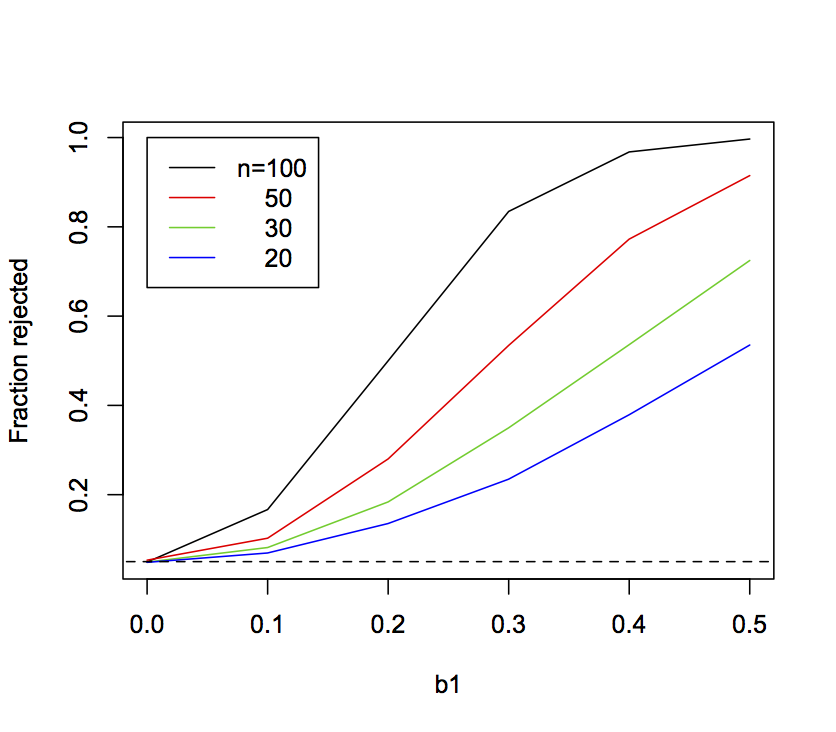Fig. 2.5: Fraction of simulations for which H0:b1=0 was rejected as the true value of b1 used in the simulations increases from zero. Data were simulated with sample sizes 20, 30, 50, and 100. The horizontal dashed line gives 0.05. In the simulations, b0 = 1 and sd.e = 1, and x was drawn from a normal distribution with mean = 0 and sd = 1. ### 2.6 P-values for binary data For hypothesis tests of b1 in the linear regression model, the P-values are computing exactly using the fact that the distribution of the estimator of b1 follows a t-distribution. For most methods, however, the exact distribution of the estimator is unknown, and instead an approximation must be used. Two standard approximations are the Wald test and the Likelihood Ratio Test (LRT). I used both of these tests in Chapter 1 with the GLM route-level model (subsection 1.4.2), but here I will go into more detail. I’ll also present two related tests: parametric bootstrapping of the parameter estimate and parametric bootstrapping of the hypothesis test. To motivate this discussion, I’ll illustrate these different approximations for P-values using a model of binary data with the form Z = b0 + b1*x1 + b2*x2 p = inv.logit(Z) Y ~ binom(1, p) where as before we are focusing on the significance of b1. I’ve added x2 and b2 (where x2 has a norm(0, 1) distribution and b2 = 1.5) because this presents more of a statistical challenge. I simulated the data assuming that x1 and x2 are independent and then fit the data with a binomial GLM. For each fitted GLM I computed the P-values for the null hypothesis H0:b1 = 0 and scored the number of datasets for which H0 was rejected based on four approaches: the Wald test, the LRT, a bootstrap of b1, and a bootstrap of H0. This procedure gives the type I errors (the fraction of simulations rejected at the alpha = 0.05 level) for the case when the true value of b1 = 0. I’ll describe the specific tests later in this section, but I want to start by contrasting their results upfront; this gives you a sense of the problems we face when selecting the best test.  n Wald LRT Boot(b1) Boot(H0) LM glm.converged 20 0.004 0.103 0.102 0.047 0.050 0.952 30 0.025 0.078 0.092 0.054 0.053 0.994 50 0.042 0.063 0.062 0.055 0.054 1.000 100 0.046 0.055 0.074 0.049 0.049 1.000 Obviously, the standard Wald and LRT tests have poor type I error control when sample sizes are small, with the Wald test P-values being far too high (so that rejections occur rarely) and the LRT P-values being far too low (so that rejections occur too frequently). Remember, these are both standard tests, and GLMs are supposed to be appropriate especially for small sample sizes. The parametric bootstrap of the estimated parameter (the column “Boot(b1)”) showed inflated type I errors, with more simulated datasets rejected than the nominal alpha significance level. The parametric bootstrap of the null hypothesis H0 (the column “Boot(H0)”) worked pretty well, giving type I errors of close to 0.05. For comparison, I also fit a LM to the same data, with the P-value for b1 based on the standard t-test. The LM takes no account of the binary nature of the data, so the actual values of b1 are hard to interpret; for example, the predicted values for Y from the LM can be less than zero or greater than one, even though the values of Y can only be zero or one. Nonetheless, as a test of association (whether b1 is different from zero), the LM did surprisingly well, giving appropriate Type I errors. There is a small complication in these results, because the function glm() used to fit the GLM didn’t converge for some datasets with small sample sizes. The column labeled “glm.converged” is the fraction of datasets for which convergence was reached. When doing simulations, one could argue for including these cases (lack of convergence could occur with real data) or excluding them (if glm() failed to converge, you would try another method). In this simulation, the P-values either way were not that different, so I only present the results for the cases for which glm() converged. Finally, there is greater variability in the reported rejection rates for the parametric bootstrap than for the other methods, because I ran fewer simulations. To match the other methods, each of the 10,000 simulations would require 10,000 bootstraps, or a total of 100,000,000 simulations. Therefore, I ran 500 simulations and 1000 bootstrap simulations for each to give the parametric bootstrap of the estimate. Below I present in more detail the different GLM tests of the null hypothesis H0:b1 = 0 given in the table. #### 2.6.1 Wald test The Wald test approximates the standard error of an estimate and then assumes that the estimator is normally distributed with standard deviation equal to the standard error. The Wald test can go wrong in three ways. First, the estimate of the standard error could be wrong. Second, the estimator might not be normally distributed. Third, the estimator could be biased. All three of these problems greatly diminish with large enough sample sizes, but it is generally impossible to guess how large a sample size is large enough. We’ll see the problems with the Wald test approximation when applying the parametric bootstrap (subsection 2.6.3). #### 2.6.2 Likelihood Ratio Test (LRT) The LRT is a very general and useful test. It is based on the change in log likelihood between a full model and a reduced model, where the reduced model has one or more parameters missing. For the example, the reduced GLM model used for testing the significance of b1 is the model excluding x1. If x1 is important for the fit of the full model to the data, then there should be a large drop in the logL when x1 is removed, since the data are less likely to be observed. This drop is measured by the log likelihood ratio (LLR), the difference between logLs of full and reduce models. As the sample size increases, 2*LLR approaches a chi-square distribution; for GLMs, 2*LLR is referred to as the deviance. This gives the probability of the change in logL if in fact the reduce model was the true model (i.e., b1 = 0) and hence the statistical test for H0. A particularly useful attribute of the LRT is that more than one parameter can be removed, so joint hypotheses about multiple parameters can be tested. The problem with the test, however, is that the chi-square distribution is only an approximation, and the approximation for our example problem is not very good for even a sample size of 50, as shown in the table above. #### 2.6.3 Parametric bootstrap of b1 A parametric bootstrap is simple to apply and often very informative; many packages (such as lme4 and boot) provide easy tools to perform a parametric bootstrap. The idea is simple (Efron and Tibshirani 1993): 1. Fit the model to the data. 2. Using the estimated parameters, simulate a large number of datasets. 3. Refit the model to each simulated dataset. 4. The resulting distribution of parameters estimated from the simulated datasets approximates the distribution of the estimator, allowing P-values to be calculated. To show an example with a single dataset, I simulated a dataset with n = 30 and b1 = 1. I fit the model (mod.dat in the code below) that gave an estimated value of b1 = 1.79 (even though the true value was 1; this was not an unusual simulation, though). I then computed the bootstrap P-value: I used the R function simulate() that takes a model and simulates it for its fitted parameters (which in this case is b1 = 1.79). The simulated data are then refit with a model having the same form as mod.dat. I made the decision only to use bootstrap simulations for which glm() converged. In the bootstrap, the P-value is the proportion of bootstrap values of b1 that extend beyond zero, multiplied by 2 because we are only interested in whether b1 is more extreme than zero regardless of its sign (i.e., a two-tailed test). To explain the code, mean(boot$b1 < 0) is the proportion (mean) of the bootstrapped values of b1 that is less than zero. If the estimate of b1 is greater than zero, then this proportion will be less than 0.5. Therefore, it will be selected by the min() function. This is thus the probability of that the true value of b1 is less than zero, conditioned on the fitted value being greater than zero. Because the fitted value of b1 could have been less than zero, it is necessary to multiply by 2, so that the P-value gives the unconditional probability that the fitted b1 is greater than or less than zero.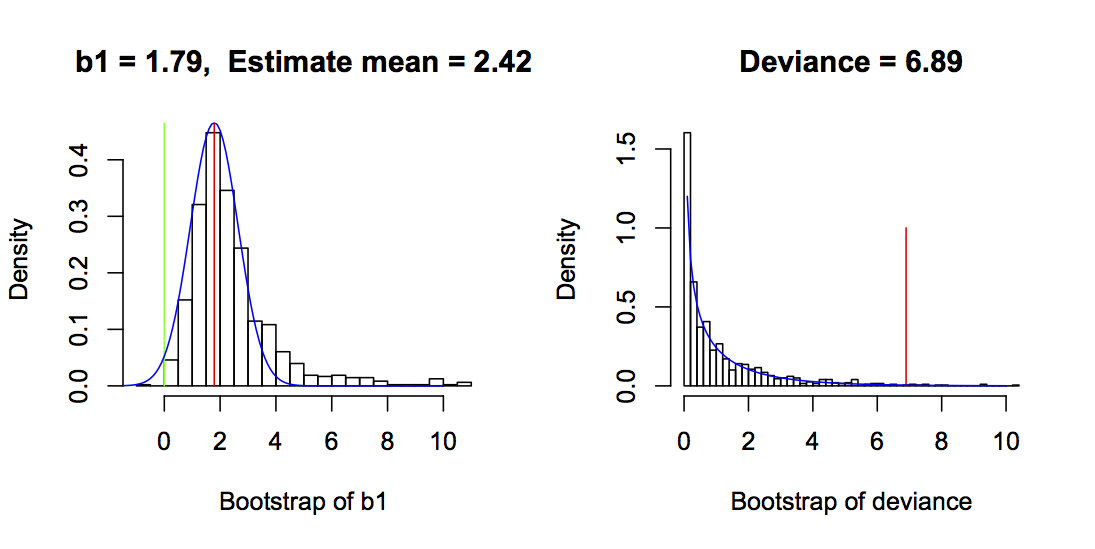Fig. 2.6: Bootstrap values from the parametric bootstrap and parametric bootstrap of H0. The left panel gives the bootstrap values of b1 from the parametric bootstrap, with the red line giving the value of b1 from the fitted dataset. The P-value is computed as 2 times the proportion of bootstrap b1 values less than zero; here, P = 0.014. The normal distribution of the estimator of b1 used for the Wald test is given by the blue line, and the P-value from the Wald test is 0.037. The right panel gives the bootstrap values of the deviance (= 2*LLR) for the parametric bootstrap around H0 for the same dataset. The red line is the deviance from the fitted data, and the P-value is 0.011. The green line is at zero. The chi-square distribution from a standard LRT is shown in blue, and the LRT P-value is 0.0086. In both bootstraps, bootstrap datasets for which glm() failed to converge are excluded, and for the parametric bootstrap of b1, values >2.5 times the standard deviation of the estimates are excluded. Other parameters are b0 = 1, b2 = 1.5, the variances of x1 and x2 are 1, and x1 and x2 are independent.

The left panel of figure 2.6 gives an example of the bootstrap of b1 and reveals several interesting things about the GLM estimator and the Wald test. First, even though the bootstrap simulations were performed with a model in which b1 = 1.79 (the fitted value), the mean of the bootstrapped estimates was 2.42. This shows that the GLM estimator of b1 is biased upwards. Second, the distribution of the estimator is skewed away from zero. In contrast to the bootstrap estimator of b1, the Wald estimator (blue line) is normal and symmetrical. These differences lead to different P-values, with the parametric bootstrap giving P = 0.002 and the Wald test giving P = 0.037.

These results were generated for a single dataset, yet they are typical of the performance of the bootstrap of b1 and the Wald test. The table at the top of this section shows the rejection rates of the null hypothesis H0:b1 = 0 for a large number of simulated datasets. This table shows that the bootstrap of b1 generally gives P-values that are too low, since the proportion of datasets rejected at the 0.05 level is greater than 5%. It also shows that the Wald P-values are too high, since the proportion of datasets rejected at the 0.05 level is less than 5%. Figure 2.6 is just a single example, and H0:b1 = 0 was rejected by both bootstrap and Wald tests. Nonetheless, the much lower P-value for the bootstrap test of b1 appears to be general for this binary regression model.

#### 2.6.4 Parametric bootstrap of H0

A parametric bootstrap of the null hypothesis H0 is very similar to the parametric bootstrap of the parameter b1, but the data are simulated under H0:

1. Fit the model to the data and calculate the deviance (= 2*LLR) between the full model and the reduced model without b1.
2. Using the estimated parameters from the reduced model, simulate a large number of datasets.
3. Refit both the full and reduced models for each dataset and calculate the deviance.
4. The resulting distribution of deviances estimated from the simulated datasets approximates the distribution of the estimator of the deviance, allowing P-values to be calculated.

This is closely related to the LRT, although rather than assume that the deviances are distributed by a chi-square distribution, the distribution of the deviances is bootstrapped.

In figure 2.6, the bootstrapped deviances are similar to the chi-square distribution used in the LRT, although the tail of the bootstrap distribution is wider and longer. This gives the bootstrap P = 0.011, while for the LRT the P-value is 0.0086. The lower P-value for the LRT is consistent with the table at the beginning of this section, in which the LRT gave inflated type I error rates. The good performance of the parametric bootstrap of H0 can be understood by considering the distribution of the estimates of b1. While these estimates aren’t used for the significance test, the distribution of the estimates is symmetrical and therefore necessarily unbiased because the bootstrap simulations are performed under H0:b1 = 0. The better behavior of the estimator of b1 under H0 explains the better performance of the parametric bootstrap of H0 compared to the parametric bootstrap of the parameter b1 (subsection 2.6.3).

#### 2.6.5 Why did the LM do so well?

I included the LM in the table at the top of this section to show that in fact it does as well or better than any of the other methods, at least in terms of type I error. In some respects, this is not surprising. As I discussed in subsection 2.4.2, the OLS estimator of LMs is BLUE (best linear unbiased estimator), provided the errors are independent and homoscedastic. They are independent in the binary model analyzed here, but are they homoscedastic? If this were a univariate binary regression model with only x1, then under the null hypothesis that b1 = 0, they will be homoscedastic, because under H0 there is no variation in the mean, which is determined by b0. With no variation in the mean, there is also no variation in the variance. In the model, however, there is x2. If x2 changes the mean (b2 ≠ 0), then this will generate heteroscedasticity, but if x1 and x2 are independent, then this heteroscedasticity will have no effect on type I errors for the estimate of b1, because the differences in the variances among errors will be independent of x1. However, I would anticipate a problem with heteroscedasticity for the case when b2 > 0, and x1 and x2 are correlated, because this should produce a gradient in heteroscedasticity of residuals across values of x1. But it turns out for the binary model that even this case does not degrade the good type I error control. Figure 2.7 shows an example of the distribution of residuals with b1 = 1.5 and a covariance of 0.8 between x1 and x2 using a large enough sample size (n = 1000) to allow assessment of heteroscedasticity of the residuals. There is some, with the variance in residuals decreasing with increasing b1. However, it is not great, and apparently not great enough to badly degrade the type I error control of the LM. For other families of distributions used in GLMs, such as the binomial distribution with n ≥ 2 or the Poisson distribution, this might not be the case, and the combination of b2 > 0 and correlation between x1 and x2 could cause inflated type I errors; I’ve left this as an exercise. At least for the binary case – the case that is least like continuous data – the LM does well.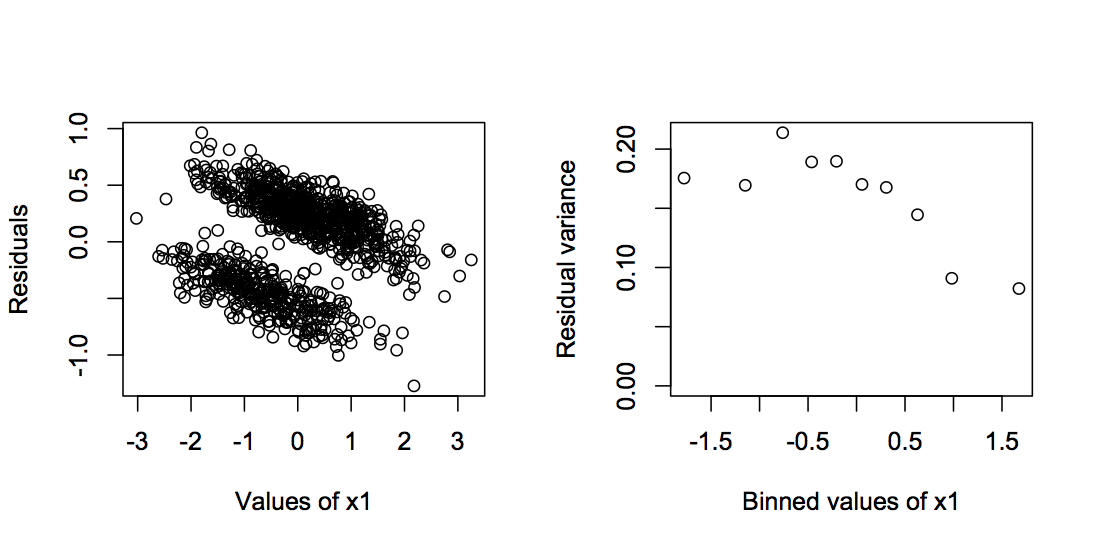Fig. 2.7: Residuals of a LM fit to binary data with n = 1000, b0 = 1, b1 = 0, b2 = 1.5, the variances of x1 and x2, var.x = 1, and the covariance between x1 and x2, cov.x = 0.8.

#### 2.6.6 Power in fitting the binary data

The power and type I error rates of the four methods for computing P-values from the binary GLM, plus the LM, can be shown (as in figure 2.5) by plotting the rejection rate of simulated datasets against the value of b1 used in the simulations. Figure 2.8 repeats the results that the Wald test gives deflated type I errors, the LRT and parametric bootstrap of b1 give inflated type I errors, and the parametric bootstrap of H0 and the LM give pretty good type I errors. Type I errors have to be correct before it makes sense to investigate power; otherwise, you could always increase the power of your test by increasing the type I error rate. Therefore, I shouldn’t really show the power curves for the LRT and parametric bootstrap of b1. Nonetheless, I have. The power of the parametric bootstrap of H0 and the LM are almost identical. Not surprisingly given its lower type I error rates, the Wald test has less power. And while the LRT and parametric bootstrap of b1 appear to have higher power, they shouldn’t be used in the first place due to inflated type I errors.

I left out one good method for performing regressions on binary data: logistic regression using a penalized likelihood function proposed by Firth (1993). I have left you to explore this as an exercise.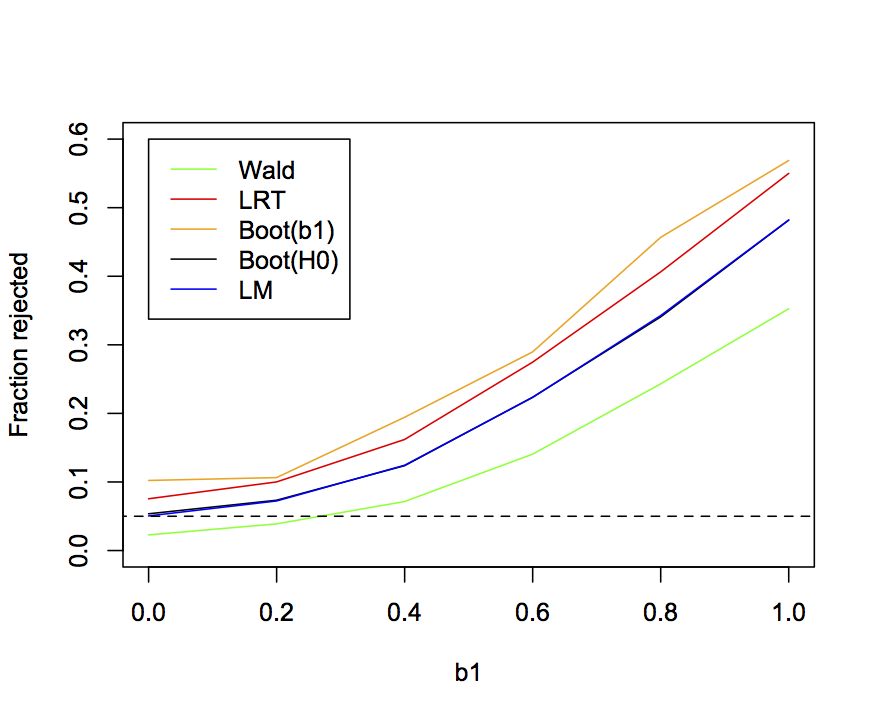Fig. 2.8: Curves showing the proportion of simulations rejected by four methods for computing P-values with the GLM, and for the LM. Parameter values are n = 30, b0 = 1, b2 = 1.5, var.x = 1, and cov.x = 0.

### 2.7 Example data: grouse

We are finally in position to return to the grouse data to ask which methods for analyzing the data are best. For this, I will assess the methods based on their type I error control and their power, using the same approach I used for the linear regression model and binary GLM investigated in the last two sections. As a reminder, here are the different methods and the results they give for the effect of wind speed (WIND_MEAN in the route-level methods and WIND in the station-level methods) on the observed abundance of grouse:

 Aggregated data (site-level) estimate P-value LM -0.138 0.0436 GLM(binomial) -0.494 0.0082 GLM(quasibinomial) -0.495 0.0992 GLMM -0.720 0.0496 Hierarchical data (plot-level) LM -0.056 0.0026 GLM -0.388 0.0030 GLM(ROUTE as a factor) -0.423 0.0534 GLMM -0.459 0.0108 LMM -0.050 0.0114

There is an important distinction between the assessment of the approximations used to calculate P-values in the linear regression (Fig. 2.5) and binary GLM (Fig. 2.7) versus the assessment of the methods used to analyze the grouse data presented below. In the assessments of linear regression and binary GLM, the methods used for computing the P-values were assessed using simulations of the same model. Therefore, these gave assessments of the approximations used to compute P-values under the assumption that the models were correct. For the grouse data, I’m going to simulate the data with the station-level GLMM and fit models for all nine different methods. This procedure assesses the methods in their ability to fit data from the data simulated from a different model. Therefore, there is the explicit assumption that the simulation model is correct. In most situations, we wouldn’t know if the station-level GLMM does in fact describe the process that underlies the real grouse data. In this particular case, however, I do in fact know that the station-level GLMM describes the process that underlies the “real” grouse data, since I used it to simulate the “real” grouse data. But I also know that the station-level GLMM does fit the real grouse data well.

#### 2.7.1 Simulating the grouse data

It is convenient to write a function to simulate the station-level grouse data:

This function has the three parameters, b0, b1, and sd as inputs, along with the entire dataset d that contains the station-level data. The dataset d is included, because the simulations use the same numbers for stations in each route, and the same values of WIND at each station, as the real data.

#### 2.7.2 Power curves for the grouse data

Figure 2.9 shows the fraction of simulation datasets for which the null hypothesis H0:b1 = 0 is rejected by the nine methods as a function of the value of b1 in the simulations. Because estimates of b1 in the data were negative, I simulated b1 over negative values. For the route-level data, the binomial GLM has badly inflated type I errors. As described in subsection 1.4.5, this occurs because the binomial GLM constrains the variance to equal the mean during model fitting and estimation. When greater-than-binomial variance is allowed, in either the quasibinomial GLM or the logit normal-binomial GLMM, type I errors are much better controlled, although the GLMM still has slightly inflated type I errors. The LM has good type I error control although has lower power. This result of LMs having reasonable type I error control but lower power is common (Ives 2015).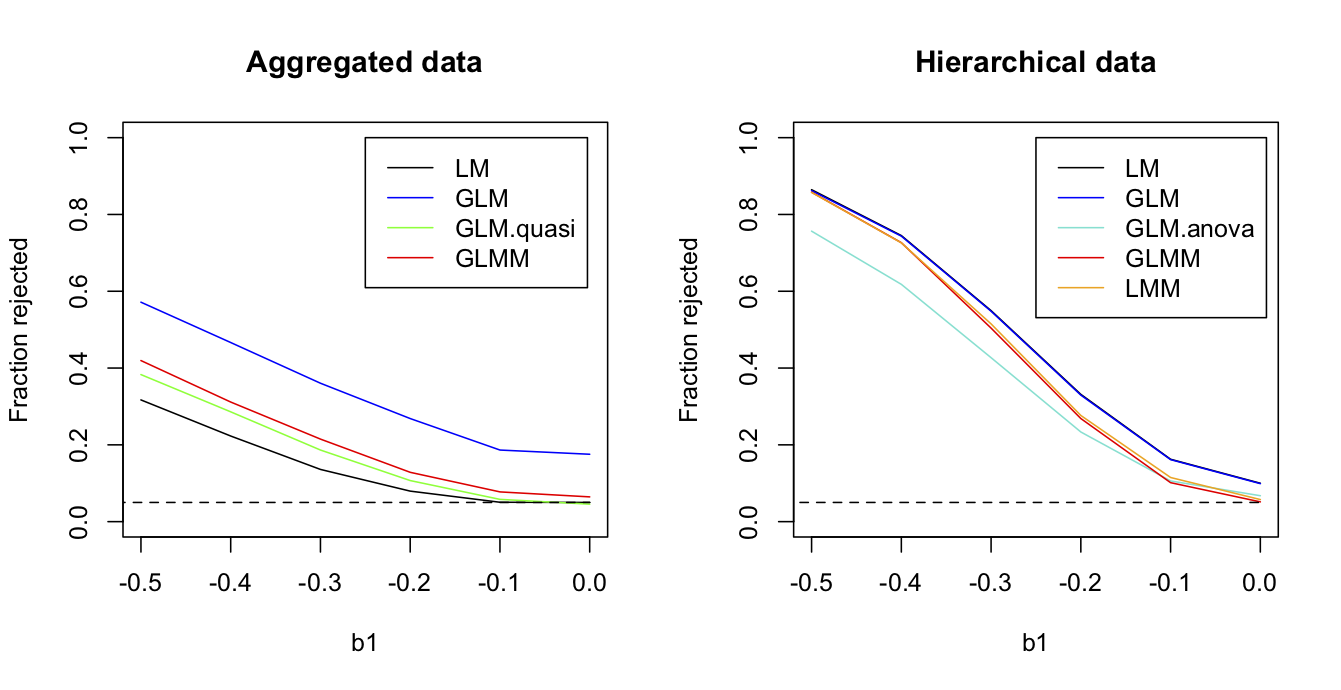Fig. 2.9: Curves showing the fraction of simulations from the station-level GLMM rejected by four methods used to analyze route-level data (left panel) and the five methods used with the station-level data (right panel). In the right panel lines for the LM (black) and GLM (blue) coincide. Parameter values used to simulate data are b0 = -1.063, b1 = -0.406, and sd.route = 1.07.

Turning to the station-level data, the LM and GLM that don’t account for the correlated structure of the data (stations in the same route having similar grouse abundances) both have inflated type I errors. This is a very common result and is a key reason for accounting for correlated residuals in statistical analyses. The three methods that account for the correlated structure of the data, the LMM and GLMM have pretty good type I error control. The GLM.anova is the GLM in which routes are treated as categorical fixed effects; although GLM.anova has acceptable type I error control, it has lower power. As described in subsection 1.5.4, this likely occurs because no grouse were observed in 22 of the 50 routes, and information from these routes would be absorbed with the route factors and therefore ignored, rather than be used for inference about the effects of WIND.

#### 2.7.3 Do hierarchical methods have more power?

Perhaps the most striking pattern in figure 2.9 is the greater power of the station-level methods in comparison to the route-level methods. Since the station-level methods use datasets with many more points (372 vs. 50), this is not surprising, right? Wrong. The reason for the increased power of the station-level methods is that they use more information, specifically the station-level information about wind speed taken during each station-level observation.

To show that the station-level methods have more power because they have more information, rather than because they have more points, I leveled the playing field by removing the station-level information about wind speed. Specifically, for the station-level analyses I kept everything exactly the same except rather than use the values of WIND measured at each station, instead I assigned the route-level value of MEAN_WIND to all stations within the same route. Thus, the number of data points (372) in the station-level data remained the same, but these points provided no more information about wind speed than the route-level mean. In this case (Fig. 2.10), the powers of the GLMM and LMM applied to the station-level data are almost identical to the powers of the quasibinomial GLM and logit normal-binomial GLMM applied to the route-level data. This is exactly as it should be. If the correlation structure of the data is correctly accounted for, and if the information available from the predictor variables is the same, then analyses of aggregated data should give the same result as analyses of the hierarchical data. If not, your analyses are wrong, since hierarchical models can’t make information from nothing. But comparison with the GLM.anova shows that they can stop you from losing information. The GLM.anova has little power, a consequences of the information lost when it fails to use information from the 22 routes in which no grouse were observed.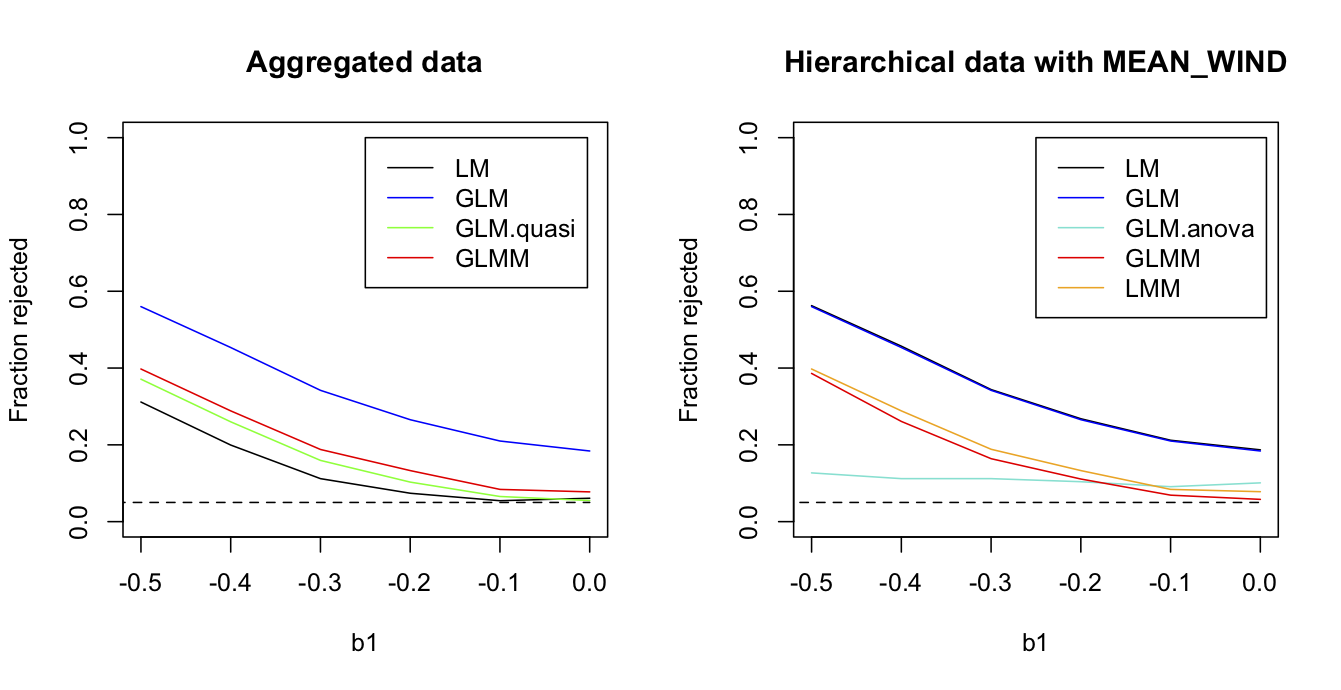Fig. 2.10: For the case in which MEAN_WIND is used for all stations within the same route, the fraction of simulations rejected by four methods used to analyze route-level data (left panel) and the five methods used with the station-level data (right panel). Parameter values used to simulation data are b0 = -1.063, b1 = -0.406, and sd.route = 1.07.

### 2.8 Summary

1. Estimators are functions of random variables that describe your data and have useful properties that lead to estimates of parameters in statistical models. A good estimator should have an expectation equal to the true value of the parameter it estimates (lack of bias). A good estimator should have high efficiency (high precision or low variance). And a good estimator should be consistent, converging to the true value of a parameter as the sample size increases.
2. Even standard statistical tests (except those from OLS) use approximations to compute P-values, and these approximations are prone to poor results when sample sizes are small. You should therefore test them. If you find a problem with a statistical test, it is often relatively easy to use parametric bootstrapping of the null hypothesis to give accurate P-values.
3. Failing to account for correlation in your data can lead to badly inflated type I errors (false positives). This was shown with the grouse data, but is a common result.
4. The problem presented by discretely distributed data, or more generally non-normal data, rests mainly in the problem of heteroscedasticity. Often the problem of heteroscedasticity is not that great, so linear models are often a robust choice when all that is wanted is a test for whether a relationship is statistically significant. To simulate discrete data, however, it is necessary to fit a GLM or GLMM.
5. Hierarchical models, such as LMMs and GLMMs, are sometimes thought to have more statistical power because they use more data. However, having more data points per se does not increase statistical power, and if you find it does, then your statistics are probably wrong. However, if a hierarchical model uses more information in the data, then it can have more power.

### 2.9 Exercises

1. As discussed in subsection 2.6.5, the LM should give good type I errors even when applied to binary data, provided the residuals are homoscedastic. This will be true for the simplest case of only a single predictor (independent) variable, because under the null hypothesis H0:b1 = 0, the residuals will be homoscedastic (since the predicted values for all points are the same). However, if there is a second predictor variable that is correlated to the first, then under the null hypothesis H0:b1 = 0 the residuals will not be homoscedastic. This could generate incorrect type I errors for the LM. In the simulations of binary data, this didn’t seem to cause problems with type I error for the LM (section 2.6). For this exercise, investigate this problem for binomial data with more than two (0 or 1) outcomes. You can modify the code from section 2.6 for this.
2. When analyzing the binary regression model, I left out one of the best methods: logistic regression using a Firth correction. I won’t go into details to explain how logistic regression with a Firth correction works, but the basic idea is to fit the regression using ML while penalizing the likelihood so that it doesn’t show (as much) bias as the binomial glm. To see how logistic regression with a Firth correction performs, use the function logistf() in the package {logistf} to produce the same power figure as in Fig. 2.8 (subsection 2.6.6). Don’t bother including the bootstraps, since they take more time. You can still compare how well logistf() does compared to the other methods, since the LM performed the same as the bootstrap of H0. For the simulations, you fit logistf() using the syntax mod.logistf <- logistf(Y ~ x1 + x2). You can then extract the P-value for x1 with mod.logistf$prob. 3. As an exercise, produce figure 2.10 showing the power curves for the grouse data assuming that the station-level values of WIND are given by MEAN_WIND for all stations within the same route. This can be done easily by modifying the code provided for making figure 2.9. ### 2.10 References Efron B. and Tibshirani R.J. 1993. An introduction to the bootstrap. Chapman and Hall, New York. Firth D. 1993. Bias reduction of maximum likelihood estimates. Biometrika 80:27-38. Ives A.R. 2015. For testing the significance of regression coefficients, go ahead and log-transform count data. Methods in Ecology and Evolution, 6:828-835. Judge G.G., Griffiths W.E., Hill R.C., Lutkepohl H, and Lee T.-C. 1985. The theory and practice of econometrics. Second edition. John Wiley and Sons, New York. Larsen R.J. and Marx M.L. 1981. An introduction to mathematical statistics and its applications. Inc., Englewood Cliffs, N. J, Prentice-Hall. Smyth G.K. and Verbyla A.P. 1996. A conditional likelihood approach to residual maximum likelihood estimation in generalized linear models. Journal of the Royal Statistical Society Series B-Methodological 58:565-572. ## Chapter 3: Phylogenetic Comparative Methods ### 3.1 Introduction Phylogenetic comparative methods involve models designed to compare traits among species. Because there is a good chance that phylogenetically closely related species are more likely to be more similar than distantly related species, a natural hypothesis is that correlations in trait values among species reflect phylogenies. Therefore, phylogenetic data present similar challenges as hierarchical data: just as observations among experimental plots within the same sites may be correlated, so might observations among closely related species. Even though phylogenetic correlations are commonly observed in data, this is not universal. Therefore, phylogenetic correlations should be treated as hypotheses, just as hierarchical correlations are treated as hypotheses when they are incorporated into a mixed model as a random effect. Phylogenetic analyses address patterns of traits among extant species, or occasionally extinct species for which remains are available. Therefore, I am going to avoid the word “evolution” as much as possible. Phylogenetic comparative methods do not directly give information about evolution, and it is easiest to explain these methods without placing them in an explicit evolutionary context. Of course, the distribution of traits among extant species reflects evolutionary history. It was these distributions that, in part, led Darwin to the idea of evolution by natural selection and common descent. Nonetheless, hypotheses tested with phylogenetic models involve extant patterns, and therefore any interpretation beyond extant patterns is beyond the statistical inference of the models. I find it safest to focus on statistical inference and keep evolutionary interpretations separate. This chapter gives an introduction to phylogenetic comparative methods, emphasizing the themes of Chapters 1 and 2: the statistical challenges of correlated data, and the properties of estimators designed for such data. I will begin with an explicit comparison between phylogenetic and hierarchical models, showing how closely they are related and, by extension, how the concepts from Chapters 1 and 2 map into this chapter. Chapters 1 and 2 primarily addressed the estimation of regression coefficients (fixed effects) of models. Here, I will address first the identification of phylogenetic correlations of trait values among species. The magnitude of these phylogenetic correlations is generally referred to as phylogenetic signal (Blomberg et al. 2003). I will then address estimation of regression coefficients. ### 3.2 Take-homes 1. Phylogenetic comparative methods involve models that explicitly include the hypothesis that phylogenetically related species are more likely to have similar trait values. Whether this hypothesis is correct is open to statistical tests. 2. Estimating the strength of phylogenetic signal involves estimating covariances in the data. Estimating covariances is sometimes harder statistically than estimating regression coefficients, and therefore requires caution. 3. All of the issues that can corrupt results from analyses of hierarchical data also apply to phylogenetic data. In particular, don’t trust the P-values that are reported from established methods. 4. As found for hierarchical data, failing to account for phylogenetic correlation can lead to badly inflated type I errors (false positives). 5. Most phylogenetic comparative analyses are performed under the assumption that the topology of the phylogeny is correct. When it is not correct, increasing topological mistakes increase the statistical problems that are found when phylogenetic signal is ignored altogether. 6. Phylogenetic models can be formulated for binary data. These models experience the same challenges as models for continuous data, with the challenges exacerbated by the lower information content of binary data. ### 3.3 Phylogenetic correlation To explicitly compare the structures of hierarchical and phylogenetic models, I’ll begin by constructing a “phylogeny” for hierarchical data. I’ll then use the equivalence of hierarchical and phylogenetic data to explain how phylogenetic signal can be incorporated into models. #### 3.3.1 Phylogenetic covariances In subsection 1.5.5 I constructed the covariance matrix for a hierarchical LMM for the grouse data, in which stations were nested within routes. The covariances between two stations in the expected number of grouse observations depend upon whether they are in the same or different routes. Therefore, assume stations i and j are in the same route, and station k is in a different route. If the random effect for route is s2_b and the residual (uncorrelated) error variance is s2_e, then the covariance matrix is s2_b*V + s2_e*I. To make this concrete, assume s2_b = 1 and s2_e = 2, which gives the covariance matrix for 4 stations nested within 3 routes: This covariance matrix can visualized as a phylogeny by letting the branch lengths connecting stations within the same route be equal to the covariance between them, and the residual error variance be equal to the length of the tips of the phylogeny (Fig. 3.1, left panel).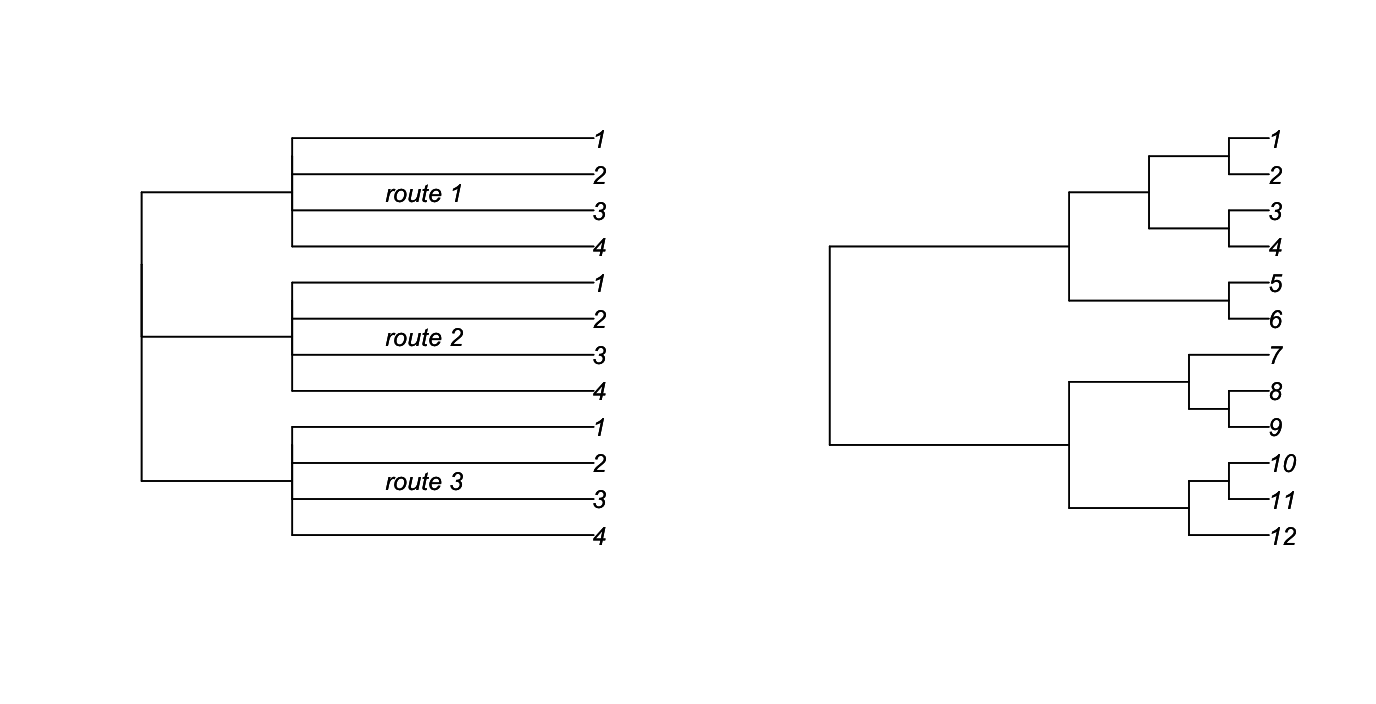Fig. 3.1: On the left is a “phylogenetic tree” for the covariance matrix in the text with four stations within each of three routes. On the right is an ultrametric phylogeny produced from the rtree() function in ape. A phylogeny can be graphed in a similar way, with the covariance between the species at the tips of the phylogeny equal to the height of the node of their most recent common ancestor. The phylogeny in the right panel of figure 3.1 corresponds to the covariance matrix The comparison between the two phylogenies and their covariance matrices illustrates the similarity between hierarchical and phylogenetic data. They both have structured covariance matrices. In the case of hierarchical models, the matrices are often block diagonal, as they correspond to nested structures in the data. This is not necessary, however, and the logit normal-binomial model from Chapter 1 (subsection 1.4.4) is an example of a mixed model that does not have a block-diagonal covariance matrix. For phylogenetic models, the structure of the phylogeny forces the covariance matrix to be clustered, so that, for example, the covariance between species 1 and 3 is equal to the covariance between species 1 and 4, since species 1 had the same shared ancestor with species 3 and 4 (Fig. 3.1). I’ve shown an ultrametric phylogenetic, in which all tips are contemporaneous. It is also possible to have all tips ending at different distances from the base of the tree; in fact, this is the most common situation for phylogenies derived from molecular genetic data. #### 3.3.2 The strength of phylogenetic signal Because the strength of phylogenetic signal – the magnitude of correlations among related species – varies among traits and groups of species, a statistical model needs to allow the strength of phylogenetic signal to vary. For the simple hierarchical data in figure 3.1 (left panel), the magnitude of correlations depends on the magnitude of the random effect variance relative to the residual variance; the larger the random-effect variance, the greater the hierarchical correlation. To allow a similar gradation for the strength of phylogenetic signal, branch-length transforms are used to push the locations of the internal nodes of the phylogeny either towards the tips (increasing phylogenetic signal) or towards the base (decreasing phylogenetic signal). The two most common branch-length transforms are Pagel’s λ (Pagel 1997, 1999) and the Ornstein Uhlenbeck (OU) transform (Martins and Hansen 1997). Pagel’s λ transform involves reducing the height of the phylogeny by a factor λ and adding length to the tip branches by a factor (1 - λ), as shown in figure 3.2. This branch-length transform is similar to the structure of mixed models in which the random effect variance is reduced relative to the error variance, which extends the relative length of the tip branches.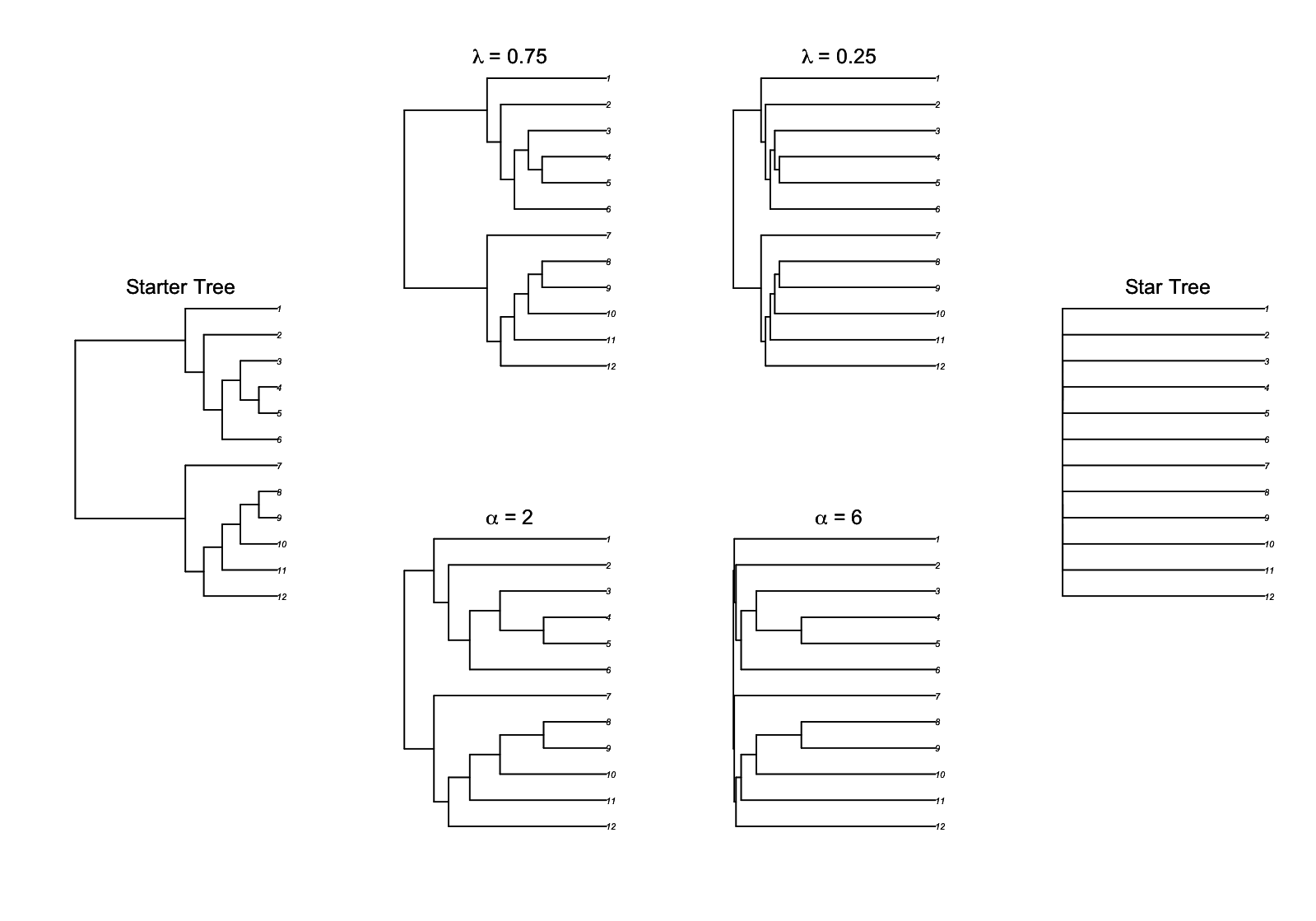Fig. 3.2: Pagel’s λ and the OU branch-length transforms with the “starter tree” at the left (λ = 1 and α = 0), the “star tree” at the right (λ = 0 and α = ∞), and intermediate values of λ and α in between. The second common branch-length transform, the OU transform, has different variants in the literature. The one I prefer is derived so that when its parameter d is zero (no signal) a “star” phylogeny is given in which all branches radiate from the base and there is no correlation between tips; when d is one, the original “starter” phylogeny is given. This formulation of the OU transform was what we used in Blomberg et al. (2003). However, the more common version in R packages has a parameter α that is inversely related to the strength of phylogenetic signal; when α = 0, there is strong phylogenetic signal, while as α increases to infinity a star phylogeny is given in which there is no covariance among species. As another complication, the original OU transform in Martins and Hansen (1997) and implemented in the package ape assumes that the trait value at the root of the phylogeny is not fixed, so that there are no zero elements in the covariance matrix, and the original starter tree is not recovered when α = 0. Here I use the OU transform implemented in phylolm() that does recover the starter phylogeny when α = 0. Figure 3.2 shows this transform paralleling Pagel’s λ transform; although they both change the strength of phylogenetic signal, they do this somewhat differently, with the OU transform tending to shorten branch lengths towards the base of the phylogeny more than Pagel’s λ transform. #### 3.3.3 Brownian motion evolution Translating a phylogeny into a covariance matrix is generally described in the literature in terms of a “model of evolution”, so I will break my silence on evolution briefly in this subsection. Earlier I asserted that a phylogeny was a visual depiction of a covariance matrix. It is, but there is more to this association than visual. Phylogenies, as depicted by trees, give information about genetic diversification if they are generated from molecular data; morphological diversification if they are generated from trait data; or time if they are calibrated with the fossil record. There is a mathematical way to take a phylogeny and generate a covariance matrix. Specifically, if a hypothetical trait evolves in a Brownian motion fashion, increasing or decreasing a very small amount every very small time step up the phylogenetic tree, then the trait value will follow a stochastic random walk that branches at each node on the tree. Such as random walk has the property that the variance increases linearly with time. This means that the branch lengths on the phylogenetic tree are proportional to the variances and covariances of trait values among species. Thus, “Brownian motion evolution” gives a direct translation from phylogeny to covariance matrix. The OU branch-length transform also is derived from a model of evolution. In the OU case, the model involves stabilizing selection to a global optimum. This stabilizing selection tends to erase the history of trait values; as time progresses, the stabilization exerts more influence as it bounds trait values around the optimum, so the values “forget” their starting points. Stated another way, the variance in trait values decelerates through time, so covariances in the distant past are reduced. This decrease of phylogenetic covariances can be modeled on the starter tree as OU evolution, or the starter tree can have its branch lengths transformed according to an OU process and then the phylogenetic covariances modeled as a Brownian motion process. Throughout this chapter, for clarity I’ll use the latter approach of first performing a branch-length transform and then pulling variances and covariances from the resulting covariance matrix under the assumption of a Brownian motion process. ### 3.4 Estimating phylogenetic signal Phylogenetic signal can be estimated from a dataset and accompanying phylogeny as the value of the branch-length transform parameter (e.g., λ or α). This can be done with or without predictor variables in the model. I’ll first present the general regression model and then discuss the estimation of phylogenetic signal parameters, first for the case in which there are no predictor variables. When there are no predictor variables, the model gives a direct estimate of phylogenetic signal. #### 3.4.1 Phylogenetic regression model A phylogenetic regression model for a single predictor variable is Y = b0 + b1*x + e e ~ norm(0, s2*V(θ)) Here, the covariance matrix of the errors, V(θ), contains the phylogenetic covariances and a phylogenetic signal parameter θ that could be λ or α. In contrast to a mixed model which would have two covariance matrices, one for the random effect and a second for the errors, the phylogenetic regression model might only have a single covariance matrix. However, for Pagel’s λ transform, the covariance matrix V(λ) can be written as the sum of two matrices, λ*V + (1 - λ)*I where V is the starter covariance matrix (typically the one derived assuming Brownian motion evolution) and I is the identity matrix. This phylogenetic regression model is often referred to as a Phylogenetic Generalized Least-Squares model (PGLS). Technically, because the covariance matrix contains a parameter θ that must be estimated, this is really an Estimated GLS model (EGLS). Because a well-used package for fitting EGLSs is nlme, in which the function gls() solves the EGLS problem, I’m not going to fight the usage of PGLS. The important point, though, is that simple GLS can’t be used for estimating parameter values. Instead, ML or REML are generally used, although there are alternatives (Ives et al. 2007). For this specific model, ML or REML will give estimates of the regression coefficients (b0 and b1), the phylogenetic signal (θ), and s2 that scales the residual variance. Of course, this regression model can be expanded to include multiple predictor variables, and interactions among them. Unlike hierarchical models, however, there is typically only a single parameter that is estimated in the covariance matrix. Exceptions to this are models that attempt to identify regions of the phylogeny in which changes in trait evolution are relatively fast or slow, or where there has been a shift in the optimum trait value governing an OU process (Butler and King 2004, Bartoszek et al. 2012). I won’t talk about these models in this book, because they involve both statistical and conceptual challenges; I would only attempt this type of analyses with very large numbers (many hundreds) of species and a very clear a priori hypothesis for how the rate of trait evolution might vary. For the case without a predictor variable x, figure 3.3 gives a simulated example, with branches colored according to a hypothetical trait evolving in a Brownian motion fashion up a phylogeny. The tip trait values are plotted in the panel on the right. The clustering of points reflecting phylogenetic correlations is clear.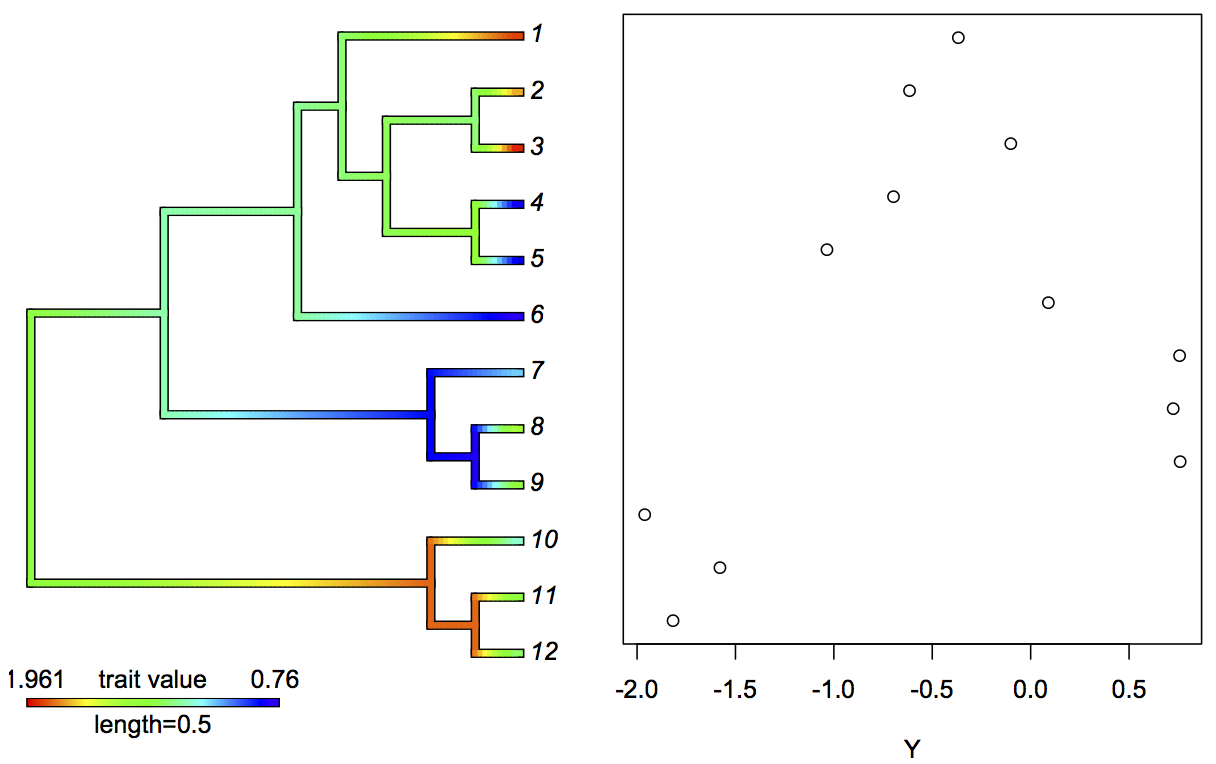Fig. 3.3: Brownian motion evolution of a hypothetical trait up a phylogeny, with colors on the branches corresponding to the trait values using the phytools package (Revell 2012). The right panel gives the trait values at the tips of the phylogeny. #### 3.4.2 Analyzing hierarchical data as a phylogeny To hammer home the close relationship between hierarchical and phylogenetic models, I fit simulated hierarchical data using both a LMM and a phylogenetic model. The hierarchical data and LMM have the same structure as the hierarchical data in figure 3.1, with stations nested within routes. The phylogenetic model requires constructing a “phylogeny” for the hierarchical data, which involves taking the covariance matrix suitable for stations nested within routes and converting it into a phylogenetic object of class phylo in the package ape (Paradis et al. 2004). For this illustration, I simulated data for 4 routes each containing 6 stations (Fig. 3.4).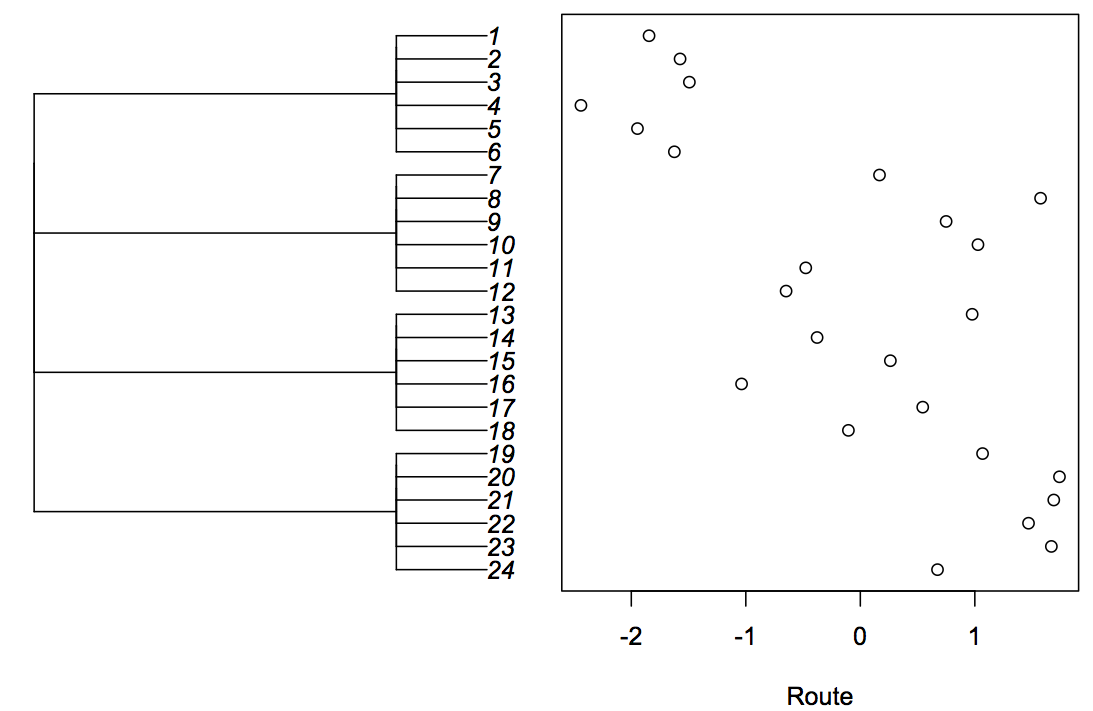Fig. 3.4: “Phylogenetic tree” for the covariance structure of 4 routes each containing 6 stations used for fitting hierarchical data as a PGLS. Data in the panel on the right were simulated from the covariance structure on the left. I fit the phylogenetic model using Pagel’s λ branch-length transformation, since this takes the form of the sum of a phylogenetic covariance matrix and the identity matrix, which has the same form as the covariance matrix for the LMM with a random effect for route (although parameterized differently, since the phylogenetic covariance matrices are multiplied by λ and (1-λ), while the LMM matrices are multiplied by 1 and s2_b). The results from the LMM and PGLS are The logLik of both models is the same, indicating that they fit the data the same. Showing that the covariance for stations within routes are the same involves extracting the covariance from the phylogenetic covariance matrix, vcv. This is given by λ (= z.pgls$optpar) times the covariance in vcv (I just picked the element in the second row, first column, vcv[2,1]) and the estimate of the overall variance s2 (= z.pglssigma2). That the covariances for stations within routes are the same for the LMM and PGLS demonstrates that they are the same model. #### 3.4.3 Choosing a branch-length transform Given the several possible branch-length transforms that could be used to assess phylogenetic signal, how do you select the best? An appropriate way is to use the likelihood of the fitted model. As discussed in subsection 2.3.2, the likelihood gives the probability of observing the data conditional on the values used for the parameters in a model. When these parameters are fit by ML, the likelihood of the resulting model is the highest possible for the given branch-length transform. Therefore, if one branch-length transform allows the model to fit the data better than another branch-length transform, then its likelihood will be higher. To illustrate this, I first simulated a phylogeny using the rtree() function in ape, additionally using the function compute.brlen() with a Grafen branch-length transformation to make it ultrametric. I used the transf.branch.lengths() function from phylolm() (Ho and Ane 2014) to transform the branch lengths according to Pagel’s λ, and simulated data using rTraitCont() in ape. Finally, I fit the simulated data using phylolm() using either Pagel’s λ (model = "lambda") or the OU branch-length transform (model = "OUfixedRoot"). Below, I’ve abbreviated the output to show the important results. phylolm() gives the fitted measure of phylogenetic signal (lambda or alpha) that transforms the covariance matrix, and sigma2 that scales the covariance matrix. Because the branch-length transforms change the variances in the covariance matrices, the estimates of sigma2 can’t be compared. However, the log likelihood logLik is clearly higher for the model fit with Pagel’s λ, indicating that this is the better of the two branch-length transforms. Repeating these simulations 100 times shows that the branch-length transform used to simulate the data isn’t always the one that gives the better fit to the simulated data. Even for n = 100 species, 7% of the datasets simulated with λ = 0.5 were fit better using an OU branch-length transform, and when the data were simulated with an OU branch-length transform with α = 1, 16% of the simulated datasets were fit better using Pagel’s λ. This is a indication that it is often difficult to fit details of the covariance matrix: covariances can be hard to estimate. ### 3.5. Statistical tests for phylogenetic signal There are multiple ways of testing for the presence of phylogenetic signal in a dataset. Somewhat surprisingly, most ways that are available in common packages in R do not have great statistical properties: most have low statistical power. Here I present methods that I discussed in chapter 2: the Likelihood Ratio Test (LRT), a parametric bootstrap of the phylogenetic signal parameter, and a parametric bootstrap of the null hypothesis H0 that there is no phylogenetic signal. I’ll also introduce a permutation test and Blomberg’s K. For all of these examples, I’ll analyze the same dataset that I generated by simulation to have moderately strong phylogenetic signal (λ = 0.5) using the code #### 3.5.1 Likelihood Ratio Test I introduced the Likelihood Ratio Test (LRT) in subsection 2.6.2 as a general method to test the significance of a component of a model by comparing the full model containing that component to the reduced model without the component. In subsection 2.6.2, the parameter in question was a regression coefficient, but LRTs can also be used to give P-values in statistical tests of phylogenetic signal that involve the covariances of the errors. For this, the LRT compares a full model in which phylogenetic signal is estimated using ML to the reduced model in which there is no phylogenetic signal; the appropriate reduced model here is just a LM. Twice the log of the likelihood ratio (LLR) between models is asymptotically (as n gets large) chi-square distributed. The LRT is a standard way to generate P-values, although we’ll find below that they are prone to deflated type I errors and loss of power for small sample sizes. For the simulated dataset used in the following examples, LRTs for Pagel’s λ and the OU transforms were P = 0.12 and P = 0.22, respectively. #### 3.5.2 Parametric bootstrap of the parameter It is possible to test for phylogenetic signal by performing a parametric bootstrap of the estimator of the phylogenetic signal parameter. This bootstrap gives the approximate distribution of the estimator from which P-values can be derived. The procedure of the parametric bootstrap is (subsection 2.6.3): 1. Fit the model to the data. 2. Using the estimated parameter value of interest, simulate a large number of datasets. 3. Refit the model to each simulated dataset. 4. The resulting distribution of the parameter values estimated from the simulated datasets approximates the distribution of the estimator, allowing P-values to be calculated. phylolm() will perform this bootstrap for you automatically when you specify a number for boot. The bootstrap of the parameter can lead to incorrect P-values when the estimator is biased (subsection 2.6.3). This turns out to be the case for the estimator of phylogenetic signal (Fig. 3.5). The estimates of λ and α are biased towards less phylogenetic signal (lower λ and higher α) than used to simulate the bootstrap datasets. This is seen in Fig. 3.5: the mean of the parameter estimates (λ and α) given by the green lines are closer to the value of no signal (λ = 0 and α = 50, the upper limit of α in phylolm) than the value used to simulate the data given by the red line. This underestimate of the phylogenetic signal is anticipated to lead to type I errors that are too low and a corresponding loss of statistical power to detect phylogenetic signal.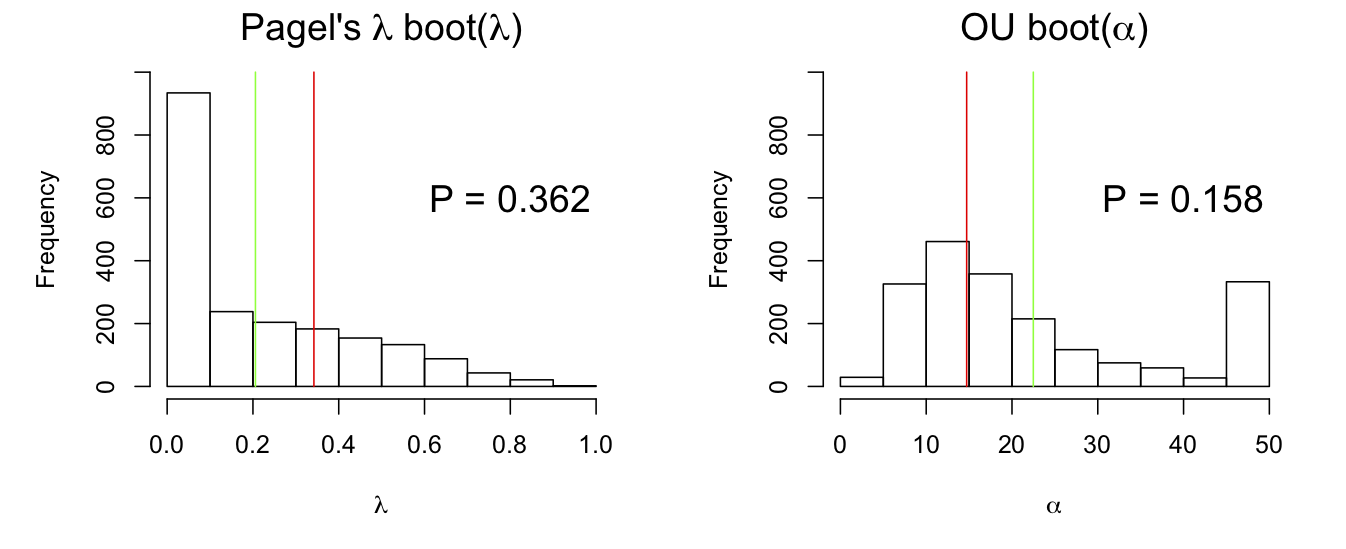Fig. 3.5: Bootstrap distributions of phylogenetic signal (λ and α) calculated from phylolm() for a simulated dataset. The red lines give the value of the phylogenetic signal parameter used to bootstrap the data, and the green lines are the means of the estimates. Note that the bootstrap estimates of λ and α tend to cluster at values giving no signal (λ = 0 and α = 50). This is typical for estimates of phylogenetic signal, and in general for ML estimates of variance components of models for correlated data. For ML estimation of variances and covariances when they are weak, there is often a peak in the likelihood function at zero, leading to estimates of zero. This type of absorption at zero (no phylogenetic signal) underlies the bias in the estimates of phylogenetic signal found for the parametric bootstrap of the estimator (Fig. 3.5). #### 3.5.3 Parametric bootstrap of H0 The parametric bootstrap of H0 involves the following procedure (subsection 2.6.4): 1. Fit the model to the data and calculate the log likelihood ratio (LLR) between the full model and the reduced model without the parameter of interest. 2. Using the estimated parameters from the reduced model, simulate a large number of datasets. 3. Refit both the full and reduced models for each dataset and calculate the LLR. 4. The resulting distribution of LLRs estimated from the simulated datasets approximates the distribution of the estimator of the LLR, allowing P-values to be calculated. This bootstrap is related to a LRT, but rather than approximate the distribution of 2*LLR by a chi-square distribution, the distribution is obtained by parametric bootstrapping. Figure 3.6 shows the bootstrap estimates of 2*LLR for simulations under H0. Under the standard LRT, these distributions should be chi-square (blue lines), which they are not. This is due to the absorption of estimates for no phylogenetic signal, which is observed in the mass of values of LLR close to zero. This does not necessarily mean that the parametric bootstrap of H0 is wrong; this absorption at zero occurs under the null hypothesis that there is no phylogenetic signal. Instead, the comparison between the parametric bootstrap of H0 shows that the chi-square approximation is poor, because it fails to account for the tendency of the estimator to absorb at zero.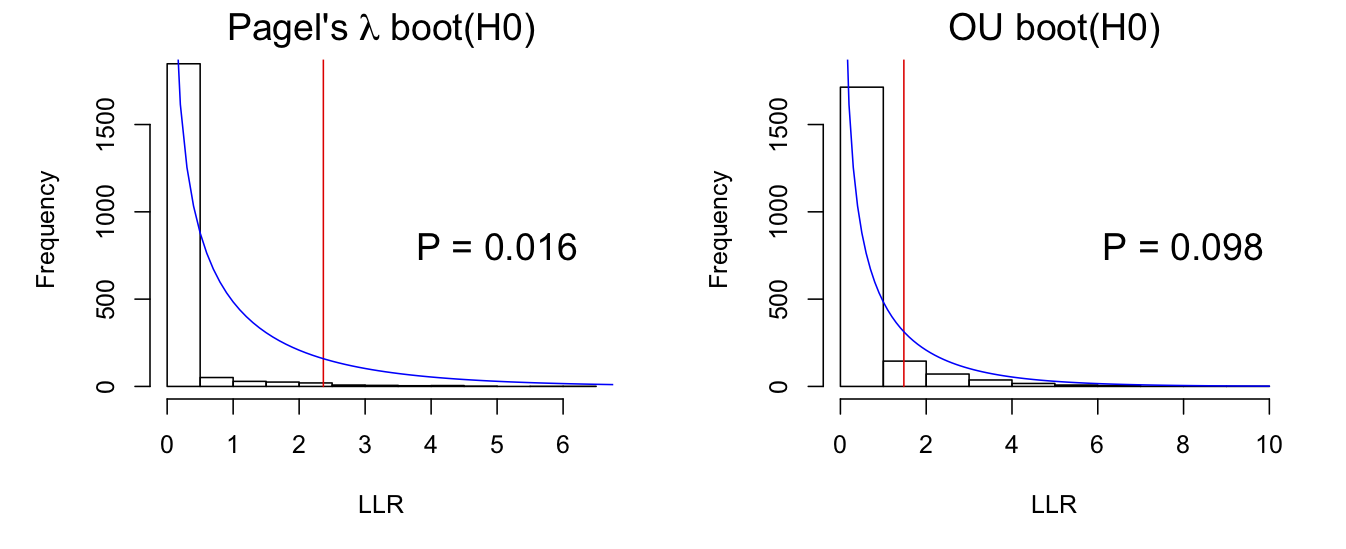Fig. 3.6: Bootstrap distributions of 2*LLR for Pagel’s λ and OU models under the null hypothesis that there is no phylogenetic signal. Simulated data were fit using phylolm(). A chi-square distribution with df = 1 is shown by the blue line. #### 3.5.4 Permutation test A permutation test can be performed that is very similar to the parametric bootstrap of H0. In general, permutation tests are the gold standard for testing null hypotheses involving correlations among data. In this case, under the null hypothesis that there is no phylogenetic correlation, trait values can be randomly permuted among species without changing the structure of the data. Thus, the permutation test involves generating a large number of permutation datasets, fitting each with a model for phylogenetic signal, and calculating P-values from the fraction of estimates that are more extreme than the estimate from the original dataset. Using a permutation test for the data we’ve been analyzing (Fig. 3.7) gives distributions of estimates of λ and α very similar to those obtained with the parametric bootstrap of H0 (Fig. 3.6), and very similar P-values.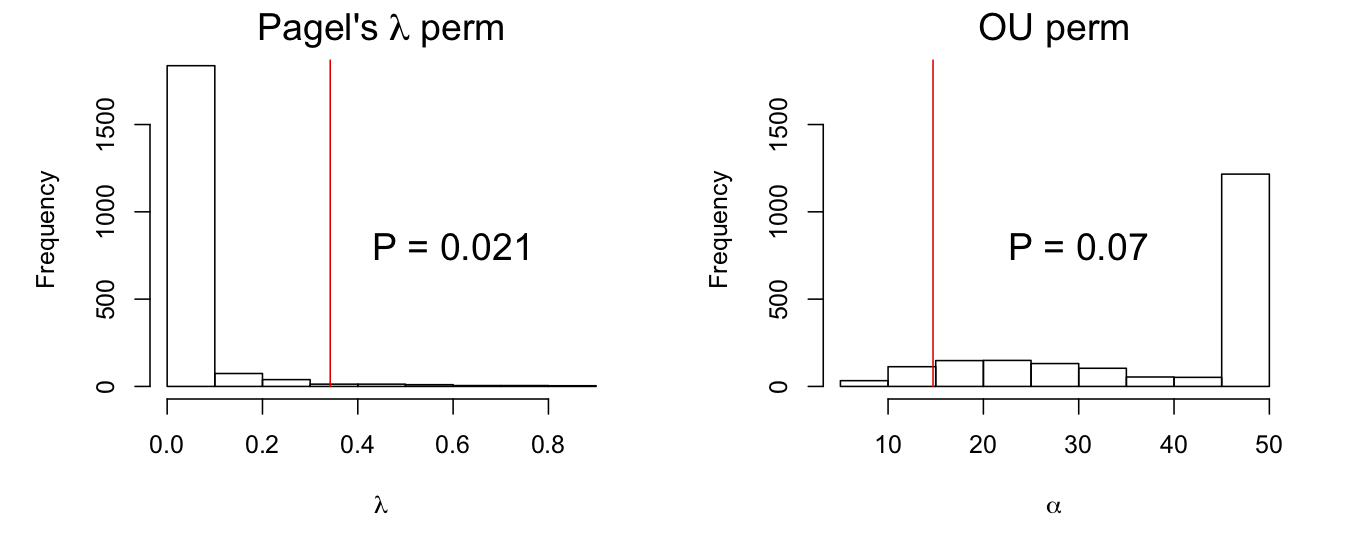Fig. 3.7: Permutation distributions of phylogenetic signal (λ and α) calculated using phylolm() by permuting values of Y among species under the null hypotheses that there is no phylogenetic signal. The red lines give the “true” value of the phylogenetic signal parameters estimated from the data. I need to give a short digression about the conceptual differences between bootstraps and permutation tests. First, the bootstrap tests I’ve been using so far have all been parametric bootstraps, in which a model is fit and datasets simulated from the fitted model. “Parametric” refers to the fact that a specific distribution has to be used to simulate the errors. The standard bootstrap is the non-parametric bootstrap (or simply “bootstrap” without the “non-parametric”). In the non-parametric bootstrap, there is no assumption about the distribution of errors. Instead, the actual residuals from the fitted model are used as the errors to generate the bootstrap datasets. For the tests of phylogenetic signal, this would involve fitting the model (the very simple model with only an intercept), collecting the residuals (differences from the mean), and for each species selecting from these residuals with replacement to construct the bootstrap datasets. The only difference between this procedure and the permutation is that the bootstrap resamples the residuals with replacement, whereas the permutation resamples residuals without replacement (since permuting residuals around the mean is the same as permuting the values). Although the only difference between non-parametric bootstrap tests and permutation tests is that the former resamples with replacement and the latter resamples without replacement, the interpretation of these tests is different (Efron and Tibshirani 1993). The non-parametric bootstrap, as with the parametric bootstrap, gives an approximation of the distribution of the statistical estimator for the parameter in question under H0. The heuristic justification for this is that the residuals from a fitted model give n realizations of the true process underlying the experiment, and therefore they can be resampled as if they were the complete distribution in order to derive the distribution of the estimator. The permutation test does not involve any thought of the estimator or statistical inference about the process underlying the data. Instead, the permutation test asks what the probability is of observing the pattern in the data under the assumption it is random (as given by the permutation). In short, bootstrapping gives an approximation of the estimator while a permutation test gives a probability of an outcome. I have focused on bootstraps in this book rather than permutation tests, because a major goal is to explain statistical inference, which involves understanding the processes that generate data. Bootstraps directly involve inference about statistical processes. Furthermore, I’ve used parametric bootstraps rather than non-parametric bootstraps. A non-parametric bootstrap could easily have been performed for testing for phylogenetic signal. A limitation of non-parametric bootstrapping often arises in hierarchical data, however, because it is impossible to derive a non-parametric resampling scheme for the residuals to test a null hypothesis of interest. For example, suppose you wanted to test the significance of a regression coefficient in a GLMM, such as testing for the effect of the variable WIND on station-level observations of grouse (subsection 1.5.4). Because the residual errors are not independent (observations from stations within the same route are correlated), the residuals can’t be resampled independently to construct the bootstrap datasets. An added problem in this example is that the observations are binary (grouse present or absent), so the residuals can’t be resampled in a way to retain binary values of the observations. Thus, parametric bootstrapping is possible even when non-parametric bootstrapping is impossible, either due to correlations in the residual errors that need to be preserved for the statistical test, or due to the discreteness of the data. #### 3.5.5 Blomberg’s K Blomberg’s K is a metric of phylogenetic signal. This is distinct from a model, such as a PGLS with a Pagel’s λ or an OU branch-length transform that gives a parameter (λ or α) corresponding to phylogenetic signal. The PGLS requires a model based on a stochastic process that is thought to underlie the data; the model provides enough information to simulate the data. In contrast, metrics are values computed from the data without making assumptions about the underlying stochastic process generating the data. Calculating a metric like Blomberg’s K does not lead to a model that can be used to simulate the data. We designed Blomberg’s K around the mean-squared-error (MSE) of the data (Blomberg et al. 2003). If there is no phylogenetic correlation among the residuals R, then the variance of the residuals is measured by the OLS MSE, which is computed by taking the sum of the squared residuals and dividing by (n - 1). If there is phylogenetic signal, then the variance of the residuals is measured by the Generalized Least squares (GLS) MSE that depends on the covariance matrix V derived from the phylogeny: MSE = (1/(n - 1))*(R’ %*% iV %*% R) where R is the n x 1 vector of residuals, R’ is the transpose of R, iV is the inverse of the matrix V, and %*% is matrix multiplication. Thus, the squared residuals are weighted by the elements of iV to account for the correlation in the data. (A more-detailed explanation is presented in Blomberg et al. 2003.) To distinguish two MSEs, let MSE(star) be the OLS MSE that assumes no phylogenetic signal (the phylogeny is a “star” with equal branch lengths radiating from the base), and let MSE(phy) be the GLS MSE calculated using the phylogeny to compute V. Blomberg’s K is based on the ratio MSE(star)/MSE(phy). If there is correlation in the residual errors, then MSE(star) decreases relative to MSE(phy), decreasing the ratio MSE(star)/MSE(phy). The ratio also depends on the number of species and the topology of the phylogeny. Therefore, we standardized MSE(star)/MSE(phy) by its expected value if the phylogenetic correlations were in fact given by V. Thus, if phylogenetic signal is the same as the phylogenetic covariance matrix V, then K = 1. Values smaller than 1 imply weaker phylogenetic signal, and values greater than 1 imply stronger phylogenetic signal. The only value of K that has a clear interpretation is K = 1. Even with the standardization relative to the expectation if the residuals had covariance matrix V, the value of K when K ≠ 1 still depends on the specific phylogenetic tree; therefore, it is not particularly useful to compare values of K among datasets from different phylogenies. Nonetheless, Blomberg’s K is useful as a metric that doesn’t make parametric assumptions about the distribution of the residual errors: the MSEs used in K are from OLS and GLS which do not assume that the errors are normally distributed. While it is true that, if traits evolved by Brownian motion up a phylogenetic tree, then the distribution of traits at the tips would be a multivariate normal distribution with covariance matrix V, this is not a prerequisite for K to measure phylogenetic signal. The null hypothesis that there is no phylogenetic signal can be tested using Blomberg’s K in a permutation test. Trait values are permuted among tips, and the resulting distribution of K is compared to the value of K calculated from the data. This permutation test is performed by the function phylosig() in the package phytools, and for the dataset we are analyzing, P = 0.018. Even though this is the same permutation test used to test for phylogenetic signal with Pagel’s λ and the OU branch-length transforms (subsection 3.5.4), the results need not be the same. Blomberg’s K uses different characteristics of the data than Pagel’s λ and OU transforms, just as Pagel’s λ and OU themselves make different assumptions about the exact form of the covariances (subsection 3.4.3). Therefore, all three might give somewhat different P-values. #### 3.5.6 Type I errors and power for tests of phylogenetic signal To assess type I errors and statistical power of different methods for identifying phylogenetic signal, I simulated data with increasing phylogenetic signal using either Pagel’s λ or OU branch-length transform (Fig. 3.8). I then tested for phylogenetic signal using Pagel’s λ and OU transforms with a LRT, Pagel’s λ and OU transforms using a bootstrap test of H0, and Blomberg’s K using a permutation test. I didn’t test for phylogenetic signal using the bootstrap of parameter estimates, because we know from subsection 3.5.2 that the estimates of λ and α are biased towards low estimates of phylogenetic signal, and therefore they will likely have low power. In these simulations, I used the same starter phylogeny because this made it possible to calculate a single bootstrap approximation of the distribution of the estimators of λ and α under H0. If instead a different phylogeny was used for each simulation, then a separate bootstrap distribution of LLR would have to be computed for each simulated dataset, because the null distributions of LLR for the Pagel’s λ and OU models could depend on the phylogeny. The overall conclusions, however, are likely to be the same if I had used a randomly constructed phylogeny for each simulated dataset.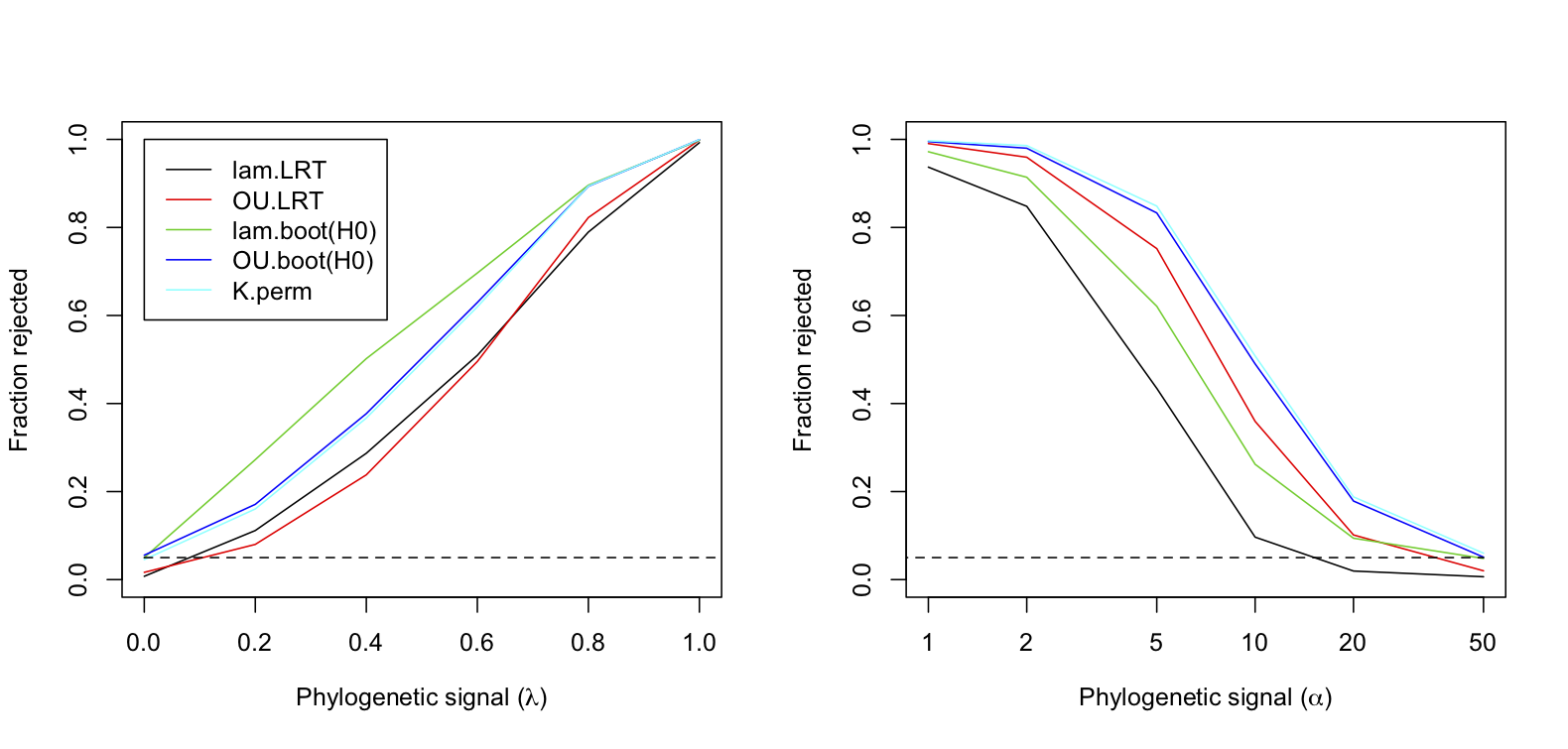Fig. 3.8: Power curves for tests of phylogenetic signal in datasets generated using Pagel’s λ branch-length transform (left panel) and the OU branch-length transform (right panel). For each set of simulated datasets, phylogenetic signal was measured using Pagel’s λ and OU transforms with a LRT (lambda.LRT and OU.LRT), Pagel’s λ and OU transforms with a parametric bootstrapped LRT under H0 (lam.boot(H0) and OU.boot(H0)), and Blomberg’s K with a permutation test (K.perm). Note that the lines for OU.boot(H0) and K.perm almost coincide. Several conclusions are apparent in figure 3.8. First, when data are simulated using Pagel’s λ branch-length transform (left panel), then the bootstrap LRT using this transform has the greatest power. Similarly, when data are simulated with an OU branch-length transform (right panel), then the bootstrap LRT using this transform has greater power than the bootstrap LRT using Pagel’s λ. This shows that the two branch-length transforms are generating different covariance patterns in the data, and the statistical test that matches the actual covariance pattern has the greater power. Second, the results for the permutation test with Blomberg’s K are almost identical to the results for the bootstrap test using the OU transform. Therefore, Blomberg’s K and the OU transform are picking up similar patterns in the phylogenetic covariances. Third, the standard LRTs that use a chi-square to approximate the distribution of LLRs generally had low power. The only case in which the standard LRT outperformed a bootstrap LRT was when data were simulated with the OU transform; in this case, the standard LRT using the OU transform outperformed the bootstrap LRT using Pagel’s λ transform (right panel). These patterns of statistical power underscore that “phylogenetic signal” is not a single, simple quantity. Phylogenetic signal refers to covariances in trait values among species, but the relative magnitude of these covariances can take different forms. Different methods will differ in their power to detect these different forms of phylogenetic signal. ### 3.6 Estimating regression coefficients The preceding analyses target phylogenetic signal which is part of the variance component of the phylogenetic models. The regression coefficients are part of the mean component of the models. To investigate estimation of regression coefficients in phylogenetic models, I simulated data using the regression model introduced in subsection 3.4.1. This regression model with one predictor variable can be simulated and fit as follows: Here, separate phylogenies allow different strengths of phylogenetic signal for the predictor variables x and the residual error e. Specifically, phy.x and phy.e are derived using transf.branch.lengths(). rTraitCont() is used to simulate both x and e, which lead to simulated values of Y. The line x <- x[match(names(e), names(x))] is used to make sure the species order in x is the same as the order in e, with the function match() aligning the index of x so that the names of e and x are in the same order. Y needs to be an array, and it has to have rownames giving the species names in the phylogeny. In general for phylogenetic analyses in R, you have to be careful to make sure that the data points match the species they correspond to. Different functions handle this differently, but phylolm() will tell you if it hasn’t found rownames in the data.frame to match the species names in the tip.label vector of the phylogeny. The simulated data are fit both to a phylogenetic model using Pagel’s λ transformation, and to a LM that does not account for phylogenetic signal. I suggest you spend a little time running the simulations and fitting the data to see how different strengths of phylogenetic signal in x and e (lam.x and lam.e) affect the results. #### 3.6.1 Where phylogenetic signal comes from The models that we were using to estimate phylogenetic signal in Y (section 3.5) were regression models with only an intercept. In these models, the source of phylogenetic signal in the residual errors was the phylogenetic relatedness of species. For the phylogenetic regression with predictor variables, the source of phylogenetic signal in the residual errors is not as obvious. This phylogenetic signal is in the variance in Y that is not explained by the predictor variable x. There are ways in which phylogenetic signal can be generated in a process involving only Y and x: if evolution of x through time represents a moving optimum for the evolution of Y, then an evolutionary lag of Y behind x can generate a phylogenetic signal in the residual error (Hansen and Orzack 2005). To me this seems like a pretty specialized situation that is unlikely to explain the ubiquity of phylogenetic signal in regression models; phylogenetic signal in the residual variation in regression models is found across a wide range of data. A simpler and (I guess) more likely explanation is that the regression model excludes other predictor variables that themselves show phylogenetic signal. Since they are not included in the model, their effects appear in the residual variation as phylogenetic signal. Thus, the regression model could be written Y = b0 + b1*x + u1 + u2 + e u1 ~ norm(0, s2_u1*V(θ_u1)) u2 ~ norm(0, s2_u2*V(θ_u2)) e ~ norm(0, s2_e*I) Here, there is residual variation in e that has no phylogenetic signal, and two unmeasured traits u1 and u2 that have phylogenetic signal. Since they are not measured, the apparent residual variation is u1 + u2 + e, which has phylogenetic signal. Of course, there might be only one unmeasured trait u1, or there might be many more than two. You might think that if there are many unmeasured traits, then their phylogenetic signals will cancel each other out: for example, two related species might have high values of u1 and low values of u2, which would degrade the overall phylogenetic signal from u1 + u2. This turns out not to be the case. If u1 and u2 are independent of each other, then the variance in u1 + u2 for species i is var[u1(i) + u2(i)] = var[u1(i)] + var[u2(i)]. The covariance in u1 + u2 between species i and j is cov[u1(i) + u2(i), u1(j) + u2(j)] = cov[u1(i), u1(j)] + cov[u2(i), u2(j)]. Therefore, if u1 and u2 have the same covariance matrix, the covariance matrix for u1 + u2 is just two times the covariance matrix for either one of them. This means that having multiple unmeasured traits doesn’t degrade the phylogenetic signal in the unexplained variation. The algebra gets a little more involved if u1 and u2 have different covariance matrices, or if u1 and u2 are themselves correlated, but these cases don’t overturn the conclusion that phylogenetic signal isn’t lost – it is just a weighted average (weighted by the variance scalars s2_u1 and s2_u2) of the phylogenetic signals of u1 and u2. #### 3.6.2 Type I errors and power Not surprisingly, type I errors and power in detecting statistically significant regression coefficients are affected by phylogenetic correlations in the errors. What is maybe more surprising is that type I error and power are strongly affected by phylogenetic signal in x; this is shown nicely by Revell (2010). The reason this might be surprising is that, in standard regression, the distribution of the predictor variables is not important: in OLS, there is no assumption about how the predictor variables are distributed, and other than making sure there are no outlying points with high leverage, the distribution of x can be ignored. For phylogenetic regression, however, the distribution of x comes into play in two ways. First, it can affect the statistical power to detect significant effects of a predictor variable. This is not a problem per se, since it is just an indication that the predictor variable contains less information if it has phylogenetic signal. Second, phylogenetic signal in a predictor variable magnifies the problems of mis-specifying the phylogenetic signal in the errors, leading to inflated type I errors. This is strictly speaking not a problem, since you should hope that you have correctly specified the phylogenetic signal of the errors. But this is a potential problem to be aware of. To illustrate these two effects of phylogenetic signal in x, I simulated datasets for different values of b1 without and with phylogenetic signal in x. I then tested the rejection rates of the null hypothesis H0:b1 = 0 for a PGLS using a Wald test, a LM using a t-test, and a PGLS using a bootstrapped LRT under the null hypothesis. For the case of no phylogenetic signal in x, all three methods had good type I error control. However, the LM had much lower power. For the case of phylogenetic signal in x, however, the LM had horrible type I error control. Even the PGLS using a Wald test had inflated type I errors, with the rejection rate of 0.064 when b1 = 0. Of course, the bootstrap LRT under H0 corrected this.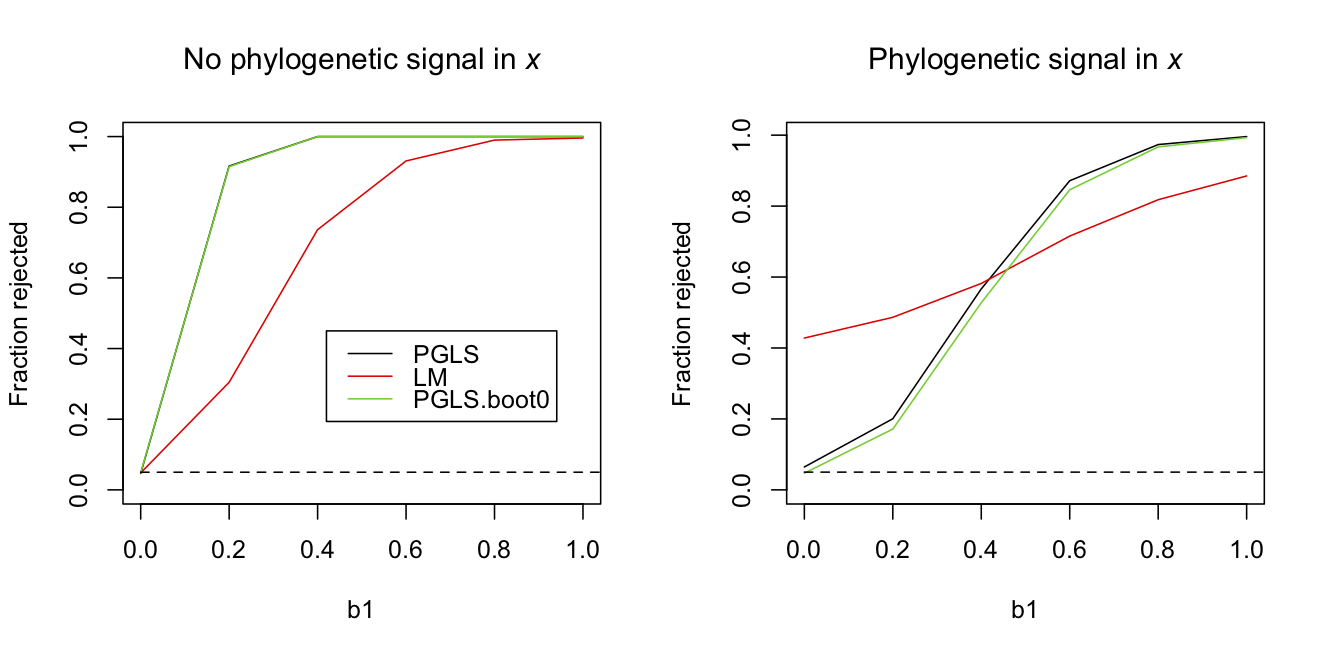Fig. 3.9: Power curves for tests of the coefficient b1 in a phylogenetic regression. Datasets of n = 30 species were simulated up a phylogenetic tree under Brownian motion evolution without (left panel) or with (right panel) phylogenetic signal in x given by Brownian motion evolution. For each level of b1 = 0, 0.2, 0.4, 0.6, 0.8 and 1.0, 2000 simulations were performed and the rejection rates calculated for a PGLS using a Wald test, a LM, and a PGLS using a bootstrapped LRT under H0:b1 = 0. Note that in the left panel the black and green lines are overlapping. The other parameters are b0 = 0, s2.e = 1 and s2.x = 1 It is disturbing that the PGLS didn’t have perfect type I error control. This is a pretty standard analysis in the evolutionary and statistical literatures. The simulations were performed all with the same phylogeny, since this makes it possible to use the same bootstrap thresholds on the likelihood ratio (otherwise, the bootstrap would be too computationally demanding). Therefore, this could be a problem that is worse for this particular phylogeny than you might expect for other phylogenies. However, using 10,000 simulations with random phylogenies selected for each, the rejection rate was 0.068, indicating that the inflated type I error rates are systemic. The best way to address this is to perform the bootstrap LRT under H0. Given that this is a routine analysis and I doubt many practitioners will use the bootstrap (even though they should), at least be skeptical of reported P-values for small sample sizes (although here “small” is n = 30). #### 3.6.3 Partial R2s A final lesson from figure 3.9 is that phylogenetic signal in x decreases the power to test the null hypothesis that b1 equals zero; this is seen in comparing the results for the bootstrap PGLS between left and right panels. Explaining the cause of this decrease in power is a reason to introduce partial R2s applied to phylogenetic data. A partial R2 measures how much explanatory power is lost when a component of a model is removed. The total R2 is the partial R2 when everything in a model is removed except for the intercept (mean). In OLS, the explanatory power of a model is measured by the proportion of the variance in Y that is explained by the model; it is just 1 - var[residuals]/var[Y]. For models with correlated data, there is no simple way of defining the proportion of the variance in Y explained by a model. Nonetheless, there are reasonable ways of defining R2 for mixed and phylogenetic models; I describe three in Ives (in press). Here, I will discuss the one that is based on the likelihoods, specifically where mod.f is the full model and mod.r is the reduced model with the component of interest removed. Thus, for the partial R2 for x depends on the difference between the full model mod.f and the reduced model mod.x: I’ve used the notation of mod.x to denote the model without x, since this is the reduce model used to test the significance of x. The R2 for the phylogenetic signal in residuals can be computed from To illustrate the partial R2s, I simulated two datasets similar to those in figure 3.9 with b0 = 0, b1 = 0.5, and phylogenetic signal in e given by lam.e = 0.8. Also, I increased n to 100 to reduce the variation from one simulation to the next and make the following table more representative of all simulations. The partial R2s for datasets simulated with and without phylogenetic signal in x were  No signal in x Signal in x full model reduced model partial R2 partial R2 1 Y ~ 1 + e(phy) Y ~ 1 + e 0.28 0.52 2 Y ~ x + e(phy) Y ~ 1 + e(phy) 0.49 0.10 3 Y ~ x + e(phy) Y ~ 1 + e 0.63 0.56 4 Y ~ x + e(phy) Y ~ x + e 0.53 0.49 5 Y ~ x + e Y ~ 1 + e 0.21 0.14 When there is no phylogenetic signal in x, the partial R2s for x (row 2) and the phylogenetic signal in e (row 4) are similar in magnitude. Furthermore, the total R2 for the model without x (row 1) and for the model without phylogenetic signal in e (row 5) are of similar magnitude. When there is phylogenetic signal in x, however, the partial R2 for x (row 2) is very low. This indicates that the inclusion of x in the model adds little explanatory power, as measured by the increases in the log likelihood. The partial R2 for phylogenetic signal in e (row 4), however, remains high. The reason for this is that, if x is removed from the model, the phylogenetic effect that x imparts on the residual variation is absorbed by the phylogenetic signal in e. This is possible, because the effects of x on Y will have phylogenetic signal that matches the phylogenetic signal in e. Note that the converse doesn’t happen: the partial R2 for phylogenetic signal in e doesn’t change much with phylogenetic signal in x. This is because the information lost by removing phylogenetic signal in e can’t be replaced by the phylogenetic signal in x; the patterns produced by x not only make phylogenetically related species have similar results, they also force them to be relatively large or small, depending on the values of x and b1. Therefore, even though residuals for related species might be correlated, phylogenetic signal in x can’t absorb this signal if values of x and b1 predict residuals in the opposite direction. I didn’t see much value in deriving R2s for models for correlated data until I realized how partial R2s can be used to tease apart different components of a model to understand how predictor variables (fixed effects) interact with phylogenetic signal (random effects). Total R2s can be useful for comparing very different models fit to the same data, or in broad comparisons among many datasets. Nonetheless, total R2s don’t seem particularly useful as measures of goodness-of-fit of a model. In other words, I don’t think it is particularly useful to report R2s routinely for analyses with correlated data, even though many practitioners will likely feel this urge. ### 3.7 How good must the phylogeny be? All of the phylogenetic analyses so far have assumed that the phylogeny is known without error. This conflicts with the common convention of publishing phylogenies with the associated uncertainty in their topologies: typically the bootstrap confidence in the topology at each node is reported, measured by the frequency in a bootstrap analysis of the phylogeny construction that the best-supported node appears in the bootstrapped phylogenies. I don’t know of a detailed, systematic simulation analysis of how uncertainty in phylogenetic tree topology and branch lengths affect inference using models for comparative data, but Martins (1996) and Rangel et al. (2015) outline general approaches for this problem very nicely. In this section, I thought I’d present a simulation that introduces this as a potential problem for your analyses. The simulations are based on a simple phylogenetic regression model with a single predictor variable x and phylogenetic signal in the residual errors. For each simulation, I generated a random phylogeny and simulated data either with or without phylogenetic signal in x. I fit the model using phylolm() with Pagel’s λ branch-length transformation and scored whether the null hypothesis H0:b1 = 0 was rejected using a Wald test. I then took the phylogeny and degraded it by permuting species among tips of the phylogenetic within clades of different depths. For example, if the clade contained only three species, then the permutation could result at most in the change of a single node (i.e., A and B might initially have been more closely related, while after permutation B and C are most closely related). The number of nodes that were changed by these permutations at different depths was scored by the Robinson and Foulds distance (Robinson and Foulds 1981), RF, divided by 2 (since the RF distance takes on values of even integers). I continued to permute species within clades until each initial phylogeny gave rise to permuted phylogenies that had RF distances from the initial phylogeny of 1, 2-3, 4-6, 7-14, and 15+. For each of these permuted phylogenies, I tested H0:b1 = 0 as if I thought (mistakenly) that the permuted phylogenies were the correct phylogeny.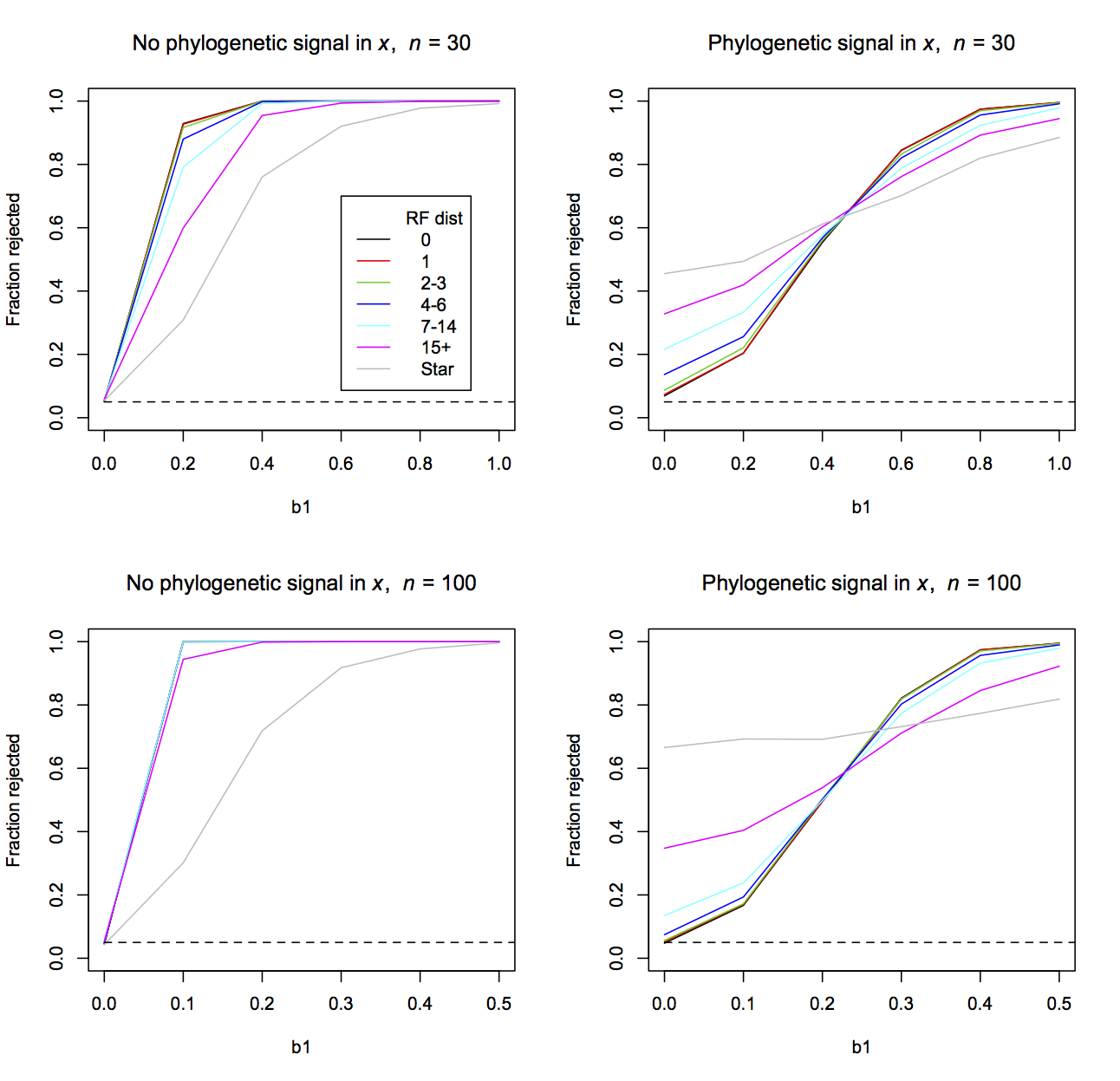Fig. 3.10: Power curves for tests of the coefficient b1 in a phylogenetic regression with permutations in the topology of the phylogenetic tree. Datasets of n = 30 and 100 species were simulated up a phylogenetic tree under Brownian motion evolution without (left panels) or with (right panels) phylogenetic signal in x given by Brownian motion evolution. The data were fit using the initial phylogeny (black lines). Permutations of the initial phylogeny were then generated and compared to the initial phylogeny using the RF distance. Single permutation trees were selected in the following bins: RF = 1, 2-3, 4-6, 7-14, and 15+. These permutation phylogenies were then used in fitting the model to the data, thereby mimicking mistakes in the phylogenetic of differing degrees depending on RF. Finally, the data were fit to a LM that assumes there is no phylogenetic signal in the residual errors (a star phylogeny). Two-thousand simulations were performed for each level of b1 = 0, 0.2, 0.4, 0.6, 0.8 and 1.0, and the rejection rates were calculated with phylolm() (Pagel’s λ model) using a Wald test. As the number of mistakes in the topology of the phylogeny increases, the type I errors and power become qualitatively more similar to the case when phylogenetic signal in the residual errors is ignored (gray line in Fig. 3.10 labelled “Star” given by a LM model). When there is no phylogenetic signal in x, power is lost with increasing numbers of topological mistakes. When there is phylogenetic signal in x, type I errors become more inflated with increasing numbers of mistakes. When n = 30, a single topological mistake (red lines) makes little difference, although even 2-3 topological mistakes can noticeably inflate the type I errors when there is phylogenetic signal in x. The same number of topological mistakes has less effect when n = 100, which is not surprising, because with more species the same number of topological mistakes leaves more of the initial phylogeny intact. Problems with type I errors and power that we have encountered in this book up to now can be overcome using bootstrapping. To account for uncertainty in the topology of phylogenies, it is necessary to bootstrap over the possible topologies, weighing the topologies according to their likelihoods (Martins 1996). As phylogenies are getting more and more common, and better and better, this issue will hopefully recede. Also, if the topological mistakes are few, they have small impact on type I errors and power. Nonetheless, the issue of topological mistakes in a phylogeny should be recognized and considered in analyses of comparative data. ### 3.8 Phylogenetic regression for binary data There are two general approaches for fitting phylogenetic models to binary data. The first uses a logit normal-binomial model in which the normal distribution is multivariate with a covariance given by Brownian motion evolution. This phylogenetic generalize linear mixed model (PGLMM) is similar to the GLMMs discussed in chapters 1 and 2. The second approach is based on logistic regression. Because a discussion of phylogenetic methods for binary data is given in Ives and Garland (2014), I will be brief here. #### 3.8.1 Binary PGLMM The structure of the binary PGLMM is like the binary GLMM, but with e having phylogenetic signal given by Brownian motion evolution: Z = b0 + b1*x + e p = inv.logit(Z) Y ~ binom(n, p) e ~ norm(0, s2*V) Here, because it is possible for s2 to be zero, there is no need to include a branch-length transform for the covariance matrix V; if there is phylogenetic signal, then s2 will be greater than zero. Although this model has a relatively simple structure, there are few off-the-shelf methods for fitting it. In the package ape, the function binaryPGLMM() fits this model using a combination of penalized quasi-likelihood and REML (Ives and Helmus 2011); a faster version of binaryPGLMM() written in C++ is in the package phyr. Because it uses quasi-likelihoods, binaryPGLMM() doesn’t give a likelihood, which makes likelihood ratio tests impossible. Nonetheless, it gives inference about the regression coefficients using Wald tests. I also performed a parametric bootstrap test of H0:b1 = 0. Because binaryPGLMM() does not give log likelihoods, I used the following procedure that bootstraps the parameter value of interest (b1) under H0:b1 = 0 rather than the LLR: 1. Fit the model to the data and calculate the value of the parameter of interest (in this case, b1). 2. Refit the model without the parameter (without x1) and use the resulting parameter values to simulate a large number of datasets. 3. Refit the model including the parameter of interest (b1) for each dataset and collect these values. 4. The resulting distribution of parameters estimated from the simulated datasets approximates the distribution of the estimator of the parameter under H0:b1 = 0, allowing P-values to be calculated. For a two-tailed test, the P-values are twice the fraction of simulated parameter values that exceed the value estimated from the data with the full model (step i). The binary PGLMM can also be fit using Bayesian methods, such as in the package MCMCglmm (Hadfield 2010). #### 3.8.2 Phylogenetic logistic regression Logistic regression is identical to a binary GLMM when the errors aren’t correlated. Nonetheless, in phylogenetic logistic regression (PLOG) the phylogenetic covariances are incorporated differently from PGLMM. In PLOG models, the value of a binary trait (0 or 1) changes as it evolves up a phylogenetic tree (Ives and Garland 2010), and this can be used to calculate the likelihood. Logistic regression in general gives regression coefficients that are biased upwards; this was seen in figure 2.6 (subsection 2.6.3). A good way to correct for this bias is to apply a penalty to the likelihood function (Firth 1993, Ives and Garland 2010), and PLOG as implemented by phyloglm() use this Firth penalized likelihood. Finally, the effects of the predictors x are added after the evolution up the phylogenetic tree; these changes caused by x occur as an instantaneous switch from 0 to 1, or 1 to 0, depending on the values of x and the regression coefficients. #### 3.8.3 Type I errors and power To investigate type I errors and power, I performed a study using a binary PGLMM to simulate data for the cases in which x does not and does have phylogenetic signal (Fig. 3.11). Both the binary PGLMM (binaryPGLMM()) and PLOG (phyloglm()) showed inflated type I errors when x had phylogenetic signal, with PLOG being particularly bad. The inflated type I errors were of course corrected using the bootstrap under H0:b1 = 0. The bootstrapped PGLMM had greater power than the bootstrapped PLOG; this might be the consequence of the data having been simulated using a PGLMM model. Finally, unlike the case of hierarchical data in which LMs and LMMs often performed well on binary data, in this case the continuous PGLS (phylolm()) either had inflated type I errors (when x had no phylogenetic signal) or very low power (when x had phylogenetic signal).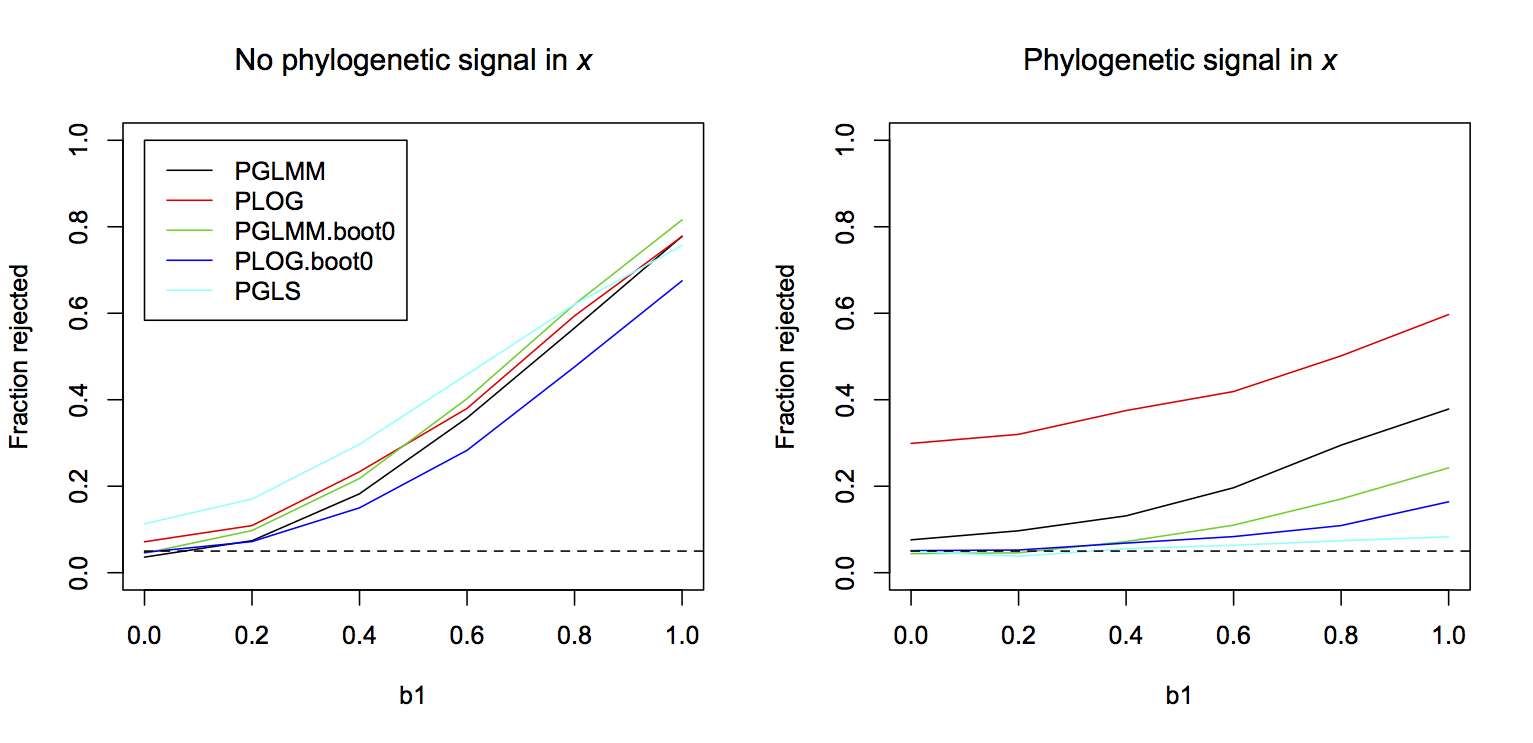Fig. 3.11: Power curves for tests of the coefficient b1 in a PGLMM for binary data when there is no phylogenetic signal in x (left panel) and when there is (right panel). The data were simulated with n = 50 species and b0 = 0. A single phylogeny was used for all simulations. Two-thousand simulations were made at each value of b1 = 0, 0.2, 0.4, 0.6, 0.8, and 1.0. The significance of b1 was assessed by fitting each dataset with a binary PGLMM (binaryPGLMM()) and PLOG (phyloglm()) using Wald tests, binary PGLMM and PLOG using bootstraps under H0:b1 = 0, and a PGLS (phylolm()) that does not account for the binary nature of the data. Finally, one thing to note about these simulations is that the power of all the methods with proper type I error control was low when there was phylogenetic signal in x, even though the sample size was n = 50, rather than n = 30 as in analyses presented previously for continuous data (Fig. 3.9). This is a reflection of the fact that there is not as much information in binary data as in continuous data, and phylogenetic signal in the residual errors and x compounds this lack of information. ### 3.9 Summary 1. Phylogenetic comparative methods involve models that explicitly include the hypothesis that phylogenetically related species are more likely to have similar trait values. Incorporating branch-length transforms, such as Pagel’s λ and OU transforms, gives a way to test this hypothesis. In regression analyses for phylogenetic data, branch-length transforms should be included to let the data say whether there is phylogenetic signal in the residual errors. 2. Estimating the strength of phylogenetic signal involves estimating covariances in the data. Estimating covariances is sometimes harder statistically than estimating regression coefficients, and therefore this requires caution. Also, phylogenetic covariances can take different forms, and therefore you should anticipate that one branch-length transform might fit the data better than others. 3. All of the issues that can corrupt results from analyses of hierarchical data also apply to phylogenetic data. In particular, don’t trust the P-values that are reported even from established methods. 4. As found for hierarchical data, failing to account for phylogenetic correlation can lead to badly inflated type I errors (false positives). This occurs especially when there is phylogenetic signal in x. 5. Most phylogenetic comparative analyses are performed under the assumption that the topology of the phylogeny is correct. When it is not correct, increasing topological mistakes increase the statistical problems that are found when phylogenetic signal is ignored altogether. 6. Phylogenetic models can be formulated for binary data. These models experience the same challenges as models for continuous data, with the challenges exacerbated by the lower information content of binary data. ### 3.10 Exercises 1. Construct the right panel of figure 3.8 giving power curves for the detection of phylogenetic signal in simulated data using an OU transform. You can modify the code I provided for the left panel which simulates data using Pagel’s λ transform. 2. The parametric bootstrap of H0 for phylogenetic signal (subsection 3.5.3) uses the log likelihood ratio (LLR) as the test statistic. It is also possible to use the phylogenetic signal parameter (λ or α) as the test statistic. This would involve the following: (i) Fit the model to the data and calculate the value of the phylogenetic signal parameter (λ or α). (ii) Refit the model with no phylogenetic signal and use the resulting parameter values to simulate a large number of datasets. (iii) Refit the model including the phylogenetic signal parameter (λ or α) for each dataset and collect these values. (iv) The resulting distribution of λ or α estimated from the simulated datasets approximates the distribution of the estimator of λ or α, allowing P-values to be calculated. Perform this bootstrap and compare the results to the bootstrap of H0 using the LLR. 3. In subsection 3.6.1 I showed that, if phylogenetic signal in the residual errors is caused by unmeasured traits with phylogenetic signal, then increasing the number of unmeasured traits doesn’t degrade the phylogenetic signal. In other words, the signals from the unmeasured traits don’t cancel each other out. Perform a simple simulation with three unmeasured traits to show this is true. ### 3.11 References Bartoszek K., Pienaar J., Mostad. P., Andersson S. and Hansen T.F. 2012. A phylogenetic comparative method for studying multivariate adaptation. Journal of Theoretical Biology 314:204-215 Blomberg S.P., Garland T. Jr., and Ives A.R. 2003. Testing for phylogenetic signal in comparative data: behavioral traits are more labile. Evolution 57:717-745. Butler M.A. and King A.A. 2004. Phylogenetic comparative analysis: a modeling approach for adaptive evolution. American Naturalist 164:683-695. Efron B. and Tibshirani R.J. 1993. An introduction to the bootstrap. Chapman and Hall, New York. Firth D. 1993. Bias reduction of maximum likelihood estimates. Biometrika 80:27-38. Hadfield J.D. 2010. MCMC methods for multi-response generalized linear mixed models: the MCMCglmm R package. Journal of Statistical Software 33:1-22. Hansen T.F. and Orzack S.H. 2005. Assessing current adaptive and phylogenetic inertia explanations of trait evolution: the need for controlled comparisons. Evolution 59:2063-2072. Ho L.S.T. and Ane C. 2014. A linear-time algorithm for Gaussian and non-Gaussian trait evolution models. Systematic Biology, 63(3):397-408. Ives A.R., Midford P.E., and Garland T. Jr. 2007. Within-species variation and measurement error in phylogenetic comparative methods. Systematic Biology 56:252-270. Ives A.R. and Garland T. Jr. 2010. Phylogenetic logistic regression for binary dependent variables. Systematic Biology 59:9-26. Ives A.R. and Garland T. Jr. 2014. Phylogenetic regression for binary dependent variables. Pages 231-261 in L. Z. Garamszegi, editor. Modern Phylogenetic Comparative Methods and Their Application in Evolutionary Biology. Springer-Verlag, Berlin Heidelberg. Ives A.R. and Helmus M.R. 2011. Generalized linear mixed models for phylogenetic analyses of community structure. Ecological Monographs 81:511-525. Martins E.P. 1996. Conducting phylogenetic comparative studies when the phylogeny is not known. Evolution 50:12-22. Martins E.P. and Hansen T.F. 1997. Phylogenies and the comparative method: A general approach to incorporating phylogenetic information into the analysis of interspecific data. American Naturalist 149:646-667. Pagel M. 1997. Inferring evolutionary processes from phylogenies. Zoologica Scripta 26:331-348. Pagel M. 1999. Inferring the historical patterns of biological evolution. Nature 401:877-884. Paradis E., Claude J. and Strimmer K. 2004. APE: analyses of phylogenetics and evolution in R language. Bioinformatics 20: 289-290 Rangel T.F., Colwell R.K., Graves G.R., Fucikova K., Rahbek C., and Diniz J.A.F. 2015. Phylogenetic uncertainty revisited: Implications for ecological analyses. Evolution 69:1301-1312. Revell L.J. 2010. Phylogenetic signal and linear regression on species data. Methods in Ecology and Evolution 1:319-329. Revell L.J. 2012 phytools: An R package for phylogenetic comparative biology (and other things). Methods in Ecology and Evolution 3: 217-223. Robinson D.F. and Foulds L.R. 1981. Comparison of phylogenetic trees. Mathematical Biosciences 53:131-147. ## Chapter 4: Phylogenetic Community Ecology ### 4.1 Introduction There is growing interest in using phylogenies to explore patterns of community composition – how species are distributed with respect to each other and with respect to environmental gradients in space. The distribution of species among communities depends in part on their traits. For example, in high-elevation habitats plant species that are tolerant of cold and snow are more likely to occur, and tolerance to cold winters depends on numerous adaptive traits. Similarly, fish species that are tolerant of low pH are more likely to occur in acidic lakes, and acid tolerance involves many fish traits. Since many traits are phylogenetically correlated among species, you would expect the distribution of species among communities to reflect, at least in part, phylogenies, with closely related species more likely to occur in similar communities. This is called phylogenetic attraction. Conversely, phylogenetically closely related species may be less likely to occur in the same communities. This might occur, for example, if closely related species with similar resource requirements are more likely to compete with each other, leaving only one present in a community. This pattern of phylogenetic repulsion could also arise through apparent competition with shared predators, with the more susceptible of a group of phylogenetically related prey species getting extirpated from communities by the predators. Thus, there are numerous ways in which phylogenies might be expected to be seen in patterns of community composition. Phylogenetic community ecology uses the information available in phylogenetic relationships to understand community composition among spatially separated sites. Phylogenetic information is often used as a surrogate for missing information about variation in trait values among species, and missing information about how these traits affect the presence and abundance of species in communities. I am interested in the statistical problem of identifying these phylogenetic patterns. I think the problem of trying to infer the biological processes underlying these patterns is far more challenging. For example, if species show the pattern of phylogenetic repulsion, with closely related species less likely to occur in the same communities, I don’t think that this provides compelling evidence that competition is involved in structuring communities. However, it does give license for more detailed studies to see if competition is important. Phylogenies are also sometimes interpreted as surrogates for unmeasured traits: if there are phylogenetic patterns in community composition, then there must be traits underlying these patterns. I think this is likely to be correct, although it is necessary to be cautious, because the traits that you suspect are involved might in fact not be. My philosophy is to look for patterns and make sure they are statistically well-founded. If they are, then start the biological work to try to understand the processes driving the patterns. Data on phylogenetic community composition are both hierarchical and phylogenetic. Therefore, the data are correlated. They combine characteristics of hierarchical data discussed in Chapters 1 and 2, and phylogenetic data discussed in Chapter 3. The approach I take (which should be no surprise) is to model the distribution of species among communities. There is also a rich history in community ecology of using metrics that measure a pattern of interest and then using permutation tests to determine if these patterns are statistically significant. This approach of using metrics and permutation tests can be very useful and powerful for simple problems. However, as problems become more complex, they become less useful and informative. Furthermore, because the metric approach does not produce fitted models that can simulate data, the results can be very difficult to assess: do the results really answer the questions you are asking? Building models for community composition is in some ways statistically more challenging, and requires greater computing power, but the payoff is greater flexibly in the questions that can be asked, and sometimes greater confidence in the answers. This chapter presents models of phylogenetic community composition as a series of examples of increasing complexity. The models are mostly Phylogenetic Linear Mixed Models (PLMMs) that incorporate both hierarchical and phylogenetic covariance matrices into LMMs for continuous (abundance) data. At the end of the chapter I’ll also present Phylogenetic Generalized Linear Mixed Models (PGLMMs) for binary (presence/absence) data. The chapter explains how to construct and test models for a range of different types of problems. I’ll begin by asking whether there is phylogenetic signal in the composition of communities. Are phylogenetically related species more or less likely to occur in the same site? This question assumes you have no information about either traits shared by species or environmental differences among sites. The second question is related to the first. If you have information about environmental differences among sites, can you account for these differences to reveal phylogenetic patterns in the distribution of species that otherwise would be obscured. The third question is, if you have trait information about species but not about the environment, does this trait information explain the phylogenetic patterns in the distribution of species among sites. Answering this question requires a statistical test of whether the phylogenetic signal in the distribution of species is explained by a set of traits that you have measured. The fourth question assumes you have both trait and environmental information, and asks whether together these can explain the distribution of species among sites. Thus, the first four questions assume you do or don’t have trait and environmental information, in all 2-by-2 combinations. The final question assumes that the sites themselves have a phylogeny. This is the case, for example, if the communities are pollinators and the sites are the plants they visit, with the plants having a phylogeny. Similar cases are diseases distributed among hosts, and predators attacking a smorgasbord of prey species. Questions that can be asked of such bipartite data (having two groups like pollinators and plants that interact with each other) include the presence of phylogenetic signal from one or both of the groups, and the role of traits in generating these phylogenetic signals. I’ll address these five questions assuming that information is known about species abundances in each site. The questions could also be asked if only presence/absence of species in sites were known. Therefore, I’ll end the chapter with a re-examination of the first question using presence/absence (binary) data. Binary data for phylogenetic community composition involves the same issues that we have seen for binary data in hierarchical and phylogenetic data in Chapters 1-3: they contain less information than abundance data and introduce greater mathematical and computational challenges. There are few statistical toolboxes for building models of phylogenetic community composition. This chapter relies heavily on the package phyr and its function communityPGLMM(). The math underlying this function was developed for fitting models of community composition (Ives and Helmus 2011) and then expanded to the case of bipartite phylogenies (Rafferty and Ives 2013). An older version of communityPGLMM() is in the package pez. Daijiang Li updated communityPGLMM() for phyr, and it has a nicer syntax and is much faster (running in C++). Also, Russell Dinnage has added Bayesian capabilities to communityPGLMM(), although I will use it only in frequentist mode. In some respects, this chapter serves as the manual for communityPGLMM(). ### 4.2 Take-homes 1. Phylogenetic models of community composition combine approaches for dealing with data having hierarchical structure and phylogenetic structure. Thus, PLMMs and PGLMMs incorporate two types of correlations in data. 2. PGLMMs can explain the distribution of species among communities using only information about phylogenies. However, these models can also flexibly include information about traits shared by species and environmental factors shared by communities. 3. Hierarchical and phylogenetic correlations present the same challenges as hierarchical data (Chapters 1 and 2) and phylogenetic data (Chapter 3). Failing to account for the correlated structure of the data can lead to inflated type I errors (false positives) or reduced power (false negatives). 4. Phylogenetic community models can be used to test which traits and which environmental factors are responsible for phylogenetic patterns in the data. They can test hypotheses for why the phylogenetic patterns exist. 5. Although PGLMMs provide flexible tools for testing a wide range of hypotheses in a diverse collection of community ecology data, there are limits to the size of datasets they can reasonably handle. For complex hypotheses involving many covariance patterns, fitting PGLMMs with more than 1000-2000 species-site data points will be computationally slow. ### 4.3 Phylogenetic patterns in community composition The first question I’ll address is how to detect phylogenetic signal in the distribution of species among sites (communities) in the absence of information about trait characteristics of species and environmental characteristics of sites. To set up this problem, I’ll assume that species differ in mean abundance across all sites, that sites differ in the mean abundance of species they contain, and that there is phylogenetic attraction, with phylogenetically related species being more likely to occur in the same site. Below, I’ll first present the model and then investigate type I errors and statistical power. #### 4.3.1 A PLMM for phylogenetic attraction The code for simulating and fitting a PLMM for phylogenetic attraction is similar to that for a LMM. Here, I’ll assume that abundances of species are known in every site, and Y gives these abundances, possibly transformed. I’ll print the R code for simulating and fitting the model, and then explain it, so don’t worry if a few things are opaque. communityPGLMM() is a hierarchical mixed model that contains covariance matrices not only for the hierarchical structures in the data, but also phylogenies. The best way of explaining the model is with pictures, and the function communityPGLMM.plot.re() shows these. If show.image=T, it shows images of the covariance matrices for the random effects in the model, and if show.sim.image=T it gives a simulated example for each separate random effect. It also returns the covariance matrices in vcv and the simulated data in sim. For the figures in this chapter, I’ve used communityPGLMM.plot.re() to generate the matrices vcv and sim, and then plotted the matrices outside of communityPGLMM.plot.re(), because this allowed me to format the figures more appropriately for a book.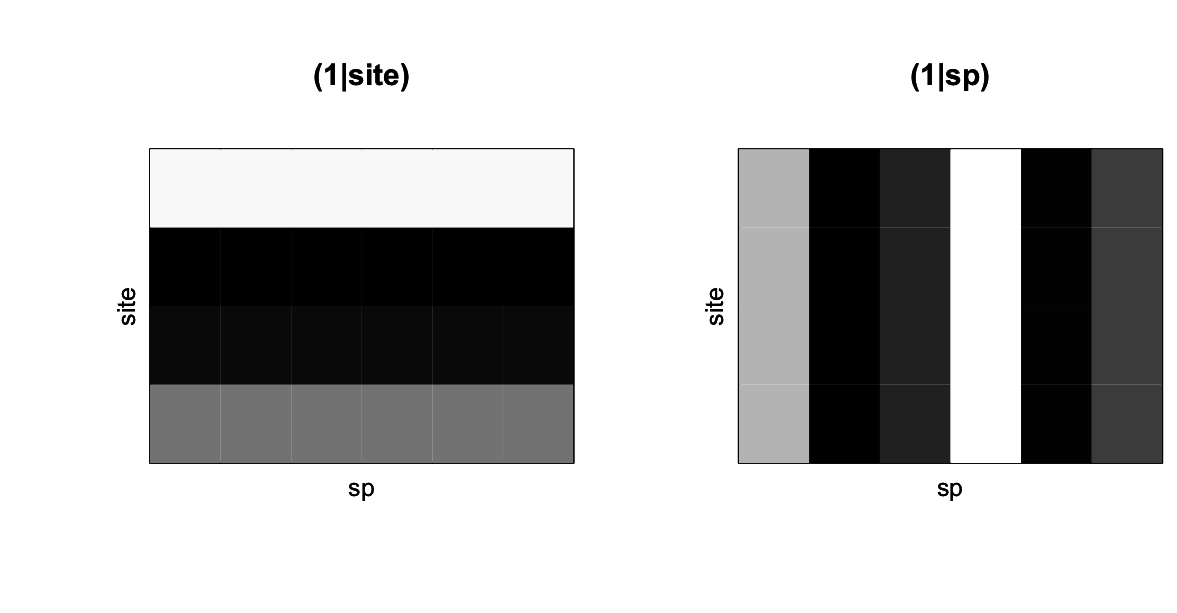Fig. 4.1: Simulated abundances for the random effects (1|site) and (1|sp) from communityPGLMM.plot.re() as would be produced with show.sim = T. Figure 4.1 shows the simulated abundances of six species among four sites, with whiter corresponding to higher values. The panel (1|site) gives the simulated mean abundances of species for each of the sites, and corresponds to the term (1|site) in the communityPGLMM() model. This term encapsulates the random effect that takes on a different value for each site. The panel (1|sp) gives a simulation of the random effect for species; each species is assigned at random a mean abundance across all sites. This term does not appear explicitly in the communityPGLMM() model, but it is there implicitly. In the model, the term (1|sp__) denotes a phylogenetic random effect for species, and when it is included, the non-phylogenetic effect (1|sp) is automatically included as well. In communityPGLMM() syntax, the double underscore __ denotes a phylogenetic random effect. Whenever a phylogenetic random effect is included, its corresponding non-phylogenetic random effect is included too. I’ll explain why a little later.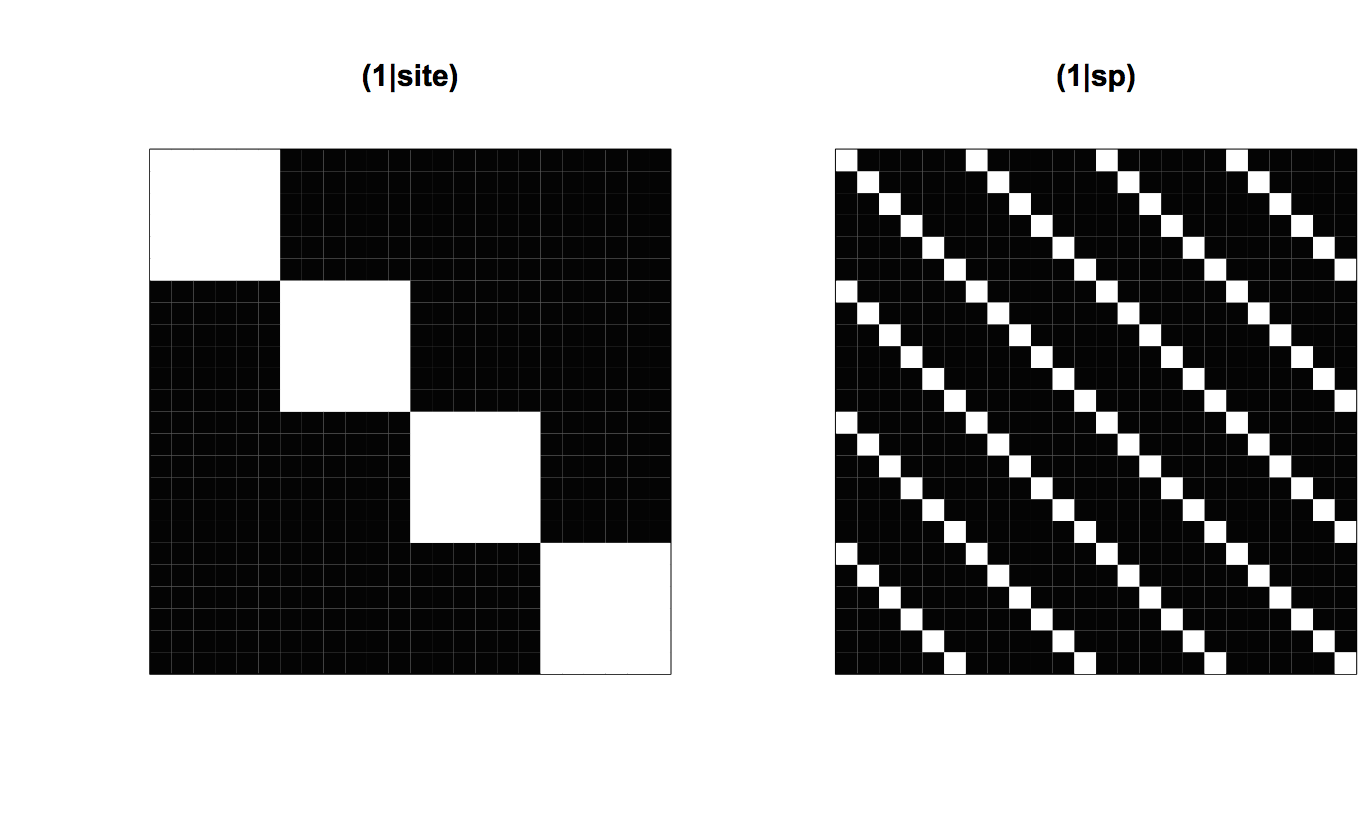Fig. 4.2: The covariance matrices for the random effects (1|site) and (1|sp) from communityPGLMM.plot.re() as would be produced with show.image = T. To see the structure of the model, it is necessary to look at the covariance matrices. Figure 4.2 shows the 24 x 24 element covariance matrices for (1|site) and (1|sp), with white showing high covariances. In fact, the covariances are either 1 or 0. The rows and columns of the covariance matrices are sorted so that species are nested within sites. Therefore, (1|site) has a block-diagonal form with 6 x 6 blocks of ones which correspond to the six species in the same site. (1|sp) contains 1s for each element corresponding to the same species in the 4 different sites. These covariance matrices are the same as typically found in mixed models that account for the hierarchical nature of the data.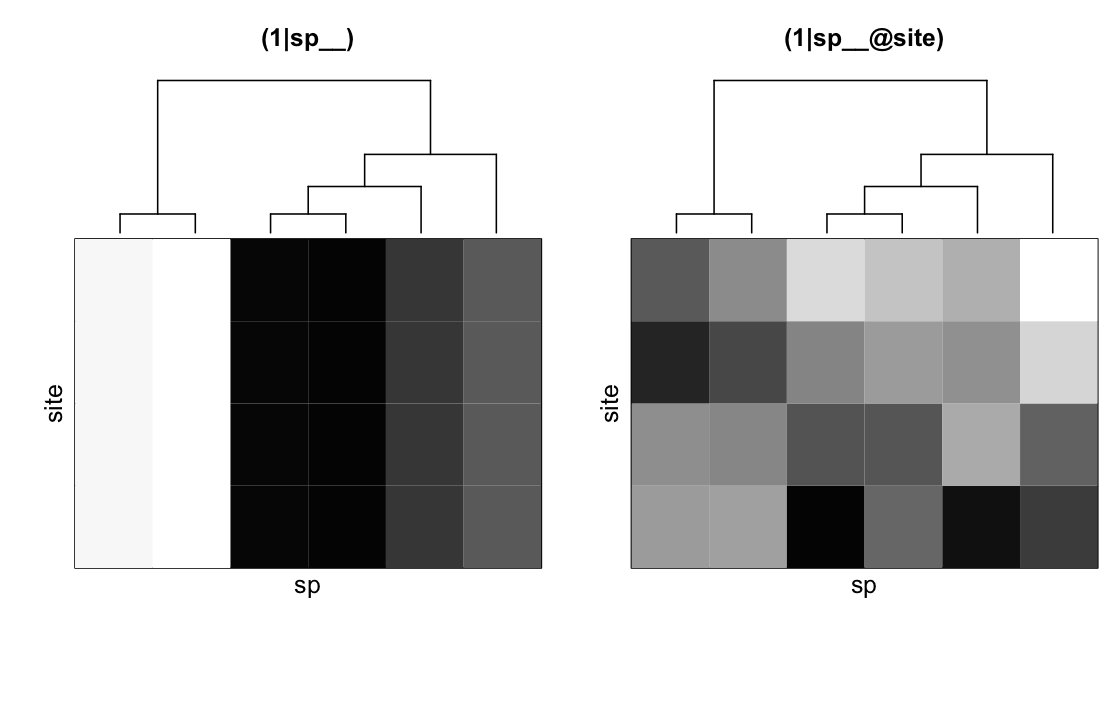Fig. 4.3: Simulated abundances for the random effects (1|sp__) and (1|sp__@site) from communityPGLMM.plot.re() as would be produced with show.sim = T. The two additional covariance matrices in the model give phylogenetic patterns in community composition. (1|sp__) gives the variation in mean abundance of species that has a phylogenetic signal (Fig. 4.3, left panel); this can be seen for the second and third species from the left that are phylogenetically closely related and have low mean abundances. (1|sp__@site) (Fig. 4.3, right panel) is phylogenetic attraction. Phylogenetically related species are more likely to occur in the same sites, although this is not due to their both having high overall abundances across all sites. Phylogenetic attraction just describes the case when related species are likely to both have low or both have high abundance in the same site. The two species on the left of (1|sp__@site) exemplify this pattern, with both being particularly (though randomly) low in the second site. Phylogenetic attraction in the covariance matrix V in the simulation code is created using the Kronecker product of two matrices (the function kronecker()). This function takes the second matrix (in this case, Vphy) and replicates it according to the first matrix (the identity matrix with the number of diagonal elements equal to the number of sites). The function is clarified in the discussion of (1|sp__@site) that follows. In the code for simulating the data, there is a line This standardization is used to give a known value of the determinant of the covariance matrix regardless of the overall branch lengths of the input matrix phy. For example, a phylogeny might have branch lengths measured in genetic distance or millions of years of separation between species, and the value of branch lengths will be very different. The determinant measures the overall size or volume of a matrix, and therefore it gives a natural way to standardize matrices. The determinant is standardized to 1, because all identity matrices (for independent data) have determinants of 1. communityPGLMM() automatically performs this standardization, so that the values of the phylogenetic random effects (the phylogenetic variances) can be interpreted alongside the values of the non-phylogenetic random effects.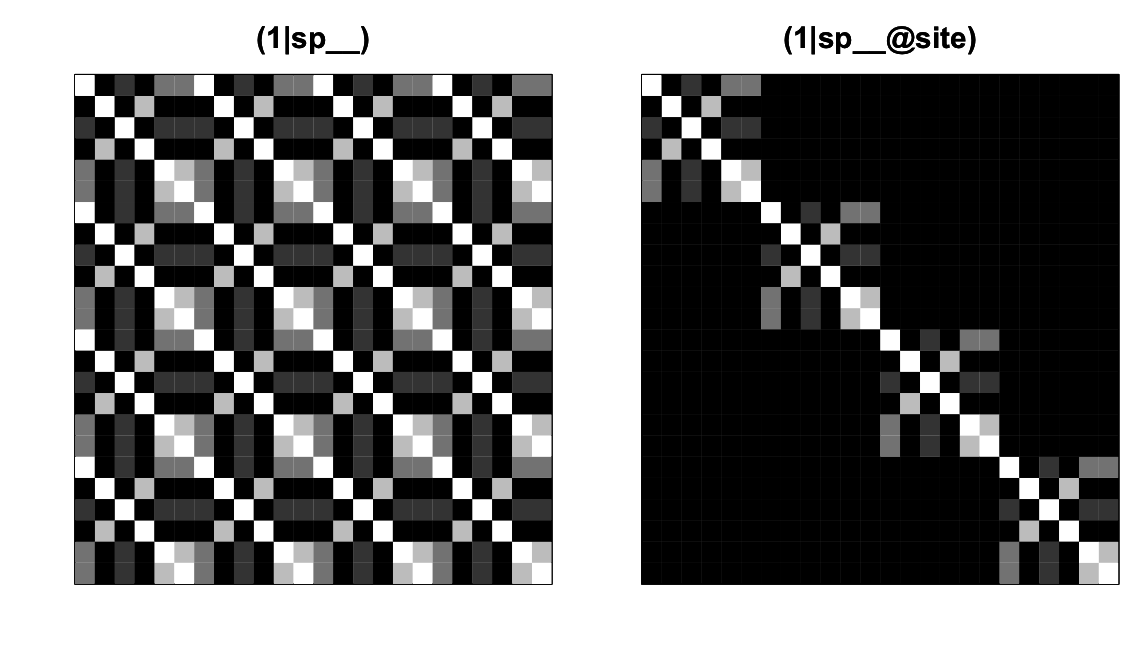Fig. 4.4: The covariance matrices for the random effects (1|sp__) and (1|sp__@site) from communityPGLMM.plot.re() as would be produced with show.image = T. The covariance matrices for (1|sp__) and (1|sp__@site) are given in figure 4.4, where like figure 4.2 species are nested within sites. The covariance matrix for (1|sp__) is densely populated: the covariance matrix for the phylogeny, Vphy, is tiled over the entire matrix. This occurs because (1|sp__) gives phylogenetic patterns in the mean abundance of species: if a species is common in one site, then a phylogenetically closely related species will likely have high abundance not only in this site, but also in any site. In contrast to (1|sp__), (1|sp__@site) gives phylogenetic attraction in which high abundance of a species in one site will only be associated with high abundance of a phylogenetically closely related species in the same site. These comparisons among covariance matrices explain why inclusion of a phylogenetic random effect requires the inclusion of non-phylogenetic effects. For (1|sp__), the covariance between the same species in different sites is 1; this is also true of (1|sp), but for (1|sp) all other elements are 0. If (1|sp__) were included in a model without (1|sp), then any variation in mean abundance of species across all sites would potentially be picked up by (1|sp__) and might falsely be ascribed to phylogenetic signal. Including (1|sp) absorbs this species-specific variation so that tests of (1|sp__) only involve the phylogenetic covariances between species, rather than also the variances within species. Similarly, (1|sp__@site) has positive covariances in blocks along the diagonal of the covariance matrix, and so it has a similar structure to (1|site). If (1|site) were not included in the model, then any site-to-site variation in mean abundance of species might be ascribed to phylogenetic attraction. Even though (1|site) should most often be included in a model that contains (1|sp__@site), communityPGLMM() does not do this automatically, so you have to do it yourself. Unfortunately, including (1|sp) and (1|site) in the model increases the number of parameters that must be estimated, but they are necessary to prevent inflated type I errors in detecting phylogenetic patterns. Finally, note that there are two phylogenetic patterns, one in the mean abundance of species and the other in the covariance in abundances of species in the same sites (phylogenetic attraction). These two phylogenetic patterns underscore the advantage of a modeling approach that can separate these two phylogenetic effects. It is difficult to derive a metric and permutation test for this pattern. The problem of different phylogenetic patterns multiplies when sites have their own phylogenies in bipartite data. The output of communityPGLMM() is similar to lmer(), giving random effects and fixed effects. For this particular simulation, the estimates of all random effects were considerably larger than 0, including the two phylogenetic terms (1|sp__) and (1|sp__@site): The P-values for the fixed effects can obtained from the Wald tests or LRTs, while P-values for the random effect can be obtained by LRTs. These are illustrated in the next subsection. #### 4.3.2 Type I errors and power To investigate the ability of the PLMM to statistically identify phylogenetic attraction, I performed a simulation in which the strength of phylogenetic attraction was increased by increasing sd.attract, the parameter scaling the magnitude of the phylogenetic attraction covariance matrix. I then tested the null hypothesis that the estimate of sd.attract is zero with a LRT. I used two methods to determine the significance of the LRT: the standard chi-square approximation to the distribution of the deviance (twice the difference in log likelihoods between full and reduced models), and a bootstrapped distribution of the deviance under the H0. The code for these two tests is The critical threshold value for the deviance in the bootstrap test, LRT.crit, is obtained by performing bootstrap simulations of the data using the following procedure, as was done for similar bootstrap tests in subsections 2.6.4 and 3.5.3: 1. Using the estimated values of the reduce model with sd.attract = 0 fit to the data, simulate a large number of datasets (e.g., 2000 for an alpha significance level of 0.05). 2. Refit both full (including sd.attract) and reduced models to the simulated datasets, and calculate the LLRs and the corresponding deviances. 3. LRT.crit is the value which is exceeded by 0.05 of the bootstrapped values of the deviance. Note that I’ve used ML for fitting the models, specifying REML=F; the default is REML=T. For performing a LRT on the covariance matrices, either ML or REML can be used for tests of random effects. When comparing fixed effects, though, only ML can be used, and therefore you should take care in which you are using. For this particular model, tests of the random effects using ML and REML give the same results. As shown in figure 4.5, the standard LRT with the chi-square approximation has deflated type I errors: only 0.023 of the simulated datasets were rejected. This implies that the P-values from the standard LRT are too high. The LRT with bootstrapped P-values under the null hypothesis H0 had correct type I errors and greater power than the standard LRT. Although it is always preferable to perform a bootstrap test under H0, for large datasets this becomes computationally challenging. Fitting a single simulated dataset with 50 species and 100 sites took 17 minutes on my old Macintosh, so a bootstrap with 2000 bootstrapped datasets would take 24 days. Because of the mathematics underlying the statistical analyses of communityPGLMM(), models that include nested terms like (1|sp__@site) run more slowly than models with only non-nested terms like (1|sp__). The standard LRT based on the chi-square approximation luckily has deflated rather than inflated type I errors. Therefore, if you use the standard LRT, it won’t give you P-values that are too low and increase you chances of falsely claiming a significant result. There is a moderate reduction in power, but this is far less serious than inflated type I errors.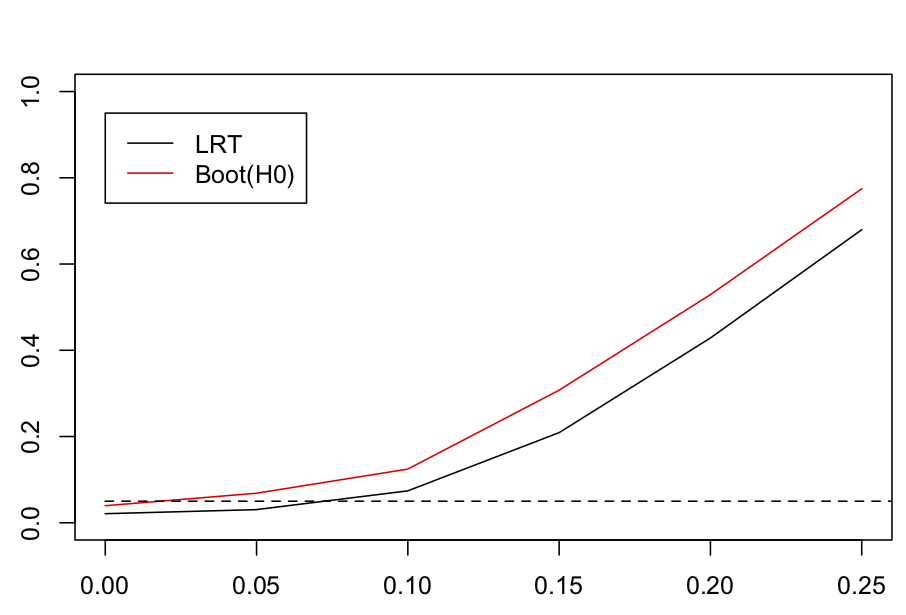Fig. 4.5: Power curves for tests of phylogenetic attraction. For each set of 2000 simulated datasets at values of sd.attract = 0, 0.05, 0.1, 0.15, 0.2 and 0.25, the significance of sd.attract was tested with a standard LRT (LRT) and with a parametric bootstrap test of H0, Boot(H0). ### 4.4 Phylogenetic repulsion Phylogenetic repulsion is the opposite pattern from phylogenetic attraction, with phylogenetically related species less likely to have high abundance in the same site. Unlike phylogenetic attraction, when a phylogenetic covariance matrix generated under the assumption of Brownian motion evolution can be used to describe the possible positive covariances in species abundance, phylogenetic repulsion involves negative covariances in the abundances of related species. One way to derive a sensible covariance matrix for repulsion is to use the matrix inverse of the phylogenetic covariance matrix, V. The justification for this can be derived by assuming that species are competitors whose population dynamics follow a Lotka-Volterra competition model. If the competition coefficients that give the strength of competition between each pair of species are assumed to equal the elements of V, so that related species compete more with each other, then their relative abundances at equilibrium will be given by the inverse of V (Ives and Helmus 2011, appendix A). A challenge in detecting phylogenetic repulsion is that phylogenetically related species may be more likely to occur in the same sites because they share values of a trait that make the site suitable for both of them (Helmus et al. 2007). Therefore, environmental differences among sites might obscure phylogenetic repulsion. This can be taken into account by including both phylogenetic repulsion and environmental factors in the same PLMM. #### 4.4.1 Phylogenetic repulsion PLMM I simulated data with phylogenetic covariances in the mean abundance of species among sites. This was just to make the data look more realistic, since in real datasets there is generally large variation among the mean abundances of species. I selected a value for an environmental variable env from a normal distribution and assumed that the responses of species to env depend on an unmeasured trait trait; specifically, for species i and site j, the effect of env on abundance depends on trait(i) * env(j). The trait is phylogenetically correlated among species, giving the case in which related species respond to the environment in a similar way. Phylogenetic repulsion is incorporated using the inverse of the phylogenetic covariance matrix; the function solve() gives the inverse. After simulating the data, communityPGLMM() is used to fit them. In the communityPGLMM() model output, (1|site) gives the variation among sites in the mean abundance of species they contain, (1|sp) and (1|sp__) give non-phylogenetic and phylogenetic variation in mean species abundances, (env|sp) and (env|sp__) give the non-phylogenetic and phylogenetic response of species to env, and (1|sp__@site) gives phylogenetic repulsion. The nested random effect (1|sp__@site) is specified as giving repulsion rather than attraction by the statement repulsion=T. The covariance matrices for the model are depicted in figure 4.6 using communityPGLMM.plot.re() to generate covariance matrices. The three covariance matrices (1|site), (1|sp) and (1|sp__), are comparable to those in the model for phylogenetic attraction (Figs. 4.2 and 4.4), while (env|sp), (env|sp__), and (1|sp__@site) require explanation.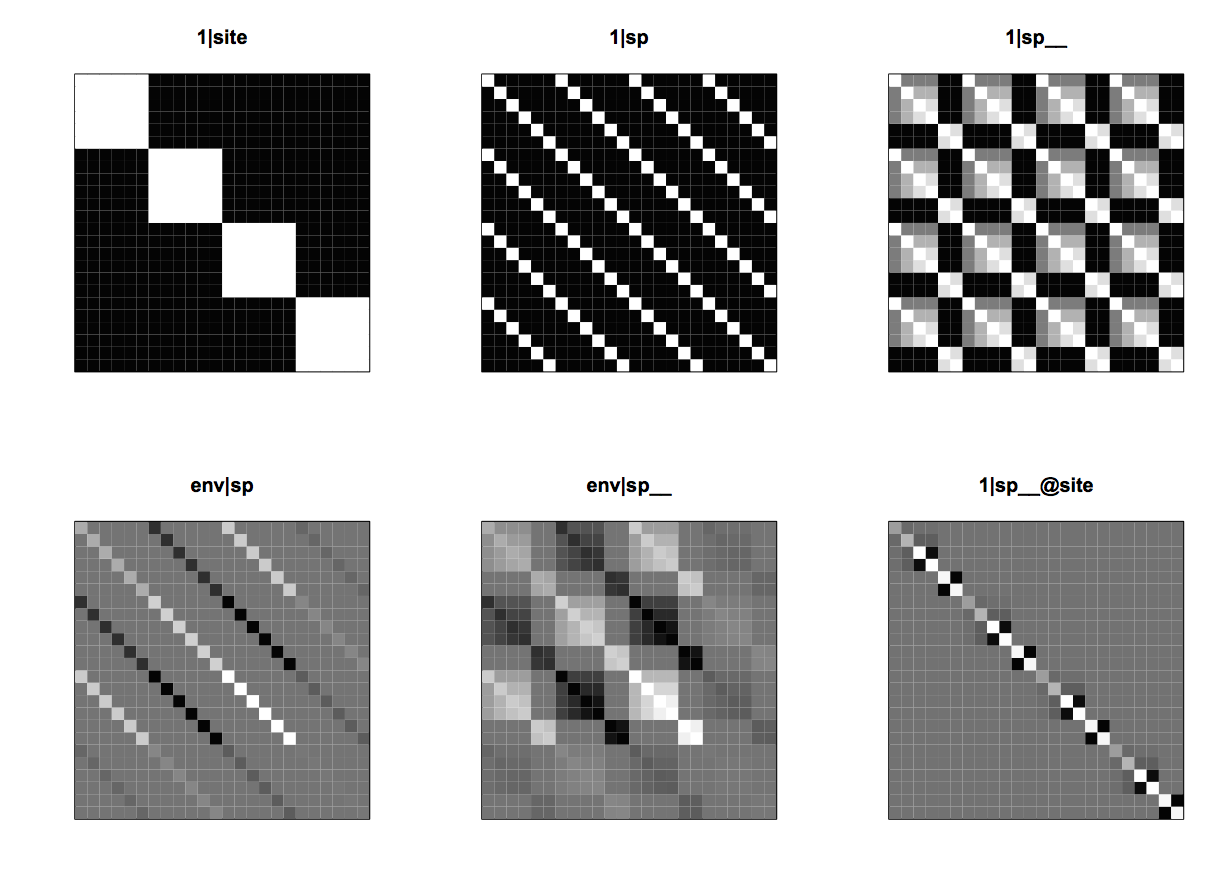Fig. 4.6: The six covariance matrices in the model for phylogenetic repulsion. (1|site) gives the variation among sites in the mean abundance of species they contain, (1|sp) and (1|sp__) give non-phylogenetic and phylogenetic variation in mean species abundances, (env|sp) and (env|sp__) give the non-phylogenetic and phylogenetic responses of species to env, and (1|sp__@site) gives phylogenetic repulsion. Explaining (env|sp) requires computing the covariances in species abundances caused by their response to the environmental factor env. This is given by the product trait(i) * env(k) for species i in site k. The response of species i to env, trait(i), is a random variable, while env(k) is a fixed value known for site k. Therefore, the variance in trait(i) * env(k) is env(k)2 * var[trait(i)]. In words, the variance in the abundance of species i in site k scales with the square of the value of the environmental factor in site k. This makes intuitive sense, because if the response of species to env is a random variable, and all you know is that the value of env in a site is very large, then you would be very uncertain about whether species i would have very high or very low abundance. The values of env for the four sites in the simulated example are -1.63, 2.25, -0.96, and -0.83. Therefore, site 2 has the highest variance, and site 4 has the lowest. The same reasoning explains the pattern of covariances in the abundance of species i between sites given by (env|sp). For sites k and l, these are env(k) * env(l) * var[trait(i)]. If env(k) and env(l) have opposite sign, then there will be negative covariances between the abundances of species i in sites k and l. This is the case for sites 1 and 2, for example. This makes intuitive sense, because if you know that sites 1 and 2 differ in env but you don’t know whether species i responds positively or negatively, you still know that species i is likely to have different abundances between sites 1 and 2. Although this structure of the random effect (env|sp) might seem unfamiliar, it is exactly how random effects for slopes are operating in all mixed models (see, for example, the lme4 documentation). The random effect (env|sp__) is similar to (env|sp), although it also incorporates phylogenetic covariances in the values of trait(i) and trait(j) for species i and j. For the general case of species i and j, and sites k and l, the covariance is env(k) * env(l) * cov[trait(i), trait(j)]. This is shown in the (env|sp__) matrix in figure 4.6. Finally, the phylogenetic repulsion covariance matrix (1|sp__@site) can be best understood in comparison to the phylogenetic attract matrix (Fig. 4.7). Here, species that are closely related, for example species t5 and t6 (species 5 and 6 within each site) are likely to show high phylogenetic attraction given by the covariance matrix V. However, in the phylogenetic repulsion matrix given by the inverse of V, species t5 and t6 have negative covariances. The “background” color of the repulsion covariance matrix is gray, rather than black as in the attraction covariance matrix, only because the repulsion covariance matrix has negative elements; the background covariances for species between different sites are zero in both matrices.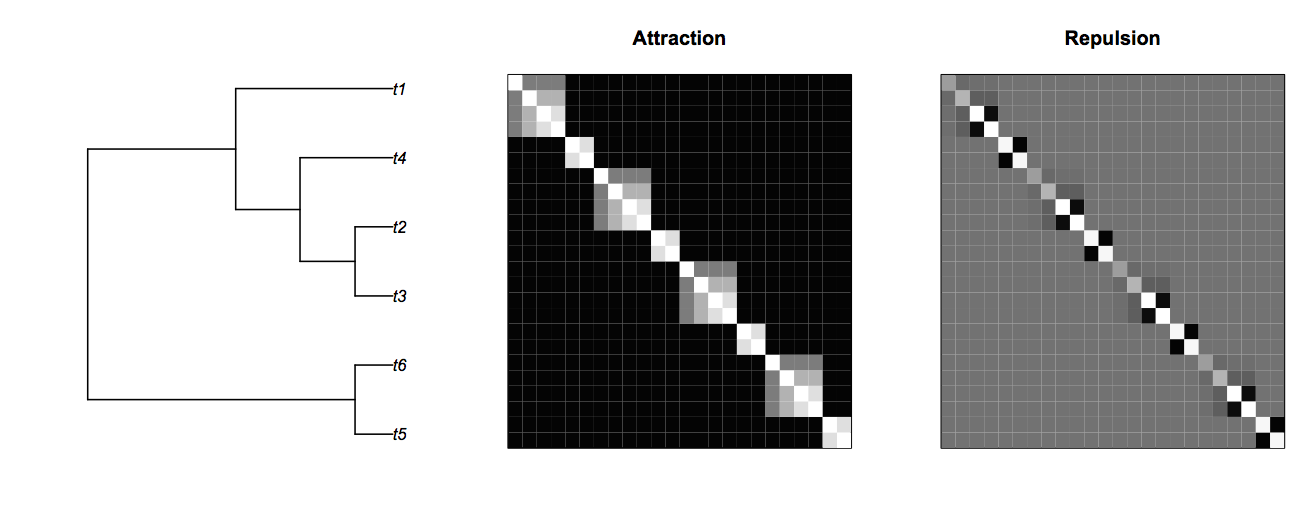Fig. 4.7: Covariance matrices for phylogenetic attraction and repulsion. The statistical challenge of detecting phylogenetic repulsion when phylogenetically related species respond similarly to an environmental factor can be shown by comparing the covariance matrices (env|sp__) and (1|sp__@site) (Fig. 4.8). The phylogenetic repulsion matrix (1|sp__@site) is the inverse of the phylogenetic attraction matrix, and the phylogenetic attraction matrix appears in blocks along the diagonal of (env|sp__) multiplied by env2. This means that the phylogenetic effects within sites in part cancel each other out, which is seen by taking the sum of (env|sp__) and (1|sp__@site) (Fig. 4.8). This acts to mask the effect of phylogenetic repulsion.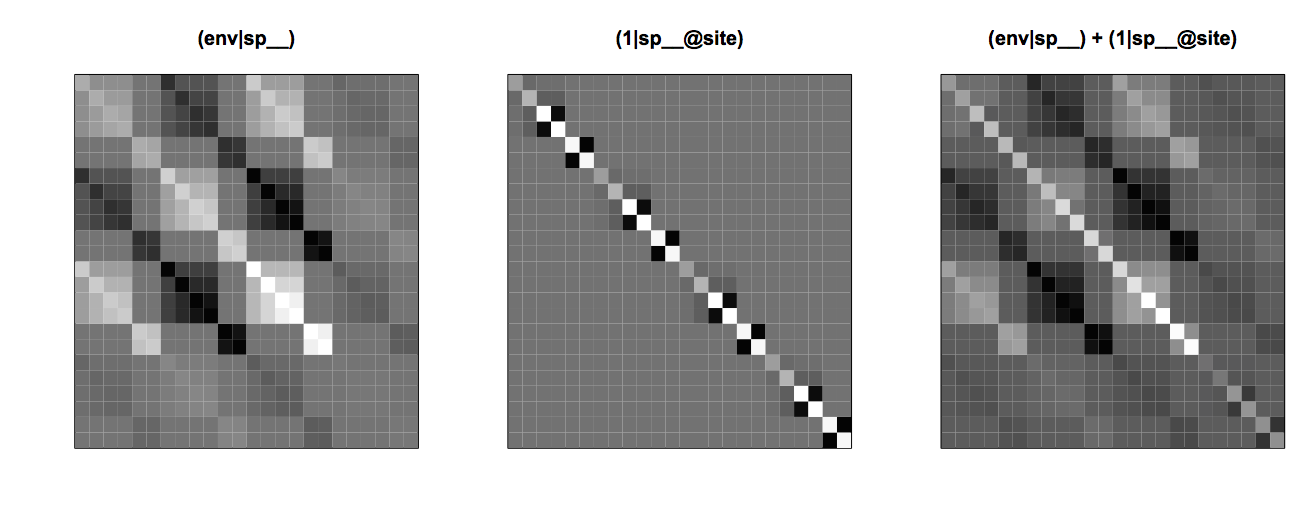Fig. 4.8: Covariance matrices for a species-specific response to the environmental factor (env|sp__), phylogenetic repulsion (1|sp__@site), and the combined effects of the environmental factor and phylogenetic repulsion. #### 4.4.2. Testing for phylogenetic repulsion A test for phylogenetic repulsion can be performed with a LRT comparing the communityPGLMM() models with and without (1|sp__@site). For the case of 20 species and 15 sites, even relatively weak phylogenetic repulsion (sd.repulse <- 0.5) is generally identified as highly significant. For a specific simulation, the LRT test gave P = 0.007. To see how phylogenetically correlated species-specific responses to env can obscure phylogenetic repulsion, I fit the same simulated example without env: In this case, the LRT gave P = 0.21, so no phylogenetic repulsion was detected. The huge improvement in detecting phylogenetic signal by including env doesn’t always happen, but it is more likely than not; you can use the R code to simulate more examples. I have used the standard LRT with the chi-square approximation in this example, although it is possible to perform a parametric bootstrap LRT under H0 of no phylogenetic repulsion. However, because I know that the LRT is conservative from section 4.3 (and from other simulations) and gives P-values that are if anything too high, and because I’m not worried about power, I’ve only used the time-saving standard LRT. ### 4.5 Can traits explain phylogenetic patterns? If phylogenetic correlations among species trait values are responsible for phylogenetic attraction of species among sites, then incorporating information about these traits into a PLMM should remove any phylogenetic attraction. This intuitive conclusion was largely supported by analyses of two real datasets by Li et al. (2017). For the dataset with the greatest information about trait values, we showed that including these values in a PLMM explained all of the phylogenetic attraction in the data. For the second dataset, trait values explained most but not all of the phylogenetic signal, although in the second dataset the number of traits available was limited. To show how trait information can explain phylogenetic attraction, I simulated data using three traits that determine the response of species to three environmental variables that are distributed independently among sites. Although the data are simulated knowing information about environmental variables among sites, I assume that environmental information is not available when analyzing the data. In the simulation of species abundances Y, c1*env1 makes the mean abundance of species in a given site depend on the value of env1 in that site, with the coefficient c1 determining the strength of this affect. The term f1*dtrait1*env1 gives the species-specific response to env1 that depends on the value of trait1, scaled by the coefficient f1. Thus, f1*d$trait1 can be thought of the slope of species abundances with respect to env1, with each species having a different slope depending on their value of trait1. I fit the simulated data using two models: the model for phylogenetic attraction presented in section 4.3, and a model that includes the trait information available for the species. The first model for phylogenetic attraction has terms (1|sp__) to account for non-phylogenetic and phylogenetic variation in mean species abundances, (1|site) to account for differences in mean species abundances among sites, and (1|sp__@site) for phylogenetic attraction. The second model has the same terms plus terms (trait|site) for each trait. These terms imply that each site may favor species with different values of trait. If the value of env were known for each site, then it would be possible to include a trait-by-environment interaction trait:env as a fixed effect in the model; this term was used in the simulation to generate phylogenetic responses of species to environmental factors. However, since we assume that env is not known in the dataset, the model specifies only that sites differ in some unknown way that selects differently for species depending on their value of trait. I also included fixed effects for each trait, since traits are also included as slopes in the random effect (trait|site). The reason to include trait fixed effects is that they give the mean effect of trait for each species and serve the same role as a main effect in a regression model that includes interaction terms: if interaction terms are in a model, so too should be the corresponding main effects for each term in the interaction. For both models I tested the significance of phylogenetic signal given by (1|sp__@site) using a standard LRT. The results of the fits show that incorporating trait information explains the phylogenetic attraction. In the first model without trait values, the random effect (1|sp__@site) is highly significant (P < 10^-15), while in the second model it is not (P = 0.88). Thus, the trait values in the second model absorbed the variation that was attributed to phylogenetic attraction in the first model. This is only a single simulated example, but you can use the code to see that this is a common result. Also, note that the P-value for (1|sp__@site) differed so much between models, I was not very concerned about the possible loss of power by not performing a bootstrap LRT under H0. The consequences of adding trait information on phylogenetic attraction can be seen by investigating the covariance matrices and comparing them to the trait data. A simulation for six species and four sites produced the dataset: Covariance matrices for this dataset are given in figure 4.9. Each of the covariance matrices for the trait random effects (trait|site) contains the phylogenetic covariances among species, but they vary in their weightings. For example, species t3 (the second species in each site) has the highest value of trait1 and therefore has the highest variance in the covariance matrix (trait1|site). Species t3 is related to t2 and t4 (taking positions 2, 1, and 3 in each community), so there are phylogenetic covariances between these species seen as 3 x 3 blocks of nine cells in the covariance matrix. Because the values of trait1 are low for the other three species (taking positions 4, 5, and 6), they don’t exhibit strong positive covariances. Covariance matrices (trait2|site) and (trait3|site) similarly have phylogenetic covariances, but weighted differently from (trait1|site) due to the different distribution of trait values among species. The sum of (trait1|site), (trait2|site) and (trait3|site) averages over the specific weights of species, and the resulting covariance matrix is similar to the matrix (1|sp__@site) giving phylogenetic attraction. This explains why, in the model with trait values analyzed above, there is little phylogenetic attraction detected; the phylogenetic covariance in species abundances within sites is accounted for by the combined random effects of traits within sites.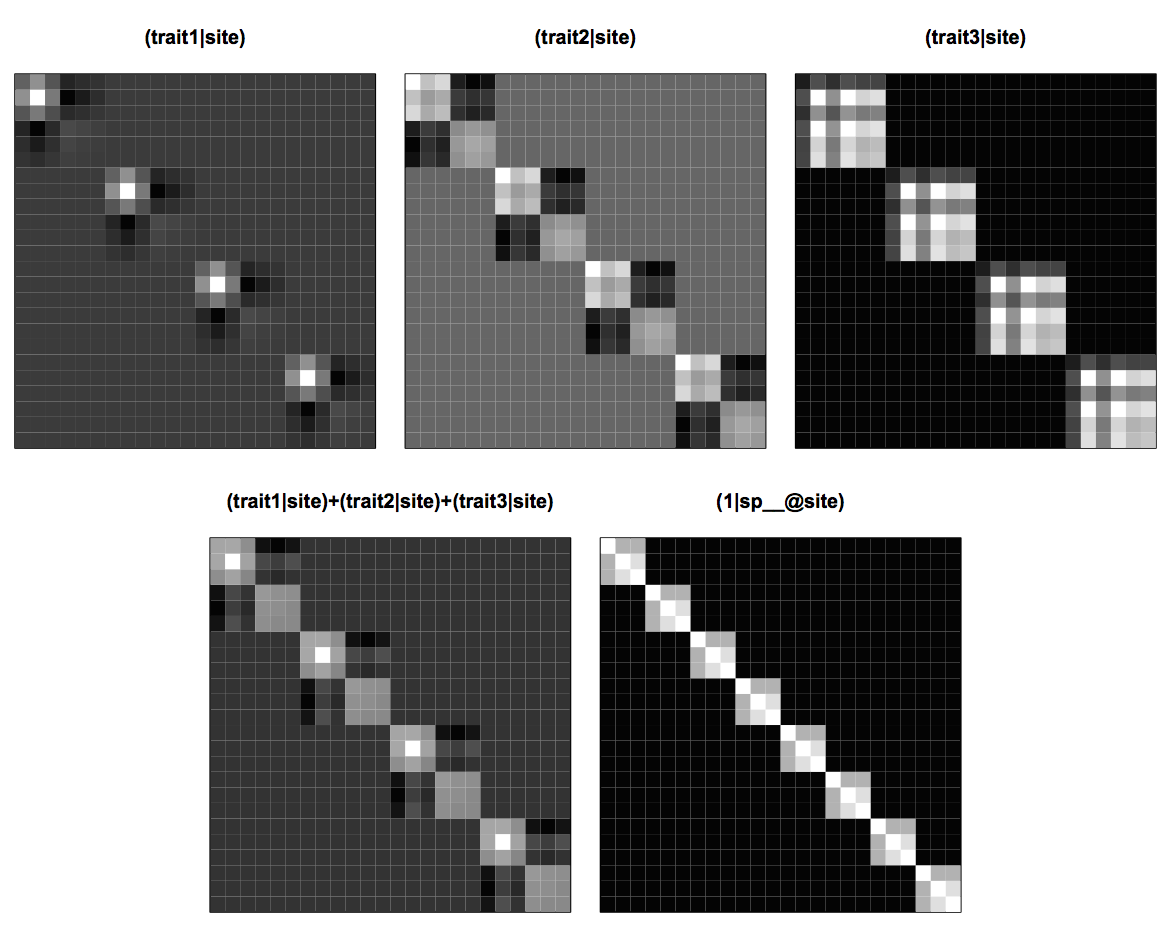Fig. 4.9: Covariance matrices for a site-specific selection of trait values, (trait1|site), (trait2|site) and (trait3|site), the sum of all three of these matrices, and the phylogenetic attraction matrix (1|sp__@site). It is not necessary to have information on all trait values for traits to absorb some of the phylogenetic attraction in the model. In other words, even information on just a single trait might absorb a lot of the phylogenetic attraction that is observed without information about this trait. I’ve left you to explore this as an exercise. ### 4.6 Trait-by-environment interactions In searching for phylogenetic repulsion (section 4.4), I assumed information was available for environmental factors, and in asking for explanations of phylogenetic attraction (section 4.5), I assumed trait information was available. If both trait and environmental information are available, then it should be possible to explain all patterns in the data without the need for a phylogeny. This assumes, however, that all traits and environmental factors are known. If they are not, then unknown traits and environmental factors can produce correlations in the data. Ignoring these correlations is like ignoring hierarchical correlations (Chapter 2) or phylogenetic correlations in comparisons among species (Chapter 3): inflated type I errors and low power. To illustrate these issues, I’ll set up and analyzed the problem investigated by Li and Ives (2017). Assume that there are two traits, trait1 and trait2, and two environmental factors env1 and env2. Values of trait1 and env1 are known, while trait2 and env2 are unknown. The mean abundances of species across sites depend on both traits, and the mean abundances of species within sites depend on both environmental factors. Finally, both trait1 and trait2 affect the response of species to env1. The reason for having trait2 affect species responses to env1 is to generate phylogenetic signal in species responses to env1 that is not due to trait1. This situation is likely in real systems, since multiple traits are generally involved in species adaptations to environmental factors, and it is often likely that some of these traits are unknown. The code to simulate the data is The statistical question is whether the trait-by-environment interaction trait1:env1 is different from zero, which corresponds to the coefficient f11 in the simulation. The statistical challenge is that there is phylogenetic signal in the data. Specifically, we know from the simulations used to produce the data that there is phylogenetic signal in the response of species to env1 that is not due to trait1. This can be included in the model with a term (env1|sp__) which corresponds to f21*trait2*d$env1 in the simulation. Similarly, there is also phylogenetic signal in the mean abundances of species caused by trait2 which can be included in the model with (1|sp__); this corresponds to b2*trait2 in the simulation. If phylogenetic signal is not included, then the corresponding terms are (env1|sp) and (1|sp). Thus, the two models needed to see the effects of incorporating phylogenetic signal on the statistical ability to detect the trait-by-environment interaction trait1:env1 are

Note that the second model is just a non-phylogenetic LMM that accounts for the hierarchical structure of the data but not phylogenetic correlations. Therefore, it could be fit with lmer() from the package lme4.

The phylogenetic covariances are expected to create problems for type I errors and/or statistical power. These problems can be anticipated by comparing the effect of the interaction term trait1:env1 on species abundances with the covariance matrix for (env1|sp__). The left panel of figure 4.10 gives the squared errors in species abundances that are attributable to the trait1:env1 interaction; each element in the matrix gives the product of trait1(i) * trait1(j) * env1(k) * env1(l) for species i and j, and sites k and l. These are thus the fixed effects equivalent to the covariance matrix for the random effects. The similarity between the matrix for trait1:env1 and (env1|sp__) shows that statistically it will be difficult to distinguish the effect of the trait1:env1 interaction from phylogenetic signal in the effect of env1 that occurs in the covariances of the residual variation. This likely leads to the two problems found for phylogenetic analyses in section 3.6.2 (Fig. 3.9). First, for the correctly specified model with (env1|sp__), this will likely lead to a decrease in power to detect a significant trait1:env1 interaction compared to the case when there were no phylogenetic signal in the effect of env1. Second, for the mis-specified model that ignores the phylogenetic signal in the effect of env1, the true phylogenetic signal from env1 will falsely be attributed to the trait1:env1 interaction and hence lead to inflated type I errors when there is phylogenetic signal in trait1.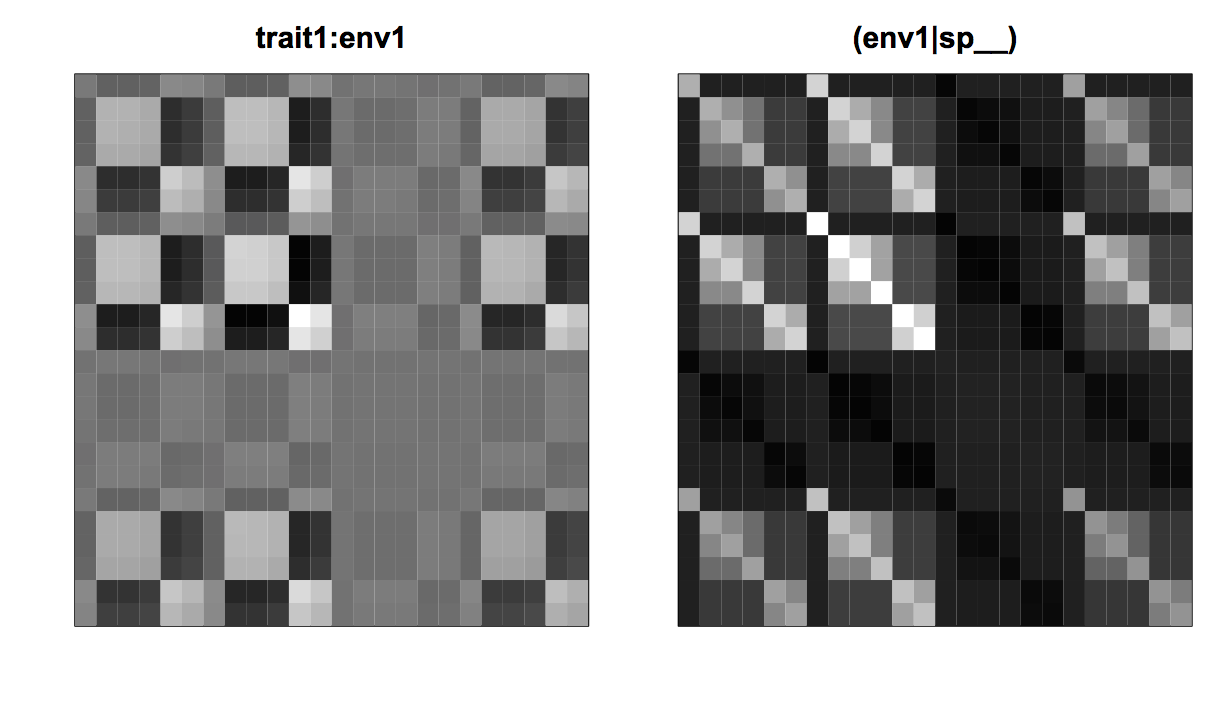Fig. 4.10: Covariance matrices for the phylogenetic model for trait-by-environment interactions showing the effect of the fixed effect trait1:env1 on the squared residuals and the covariance matrix for (env1|spp__).

I tested these anticipated problems with type I errors and power for the non-phylogenetic LMM as done in Li and Ives (2017). I generated power curves for the cases in which trait1 either didn’t or did have phylogenetic signal. These correspond to the cases presented for phylogenetic comparative methods in which power curves for a regression coefficient were generated when the associated x variable either didn’t or did have phylogenetic signal (section 3.6.2, Fig. 3.9). Failing to account for phylogenetic signal using the term (env1|sp__) had the anticipated effects. First, when there was no phylogenetic signal in trait1, the LMM that did not include the phylogeny had poor statistical power (Fig. 4.11, left panel). Second, when there was phylogenetic signal in trait1, the LMM that did not include the phylogeny had inflated type I errors (Fig. 4.11, right panel).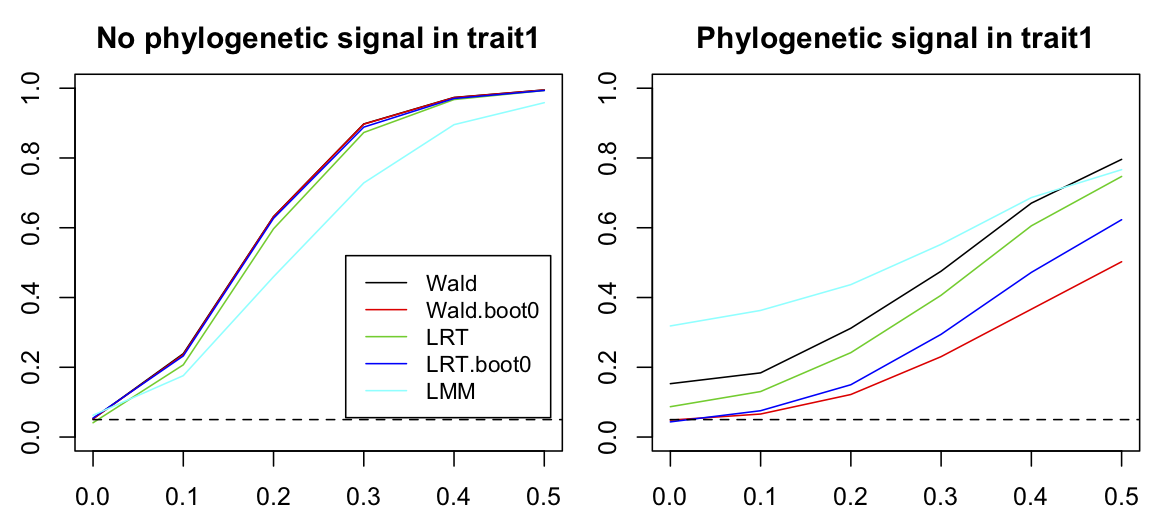Fig. 4.11: Power curves for tests of the trait1:env1 interaction in a PLMM. Datasets of 20 species distributed among 15 sites were simulated assuming that trait1 didn’t (left panel) or did (right panel) have phylogenetic signal. For each level of f11 = 0, 0.2, 0.4, 0.6, 0.8 and 1.0, 2000 simulations were performed and the rejection rates calculated for communityPGLMM() using a Wald test, a Wald test bootstrapped under H0, a LRT test, and a LRT test bootstrapped under H0. Finally, a non-phylogenetic LMM was fit to the data. The other parameters used to simulate the data are b0 = 0, b1 = 1, b2 = 1, c1 = 1, c2 = 1, f21 = 0.5, sd.env = 1, sd.trait = 1, and sd.e = 1.

In addition to showing the importance of including phylogenetic information, figure 4.11 also compares different methods for computing P-values for regression coefficients in the PLMM. P-values can be computed by either Wald tests or LRTs, and I also performed both tests by bootstrapping the distributions of Z scores (Wald test) and LLRs (LRT). When there is no phylogenetic signal in trait1, all methods had good type I errors and almost identical power. However, when there was phylogenetic signal in trait1, the Wald test and LRT both had inflated type I errors, especially the Wald test (although not as bad as the LMM). As should be the case, the parametric bootstrap tests around H0 had correct type I errors, and the bootstrapped LRT had slightly greater power. The inflated type I errors for the non-bootstrap tests means that a parametric bootstrap should be performed.

### 4.7 Bipartite phylogenetic patterns

So far we have assumed that only species have phylogenetic patterns in their distributions among sites. For problems such as the association between pollinators and plants, or disease and hosts, the sites used by pollinators or diseases have a phylogeny as well. For example, closely related pollinator species might be more likely to feed from phylogenetically related plant species, or two closely related host species might be more susceptible to the same disease. Adding a phylogeny for the site introduces nothing new statistically, although it does add more patterns to understand and analyze.

In this section, I’ll first illustrate the problem of identifying phylogenetic attraction for both species and sites. I’ll then ask whether phylogenetic attraction among sites (closely related sites being more likely to be associated with closely related species) can be statistically attributed to trait differences among sites. Both scenarios are motived by the study in Rafferty and Ives (2013) that investigated the patterns of association of pollinator visits to plants, and whether visitation patterns among plants were explained by similarities among their trait values. I’ll continue to use “sites” for one of the groups of species in the bipartite data; this makes it easier to integrate the discussion into the rest of the chapter. But throughout I’ll assume that species are pollinators, sites are plants, trait refers to pollinator traits, and env refers to plant traits.

#### 4.7.1 Phylogenetic attraction for species and sites

A simulation for the distribution of species among sites including phylogenetic attraction for both species and sites is

The code first simulates mean species abundances from the species phylogeny, phy.sp, and the mean abundance of species in each site from the site phylogeny, phy.site. These are expanded to the site-species data points and saved in the arrays Y.sp and Y.site. The code then creates the phylogenetic covariance matrices for phylogenetic attraction for species, sites, and the species-site interaction, Vphy.sp, Vphy.site, and V.sp.site; I’ll show the structure of these matrices below. From these covariance matrices, site-species data points are generated and saved in the arrays Y.sp.attract, Y.site.attract, and Y.sp.site.attract. Finally, random error is generated, and all arrays are added together to make the simulated dataset.

The covariance matrices can be visualized using communityPGLMM.plot.re(). Figure 4.12 shows the covariance matrices for the case of six species (pollinators) and four sites (plants). Phylogenetic attraction for species is the same as shown previously in figure 4.3: closely related species are more likely to have high or low abundances within the same site as given by (1|sp__@site). Phylogenetic attraction has the same structure for the sites: closely related sites are more likely to contain high or low abundances of the same species as given by (1|sp@site__). The new phylogenetic attraction covariance matrix, (1|sp__@site__), describes the pattern in which closely related species are more likely to occur in closely related sites. This is produced by tiling the species covariance matrix (seen in (1|sp__@site)) across the site-species covariance matrix, with each tile weighted by the site covariance matrix (seen in (1|site__)).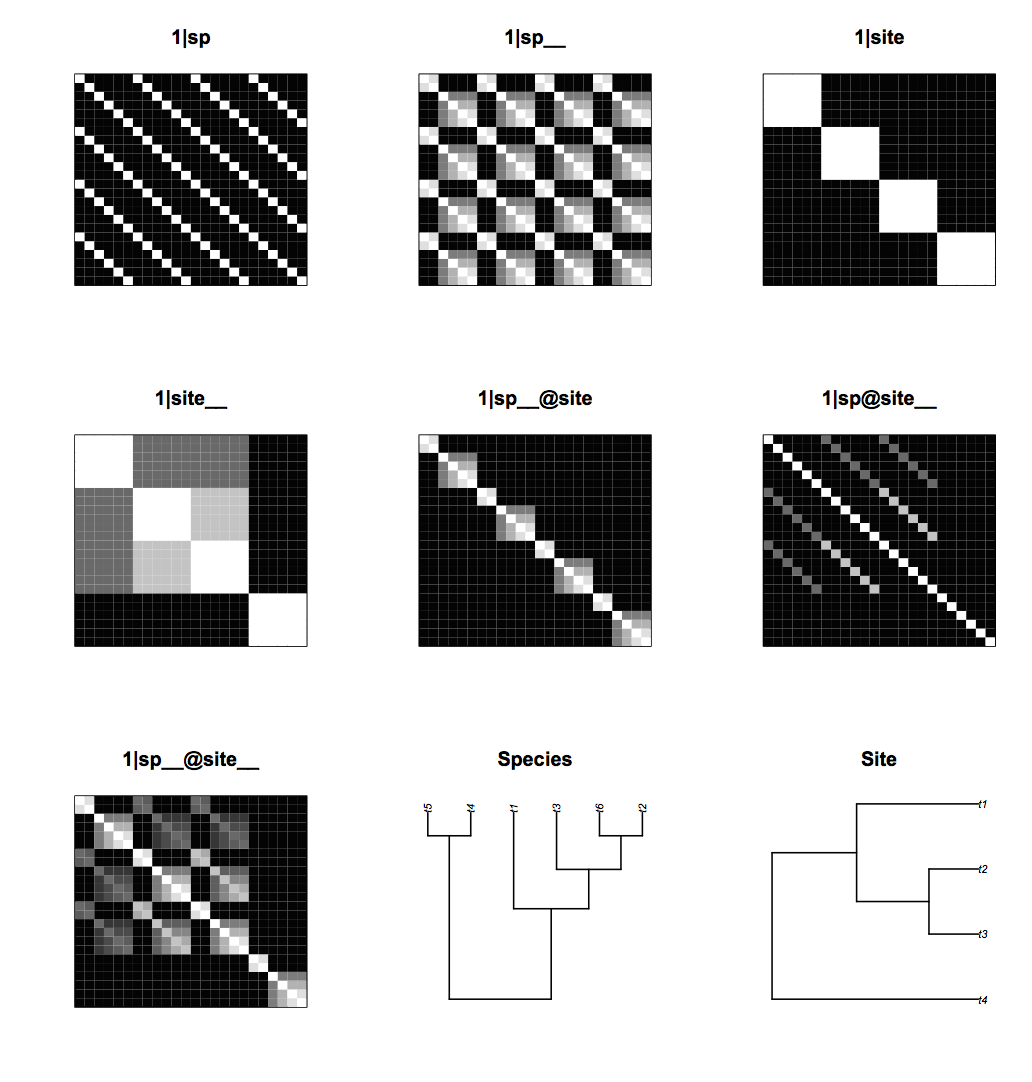Fig. 4.12: The covariance matrices for the random effects for the bipartite model generated from communityPGLMM.plot.re(). The figure also shows the species and site phylogenies.

Another way to visualize the covariance structure of the model is to simulate data from the covariance matrices using communityPGLMM.plot.re(); figure 4.13 shows an example with 20 species and 15 sites. The phylogenetic attraction of species within sites, (1|sp__@site), generates sections of rows corresponding to related species that are all high or low, showing related species having either high or low abundances within the same site. Similarly, the phylogenetic attraction of sites to contain the same species, (1|sp@site__), generates sections of columns corresponding to the same species that have high or low abundance in related sites. Finally, (1|sp__@site__) contains blocks in which related species in related sites have high or low abundances.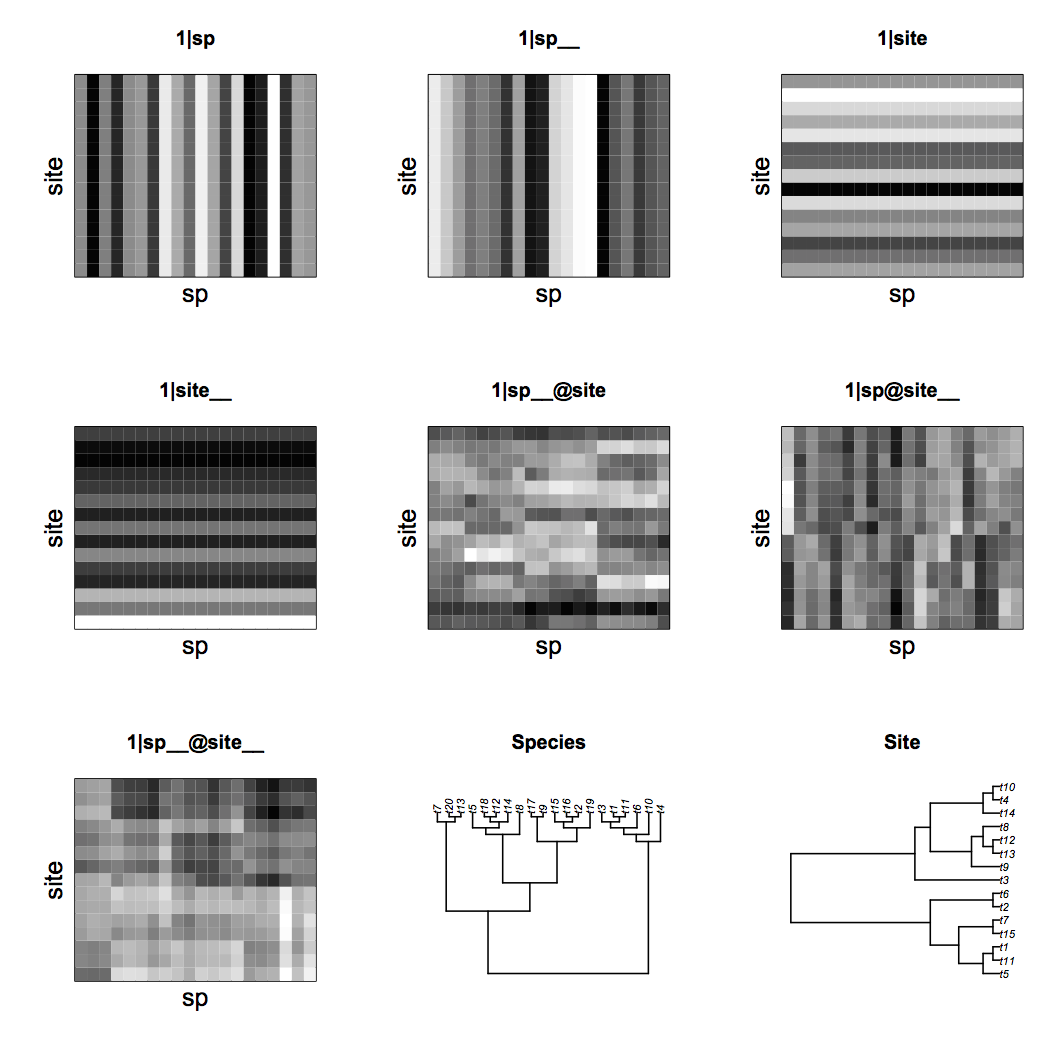Fig. 4.13: Simulations with 20 species and 15 sites to show the structure of the covariance matrices for the random effects for the bipartite model generated from communityPGLMM.plot.re(). The figure also shows the species and site phylogenies.

These simulated data can be fit by including all seven random effects in communityPGLMM(). Statistical tests for significance of the random effects are performed with LRTs. Because there are seven random effects, there may be problems with finding the true ML or REML parameter values for the random effects. Random effects are numerically challenging, because they have a boundary at zero. Therefore, the optimizer used to find the parameter values that give the highest likelihoods might have to walk up along this zero boundary, and most optimizers have a difficult time doing this. Therefore, it is worth using a few tricks. One is to add random effects one at a time, and use the estimated random effects terms from each model as starting values for the following model. For the LRT, I started with the reduced model and added the random effect that is the subject of the statistical test, in this case (1|sp__@site__). To fit the full model, I used the variances of the random effects terms estimated by the reduced model c(mod.r\$ss, .01)^2 as the starting values for s2.init in the full model, with the value of s2.init for (1|sp__@site__) that is not in the reduced model set to 0.012.

An example for this analysis for a simulated dataset is

In this simulation, the P-value for (1|sp__@site__) from the LRT was very low, implying a strong interaction between the species and site phylogenies: phylogenetically related species were more likely to have high abundances in phylogenetically related sites. Because the P-value is so low, I felt safe not performing a parametric bootstrap LRT.

#### 4.7.2 Do traits explain attraction?

Patterns of phylogenetic attraction in community composition are likely caused by traits that have phylogenetic signal. Therefore, if information about these traits is known, then it should be possible to explain the phylogenetic attraction (section 4.5). Here, I consider the scenario in which plants (sites) have traits (environmental factors) env1 and env2 that are known in the dataset and have phylogenetic signal among plants. This could be the case, for example, if flowers from related plant species have characteristics that lead to greater visits of pollinator species, as found by Rafferty and Ives (2013).

Assume that for the two plant traits env1 and env2, there are two pollinator traits, trait1 and trait2, such that pollinator (species) abundances depend on the interactions trait1:env1 and trait2:env2. This could occur if env1 were the depth of the flower corolla and trait1 were the length of a bee’s tongue. The interaction trait1:env1 would occur if plants with long corollas (high env1) were visited more often by bees with longer tongues (high trait1). Data for this situation are simulated as

The simulation does in fact produce strong species-by-site phylogenetic attraction, as given by the model investigated in the previous subsection 4.7.1.

To see if information about the site traits env1 and env2 can explain this phylogenetic attraction, a PLMM can be fit that includes (env1|sp__) and (env2|sp__). These random effects allow env1 and env2 to have different effects on each species. Since we don’t know the values of trait1 or trait2, we can’t include explicit information about species traits. Nonetheless, (env1|sp__) and (env2|sp__) allow for phylogenetic signal in the responses of species to env1 and env2. The results of this model fitted to the same dataset at above are

In the PLMM including (env1|sp__) and (env2|sp__), the P-value for the random effect in question, (1|sp__@site__), was was not significant, implying that this phylogenetic pattern is largely explained by env1 and env2.

### 4.8 Binary (presence/absence) data

In this chapter so far, I have considered only continuous data for Y. Nonetheless, communityPGLMM() can also analyze binary Y for presence/absence data. For this, it uses the standard GLMM formulation. For example, for the simple case of a single fixed effect and single random effect with phylogenetic signal, the binomial PGLMM is

Z = b0 + b1*x + e[phy]

p = inv.logit(Z)

Y ~ binom(n, p)

e ~ norm(0, s2*V[phy])

This model can be specified using communityPGLMM() as

The only difference between this model and the full model in subsection 4.3.2 is the family='binomial' statement, and the specification of the optimizer as Nelder-Mead (which I find is often good at solving problems involving boundaries at zero). All of the analyses presented earlier in this chapter for continuous abundances can be analyzed for presence/absence by setting family = "binomial" in communityPGLMM(). To give an example, I repeated the analyses from subsection 4.3.2 of phylogenetic attraction by performing a power analysis.

Figure 4.14 shows the comparable results to figure 4.5.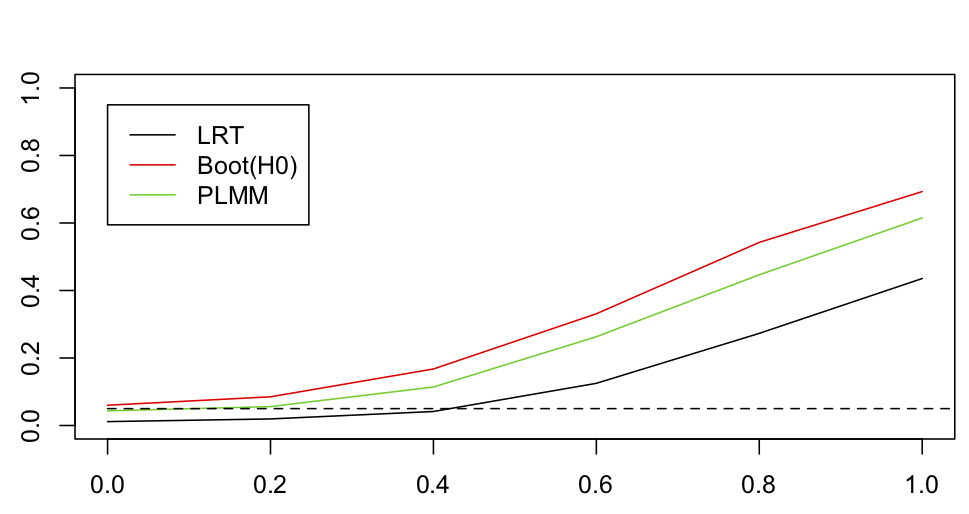Fig. 4.14: Power curves for tests of phylogenetic attraction with binary data. For each set of 2000 simulated datasets at values of sd.attract = 0, 0.2, 0.4, 0.6, 0.8 and 1, the significance of sd.attract was tested with a standard LRT (LRT) and with a parametric bootstrap LRT under H0 (Boot(H0)). Significance was also tested by fitting a PLMM that includes the same random effects but assumes the data are continuous (PLMM).

This analysis shows that the standard LRT had lower power than the LRT bootstrapped under H0. This parallels the result found for continuous data (Fig. 4.5), although the loss of power is more substantial. I also performed the test with a PLMM that has the same phylogenetic structure as the binary PGLMM but treats that data as if they were continuous. The PLMM had good type I error control although had slightly lower power than the bootstrap LRT of the binary PGLMM. This is not surprising given the performance we have seen for LMs and LMMs fit to binary data in previous chapters. This result is encouraging, because fitting PLMMs is much less computationally intensive than fitting PGLMMs for binary data.

There are numerous technical issues surrounding communityPGLMM() with family="binomial" that is outlined in the documentation. In particular, as discussed for binaryPGLMM() in section 3.8 (which uses the same algorithms), the binary implementation of communityPGLMM() uses a conditional REML approach and does not give the overall likelihood. Therefore, true LRTs are not given. Instead, the LRT is performed with communityPGLMM.binary.LRT() which is a LRT on the random effects conditional on the fixed effects. This approach is compatible with the REML structure of the fitting, but it is nonetheless not a complete LRT.

### 4.9 Flexibility and caveats for phylogenetic GLMMs

Throughout this chapter, I’ve used the standard phyr syntax of communityPGLMM() in which random effects are structured as in lmer(). This limits terms like (1|sp__) to have phylogenetic attraction, and nested terms like (1|sp__@site) to have either phylogenetic attraction or phylogenetic repulsion (setting repulsion=T). Mathematically, however, there are no constraints on the covariance matrices that can be incorporated. Therefore, communityPGLMM() allows you to structure covariance matrices and use them as random effects. You could make, for example, spatial covariance matrices instead of or in addition to phylogenetic covariance matrices. To see how to construct your own covariance matrices, see the documentation for communityPGLMM().

The largest limitation of communityPGLMM() is that fitting large datasets is slow. For example, the model of phylogenetic attraction (subsection 4.3.2) with 50 species and 100 sites took 17 minutes to run on an old and slow Macintosh. This was a model with a nested covariance matrix, and for mathematical reasons these models will be slower than models without nested covariance matrices. Nonetheless, models with large numbers of random effects applied to datasets with hundreds of species and sites will tax computers. Fitting binary data with family="binomial" is slower still, in some cases by more than an order of magnitude.

There are tricks that can help. Random effects can be added in a forward selection style, with starting values for parameters taken from the previous model. Also, I wouldn’t be shy about using family="gaussian" (the default) for binary data, provided I did simulations to check type I errors. There are also hybrids possible. For example, a model could be fit with family="binomial", and data simulated by this model used to create a parametric bootstrap LRT for the model fit with family="gaussian".

In short, if you have large datasets and questions that involve many random effects, you will need to think about how best to implement the models. It is also possible to try methods based on metrics rather than models. But here I would recommend exploring the metrics carefully and comparing them to PGLMMs for simulated data or subsets of your large dataset. This will be the best way to understand what patterns the metrics are really picking up in your data, which might not be the patterns you are interested in.

### 4.10 Summary

1. Phylogenetic models of community composition combine approaches for dealing with data having a hierarchical structure, and phylogenetic approaches for dealing with phylogenetic covariances among species. Thus, PGLMMs incorporate two types of correlations in data.
2. PGLMMs can explain the distribution of species among communities using only information about phylogenies. However, these models can also flexibly include information about traits shared by species and environmental factors shared by communities.
3. Hierarchical and phylogenetic correlations present the same challenges as hierarchical data (Chapters 1 and 2) and phylogenetic data (Chapter 3). In tests of fixed effects, failing to account for the correlated structure of the data often leads to inflated type I errors (false positives) or reduced power (false negatives). Problems with type I errors are especially bad when the fixed effects themselves have phylogenetic signal. For tests of random effects, the main problems are deflated type I errors and low statistical power. These are less serious than the problem of inflated type I errors in tests for fixed effects.
4. Phylogenetic community models can be used to test which traits and which environmental factors are responsible for phylogenetic patterns in the data. They give statistical tests for why the phylogenetic patterns exist.
5. Although PGLMMs provide flexible tools for testing a wide range of hypotheses in a diverse collection of community ecology data, there are limits to the size of datasets they can reasonably handle. For complex hypotheses involving many covariance patterns, fitting PGLMMs with more than 1000-2000 species-site data points will be computationally slow.

### 4.11 Exercises

1. Section 4.5 investigated whether information about traits could explain phylogenetic attraction in data. In the simulations, three traits and three environmental factors were used to create phylogenetic patterns. In testing whether the phylogenetic attraction could be explained by the trait values, all three traits were included in the model. Investigate whether information about only a single trait, or about only two traits, could explain the phylogenetic attraction. What do the results imply for using this approach to try to identify the existence of traits that underlie phylogenetic attraction in datasets?

### 4.12 References

Helmus, M. R., K. Savage, M. W. Diebel, J. T. Maxted, and A. R. Ives. 2007. Separating the determinants of phylogenetic community structure. Ecology Letters 10:917-925.

Ives, A. R., and M. R. Helmus. 2011. Generalized linear mixed models for phylogenetic analyses of community structure. Ecological Monographs 81:511-525.

Li, D., and A. R. Ives. 2017. The statistical need to include phylogeny in trait-based analyses of community composition. Methods in Ecology and Evolution 8:1192-1199.

Li, D., A. R. Ives, and D. M. Waller. 2017. Can functional traits account for phylogenetic signal in community composition? New Phytologist.

Rafferty, N. E., and A. R. Ives. 2013. Phylogenetic trait-based analyses of ecological networks. Ecology 94:2321-2333.